# JP2014103686A - Image processing device and method, and recording medium - Google Patents

## Info

Publication number
JP2014103686A
JP2014103686A JP2014003300A JP2014003300A JP2014103686A JP 2014103686 A JP2014103686 A JP 2014103686A JP 2014003300 A JP2014003300 A JP 2014003300A JP 2014003300 A JP2014003300 A JP 2014003300A JP 2014103686 A JP2014103686 A JP 2014103686A
Authority
JP
Japan
Prior art keywords
unit
image
intra prediction
filter
prediction
Prior art date
Legal status (The legal status is an assumption and is not a legal conclusion. Google has not performed a legal analysis and makes no representation as to the accuracy of the status listed.)
Granted
Application number
JP2014003300A
Other languages
Japanese (ja)
Other versions
JP5733587B2 (en
Inventor
Kazufumi Sato

Original Assignee
Sony Corp
ソニー株式会社
Priority date (The priority date is an assumption and is not a legal conclusion. Google has not performed a legal analysis and makes no representation as to the accuracy of the date listed.)
Filing date
Publication date
Priority to JP2009156563 priority Critical
Priority to JP2009156563 priority
Application filed by Sony Corp, ソニー株式会社 filed Critical Sony Corp
Priority to JP2014003300A priority patent/JP5733587B2/en
Publication of JP2014103686A publication Critical patent/JP2014103686A/en
Application granted granted Critical
Publication of JP5733587B2 publication Critical patent/JP5733587B2/en
Application status is Active legal-status Critical
Anticipated expiration legal-status Critical

## Images

••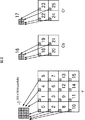•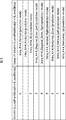•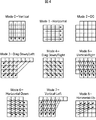•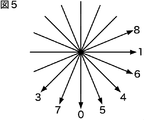••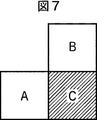•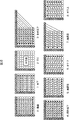•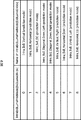•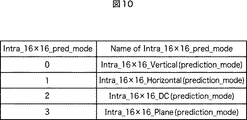•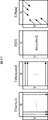••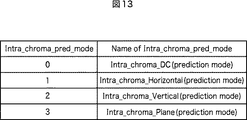•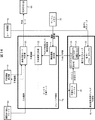•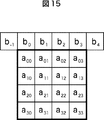•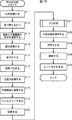•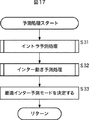•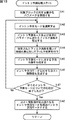•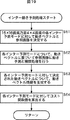•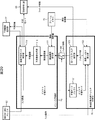•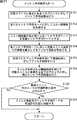•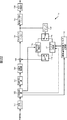•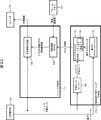•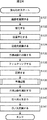•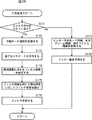•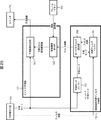•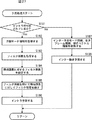•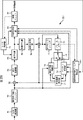•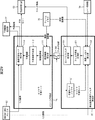•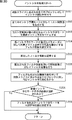•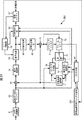•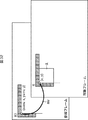•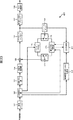•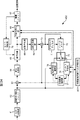•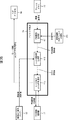••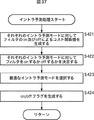•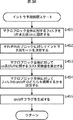•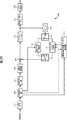•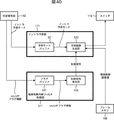••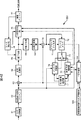•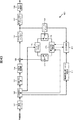•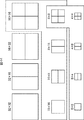•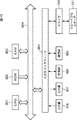••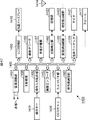•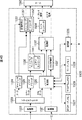•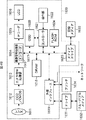## Abstract

PROBLEM TO BE SOLVED: To enable noise removal depending on an image or a bit rate.SOLUTION: A low-pass filter setting part 93 sets a filter coefficient corresponding to intra-prediction mode information and a quantization parameter among filter coefficients stored in an incorporated filter coefficient memory 94. An adjacent pixel setting part 81 performs filter processing to an adjacent pixel value of a target block from a frame memory 72 by using the filter coefficient set by the low-pass filter setting part 93. A prediction image generation part 82 performs intra-prediction by using the adjacent pixel value subjected to the filter processing from the adjacent pixel setting part 81 to generate a prediction image. This invention can be applied to an image encoding device encoding by an H.264/AVC system, for example.

## Description

The present invention relates to an image processing apparatus and method, a program, and a recording medium, and in particular, an image processing apparatus and method, a program, which realizes noise removal according to an image and a bit rate and can improve prediction efficiency, And a recording medium.

In recent years, image information has been handled as digital data, and at that time, for the purpose of efficient transmission and storage of information, encoding is performed by orthogonal transform such as discrete cosine transform and motion compensation using redundancy unique to image information. An apparatus that employs a method to compress and code an image is becoming widespread. This encoding method includes, for example, MPEG (Moving Picture Experts Group).

In particular, MPEG2 (ISO / IEC 13818-2) is defined as a general-purpose image coding system, and is a standard that covers both interlaced scanning images and progressive scanning images, as well as standard resolution images and high-definition images. For example, MPEG2 is currently widely used in a wide range of applications for professional and consumer applications. By using the MPEG2 compression method, for example, a code amount (bit rate) of 4 to 8 Mbps is assigned to an interlaced scanned image having a standard resolution of 720 × 480 pixels. Further, by using the MPEG2 compression method, for example, in the case of a high-resolution interlaced scanned image having 1920 × 1088 pixels, a code amount (bit rate) of 18 to 22 Mbps is allocated. As a result, a high compression rate and good image quality can be realized.

MPEG2 was mainly intended for high-quality encoding suitable for broadcasting, but did not support encoding methods with a lower code amount (bit rate) than MPEG1, that is, a higher compression rate. With the widespread use of mobile terminals, the need for such an encoding system is expected to increase in the future, and the MPEG4 encoding system has been standardized accordingly. Regarding the image coding system, the standard was approved as an international standard as ISO / IEC 14496-2 in December 1998.

Furthermore, in recent years, for the purpose of image coding for the initial video conference, The standardization of 26L (ITU-T Q6 / 16 VCEG) is in progress. H. 26L is known to achieve higher encoding efficiency than the conventional encoding schemes such as MPEG2 and MPEG4, although a large amount of calculation is required for encoding and decoding. In addition, as part of MPEG4 activities, this H. Based on 26L, H. Standardization to achieve higher coding efficiency by incorporating functions not supported by 26L is being carried out as Joint Model of Enhanced-Compression Video Coding. As for the standardization schedule, H. H.264 and MPEG-4 Part10 (Advanced Video Coding, hereinafter referred to as H.264 / AVC).

As an extension, FRExt (including RGB, 4: 2: 2, 4: 4: 4 coding tools necessary for business use, 8x8DCT and quantization matrix specified by MPEG-2) Fidelity Range Extension) standardization was completed in February 2005. As a result, H.C. Using 264 / AVC, it became an encoding method that can express film noise contained in movies well, and it has been used in a wide range of applications such as Blu-Ray Disc (trademark).

However, nowadays, there is an increasing need for further high-compression encoding such as wanting to compress an image of about 4000 × 2000 pixels, which is four times the high-definition image. Alternatively, there is a growing need for further high compression rate encoding such as the desire to deliver high-definition images in an environment with a limited transmission capacity such as the Internet. For this reason, in the above-mentioned VCEG (= Video Coding Expert Group) under the ITU-T, studies on improving the coding efficiency are being continued.

Here, H. One of the factors that realize the higher encoding efficiency of the H.264 / AVC format than the conventional MPEG2 format is the adoption of an intra prediction method.

In the intra prediction method, nine types of 4 × 4 pixel and 8 × 8 pixel block units, and four types of 16 × 16 pixel macroblock unit intra prediction modes are defined for luminance signals. For color difference signals, four types of 8 × 8 pixel block-unit intra prediction modes are defined. The color difference signal intra prediction mode can be set independently of the luminance signal intra prediction mode.

There is a specific pattern for each intra prediction mode in how to generate such a residual after intra prediction.

As a method for eliminating such redundancy and further improving the encoding efficiency, Non-Patent Document 1 proposes the following method.

That is, in the offline processing in advance, the normal H. According to the H.264 / AVC format, intra-picture encoding processing is performed, and orthogonal transform such as Karhunen-Loeve transform is performed for each intra prediction mode for each block, and an optimal transform coefficient is calculated.

In the actual encoding process, H. Instead of the orthogonal transformation defined in the H.264 / AVC format, processing using the orthogonal transformation coefficient optimized for each mode is performed by the above-described Karhunen-Loeve transformation.

Non-Patent Document 2 also proposes a method of combining the above-described intra prediction with inter prediction.

That is, in Non-Patent Document 2, difference information is generated not only for the target block but also for adjacent pixel values around the target block for the motion vector information obtained in the inter prediction. Secondary difference information is generated by performing intra prediction between the difference information regarding the target block generated in this way and the difference information regarding adjacent pixels. Then, the generated secondary difference information is orthogonally transformed and quantized and output to the subsequent stage together with the compressed image.

Thereby, the encoding efficiency is further improved.

In addition, as described above, H.P. In the H.264 / AVC format, the macroblock size is 16 × 16 pixels. However, the macroblock size of 16 × 16 pixels is not optimal for a large image frame such as UHD (Ultra High Definition; 4000 × 2000 pixels) that is the target of the next generation encoding method.

Therefore, in Non-Patent Document 3, etc., it is also proposed to expand the macroblock size to a size of 32 × 32 pixels, for example.

"Improved Intra Coding", VCEG-AF15, ITU-Telecommunications Standardization Sector STUDY GROUP Question 6 Video coding Experts Group (VCEG), 20-21 April 2007 "Second Order Prediction (SOP) in P Slice", Sijia Chen, JinpengWang, Shangwen Li and, Lu Yu, VCEG-AD09, ITU-Telecommunications Standardization Sector STUDY GROUP Question 6 Video coding Experts Group (VCEG), 16-18 July 2008 "Video Coding Using Extended Block Sizes", VCEG-AD09, ITU-Telecommunications Standardization Sector STUDY GROUP Question 16-Contribution 123, Jan 2009

H. In the H.264 / AVC format, the low-pass filter processing of the pixel values of adjacent pixels is performed prior to performing the above-described intra prediction in units of 8 × 8 pixels. As a result, noise contained in adjacent pixels is removed and the correlation can be increased, so that higher encoding efficiency can be realized.

However, although the degree of noise is different depending on the input image, the quantization parameter value, and the intra prediction mode, H.264 is used. In the H.264 / AVC format, a low-pass filter for removing noise is fixed. That is, the low-pass filter is not optimal according to the input image, the quantization parameter value, the intra prediction mode, and the like.

H. In the H.264 / AVC format, the noise removal of the pixel values of the adjacent pixels described above is performed only in the 8 × 8 pixel block unit intra prediction mode, and has not been applied to other modes.

The present invention has been made in view of such a situation, and realizes noise removal according to an image and a bit rate and improves prediction efficiency.

An image processing apparatus according to an aspect of the present invention targets an image to be encoded according to a prediction direction when performing intra prediction on pixels of a target block to be encoded. A pixel of the target block using a filter unit that selects whether to perform filter processing on an adjacent pixel to be referred to when performing intra prediction on the pixel or not to perform filter processing on the adjacent pixel, and the adjacent pixel And an intra prediction unit that performs intra prediction on the.

The filter unit performs a filtering process on an adjacent pixel to be referred to when performing intra prediction on a pixel of the target block using a mode number for identifying an intra prediction mode, or performs a filtering process on the adjacent pixel. You can choose not to.

The filter unit performs the filtering process on the adjacent pixel by using control information for controlling whether to perform the filtering process on the adjacent pixel or not to perform the filtering process on the adjacent pixel. It is possible to select whether or not to perform filtering on the pixel.

When the filter unit has selected to perform filtering on the adjacent pixel, the filtering unit can perform filtering on the adjacent pixel using a filter operation using a 3-tap filter coefficient.

The image processing apparatus may further include an encoding unit that encodes the image using the prediction image generated by the intra prediction unit.

The bit stream is encoded in a unit having a hierarchical structure, and the decoding unit can decode the bit stream in a unit having a hierarchical structure.

The encoding unit may encode the image in units having a hierarchical structure.

The intra prediction unit can perform intra prediction with block sizes of 4 × 4 pixels, 8 × 8 pixels, 16 × 16 pixels, and 32 × 32 pixels.

According to an image processing method of one aspect of the present invention, an image processing apparatus targets an image to be encoded according to a prediction direction when performing intra prediction on a pixel of a target block to be encoded. , Selecting whether to perform filter processing on adjacent pixels to be referred to when performing intra prediction on the pixels of the target block or not to perform filter processing on the adjacent pixels, and using the adjacent pixels, the target block Intra prediction is performed on the pixels.

Using a mode number that identifies the intra prediction mode, select whether to perform filtering processing on adjacent pixels to be referred to when performing intra prediction on the pixels of the target block or not to perform filtering processing on the adjacent pixels. be able to.

Using the control information for controlling whether to perform filtering on the adjacent pixels or not to perform filtering on the adjacent pixels, the adjacent pixels are filtered, or the adjacent pixels are filtered. You can choose not to do it.

When it is selected that the adjacent pixel is to be filtered, the adjacent pixel can be filtered using a filter operation using a 3-tap filter coefficient.

The image can be encoded using a predicted image generated by the intra prediction.

The image can be encoded in units having a hierarchical structure.

Intra prediction can be performed with block sizes of 4 × 4 pixels, 8 × 8 pixels, 16 × 16 pixels, and 32 × 32 pixels.

The program according to one aspect of the present invention and the program recorded on the recording medium have a prediction direction when performing intra prediction on a pixel of a target block that is a target of encoding processing for an image to be encoded. Accordingly, a filter unit that selects whether to perform filter processing on adjacent pixels to be referred to when performing intra prediction on the pixels of the target block or not to perform filter processing on the adjacent pixels, and using the adjacent pixels Then, the computer is caused to function as an intra prediction unit that performs intra prediction on the pixels of the target block.

In one aspect of the present invention, for an image to be encoded, the pixel of the target block is determined according to a prediction direction when performing intra prediction on the pixel of the target block to be encoded. Thus, it is selected whether to perform the filtering process on the adjacent pixel to be referred to when performing the intra prediction or not to perform the filtering process on the adjacent pixel. And intra prediction is performed with respect to the pixel of the said target block using the said adjacent pixel.

Note that each of the above-described image processing apparatuses may be an independent apparatus or may be an internal block constituting one image encoding apparatus.

According to the present invention, an image can be encoded. Further, according to the present invention, it is possible to perform noise removal according to the image and the bit rate.

It is a block diagram which shows the structure of one Embodiment of the image coding apparatus to which this invention is applied. It is a figure explaining the processing order in the case of 16 * 16 pixel intra prediction mode. It is a figure which shows the kind of 4 * 4 pixel intra prediction mode of a luminance signal. It is a figure which shows the kind of 4 * 4 pixel intra prediction mode of a luminance signal. It is a figure explaining the direction of 4 * 4 pixel intra prediction. It is a figure explaining intra prediction of 4x4 pixels. It is a figure explaining encoding of the 4 * 4 pixel intra prediction mode of a luminance signal. It is a figure which shows the kind of 8x8 pixel intra prediction mode of a luminance signal. It is a figure which shows the kind of 8x8 pixel intra prediction mode of a luminance signal. It is a figure which shows the kind of 16 * 16 pixel intra prediction mode of a luminance signal. It is a figure which shows the kind of 16 * 16 pixel intra prediction mode of a luminance signal. It is a figure explaining the 16 * 16 pixel intra prediction. It is a figure which shows the kind of intra prediction mode of a color difference signal. It is a block diagram which shows the structural example of the intra estimation part of FIG. 1, and an adjacent pixel interpolation filter switching part. It is a figure explaining calculation of a filter coefficient. It is a flowchart explaining the encoding process of the image coding apparatus of FIG. It is a flowchart explaining the prediction process of step S21 of FIG. It is a flowchart explaining the intra prediction process of step S31 of FIG. It is a flowchart explaining the inter motion prediction process of step S32 of FIG. It is a block diagram which shows the other structural example of the intra estimation part of FIG. 1, and an adjacent pixel interpolation filter switching part. It is a flowchart explaining the other example of the intra prediction process of step S31 of FIG. It is a block diagram which shows the structure of one Embodiment of the image decoding apparatus to which this invention is applied. It is a block diagram which shows the structural example of the intra estimation part of FIG. 22, and an adjacent pixel interpolation filter switching part. It is a flowchart explaining the decoding process of the image decoding apparatus of FIG. It is a flowchart explaining the prediction process of step S138 of FIG. It is a block diagram which shows the other structural example of the intra estimation part of FIG. 22, and an adjacent pixel interpolation filter switching part. It is a flowchart explaining the other example of the prediction process of step S138 of FIG. It is a block diagram which shows the structure of one Embodiment of the learning apparatus to which this invention is applied. It is a block diagram which shows the structural example of the intra estimation part of FIG. 28, and an adjacent pixel interpolation filter calculation part. It is a flowchart explaining the intra prediction process by the learning apparatus of FIG. It is a block diagram which shows the structure of other embodiment of the image coding apparatus to which this invention is applied. It is a figure explaining secondary prediction processing. It is a block diagram which shows the structure of other embodiment of the image decoding apparatus to which this invention is applied. It is a block diagram which shows the structure of further another embodiment of the image coding apparatus to which this invention is applied. It is a block diagram which shows the structural example of the intra estimation part of FIG. It is a flowchart explaining the other example of the intra prediction process of step S31 of FIG. It is a flowchart explaining the further another example of the intra prediction process of step S31 of FIG. It is a flowchart explaining the other example of the intra prediction process of step S31 of FIG. It is a block diagram which shows the structure of other embodiment of the image decoding apparatus to which this invention is applied. It is a block diagram which shows the structural example of the intra estimation part of FIG. 39, and an adjacent pixel interpolation filter control part. It is a flowchart explaining the further another example of the prediction process of step S138 of FIG. It is a block diagram which shows the structure of other embodiment of the image coding apparatus to which this invention is applied. It is a block diagram which shows the structure of other embodiment of the image decoding apparatus to which this invention is applied. It is a figure which shows the example of the expanded block size. It is a block diagram which shows the structural example of the hardware of a computer. It is a block diagram which shows the main structural examples of the television receiver to which this invention is applied. It is a block diagram which shows the main structural examples of the mobile telephone to which this invention is applied. It is a block diagram which shows the main structural examples of the hard disk recorder to which this invention is applied. It is a block diagram which shows the main structural examples of the camera to which this invention is applied.

Hereinafter, embodiments of the present invention will be described with reference to the drawings. The description will be given in the following order.
1. First embodiment (neighboring pixel interpolation filter switching: example of intra prediction)
2. Second embodiment (neighboring pixel interpolation filter switching: example of secondary prediction)
3. Third Embodiment (Adjacent Pixel Interpolation Filter On / Off Control: Example of Intra Prediction)
4). Fourth embodiment (adjacent pixel interpolation filter on / off control: example of secondary prediction)

<1. First Embodiment>
[Configuration Example of Image Encoding Device]
FIG. 1 shows a configuration of an embodiment of an image encoding apparatus as an image processing apparatus to which the present invention is applied.

This image encoding device 51 is, for example, H.264. 264 and MPEG-4 Part 10 (Advanced Video Coding) (hereinafter referred to as H.264 / AVC) format is used for compression coding.

In the example of FIG. 1, the image encoding device 51 includes an A / D conversion unit 61, a screen rearrangement buffer 62, a calculation unit 63, an orthogonal transformation unit 64, a quantization unit 65, a lossless encoding unit 66, an accumulation buffer 67, Inverse quantization unit 68, inverse orthogonal transform unit 69, operation unit 70, deblock filter 71, frame memory 72, switch 73, intra prediction unit 74, adjacent pixel interpolation filter switching unit 75, motion prediction / compensation unit 76, prediction An image selection unit 77 and a rate control unit 78 are included.

The A / D conversion unit 61 performs A / D conversion on the input image, outputs it to the screen rearrangement buffer 62, and stores it. The screen rearrangement buffer 62 rearranges the stored frame images in the display order in the order of frames for encoding in accordance with GOP (Group of Picture).

The calculation unit 63 subtracts the prediction image from the intra prediction unit 74 or the prediction image from the motion prediction / compensation unit 76 selected by the prediction image selection unit 77 from the image read from the screen rearrangement buffer 62, The difference information is output to the orthogonal transform unit 64. The orthogonal transform unit 64 subjects the difference information from the calculation unit 63 to orthogonal transform such as discrete cosine transform and Karhunen-Loeve transform, and outputs the transform coefficient. The quantization unit 65 quantizes the transform coefficient output from the orthogonal transform unit 64.

The quantized transform coefficient that is the output of the quantization unit 65 is input to the lossless encoding unit 66, where lossless encoding such as variable length encoding and arithmetic encoding is performed and compressed.

The lossless encoding unit 66 acquires information indicating intra prediction from the intra prediction unit 74 and acquires information indicating inter prediction mode from the motion prediction / compensation unit 76. Note that information indicating intra prediction is hereinafter also referred to as intra prediction mode information. In addition, information indicating an information mode indicating inter prediction is hereinafter also referred to as inter prediction mode information.

The lossless encoding unit 66 encodes the quantized transform coefficient, encodes information indicating intra prediction, information indicating inter prediction mode, a quantization parameter, and the like. To do. The lossless encoding unit 66 supplies the encoded data to the accumulation buffer 67 for accumulation.

For example, the lossless encoding unit 66 performs lossless encoding processing such as variable length encoding or arithmetic encoding. Examples of variable length coding include H.264. CAVLC (Context-Adaptive Variable Length Coding) defined in H.264 / AVC format. Examples of arithmetic coding include CABAC (Context-Adaptive Binary Arithmetic Coding).

The accumulation buffer 67 converts the data supplied from the lossless encoding unit 66 to H.264. As a compressed image encoded by the H.264 / AVC format, for example, it is output to a recording device or a transmission path (not shown) in the subsequent stage.

Further, the quantized transform coefficient output from the quantization unit 65 is also input to the inverse quantization unit 68, and after inverse quantization, the inverse orthogonal transform unit 69 further performs inverse orthogonal transform. The output subjected to the inverse orthogonal transform is added to the predicted image supplied from the predicted image selection unit 77 by the calculation unit 70, and becomes a locally decoded image. The deblocking filter 71 removes block distortion from the decoded image, and then supplies the deblocking filter 71 to the frame memory 72 for accumulation. The image before the deblocking filter processing by the deblocking filter 71 is also supplied to the frame memory 72 and accumulated.

The switch 73 outputs the reference image stored in the frame memory 72 to the motion prediction / compensation unit 76 or the intra prediction unit 74.

In the image encoding device 51, for example, the I picture, the B picture, and the P picture from the screen rearrangement buffer 62 are supplied to the intra prediction unit 74 as images for intra prediction (also referred to as intra processing). Further, the B picture and the P picture read from the screen rearrangement buffer 62 are supplied to the motion prediction / compensation unit 76 as an image to be inter predicted (also referred to as inter processing).

The intra prediction unit 74 performs intra prediction processing of all candidate intra prediction modes based on the image to be intra predicted read from the screen rearrangement buffer 62 and the reference image supplied from the frame memory 72, and performs prediction. Generate an image.

Prior to this intra prediction process, the intra prediction unit 74 performs a filtering process on adjacent pixels that are used for intra prediction of each target block and are adjacent to the target block in a predetermined positional relationship. In this filtering process, the filter coefficient set by the adjacent pixel interpolation filter switching unit 75 corresponding to the intra prediction mode supplied by the intra prediction unit 74 is used. That is, in the intra prediction unit 74, for the intra prediction processing in all the candidate intra prediction modes, adjacent pixels that have been subjected to the filter processing with the filter coefficient set by the adjacent pixel interpolation filter switching unit 75 are used. .

The intra prediction unit 74 calculates a cost function value for the intra prediction mode in which the predicted image is generated, and selects an intra prediction mode in which the calculated cost function value gives the minimum value as the optimal intra prediction mode. The intra prediction unit 74 supplies the predicted image generated in the optimal intra prediction mode and the cost function value calculated for the corresponding optimal intra prediction mode to the predicted image selection unit 77.

When the predicted image generated in the optimal intra prediction mode is selected by the predicted image selection unit 77, the intra prediction unit 74 supplies information indicating the optimal intra prediction mode to the lossless encoding unit 66. When information is sent from the intra prediction unit 74, the lossless encoding unit 66 encodes this information and uses it as a part of header information in the compressed image.

The adjacent pixel interpolation filter switching unit 75 stores the filter parameter corresponding to the quantization parameter and the intra prediction mode obtained by learning using the training image in the learning device 251 of FIG. 28 described later. ing.

The quantization parameter from the rate control unit 78 is supplied to the adjacent pixel interpolation filter switching unit 75, and information on the intra prediction mode is supplied from the intra prediction unit 74. The adjacent pixel interpolation filter switching unit 75 sets filter coefficients corresponding to the quantization parameter from the rate control unit 78 and the intra prediction mode from the intra prediction unit 74. The adjacent pixel interpolation filter switching unit 75 supplies the set filter coefficient to the intra prediction unit 74.

Note that, in the adjacent pixel interpolation filter switching unit 75, it is possible to learn and store the filter coefficient so as to correspond to either one of the quantization parameters and the intra prediction mode, not both. .

Further, in the adjacent pixel interpolation filter switching unit 75, filter coefficients learned offline in advance are stored, but it is also possible to calculate filter coefficients online instead. In this case, the filter coefficient set by the adjacent pixel interpolation filter switching unit 75 is output to the lossless encoding unit 66 as shown by the dotted arrow in order to send to the decoding side.

The motion prediction / compensation unit 76 performs motion prediction / compensation processing for all candidate inter prediction modes. That is, the inter prediction image read from the screen rearrangement buffer 62 and the reference image from the frame memory 72 are supplied to the motion prediction / compensation unit 76 via the switch 73. The motion prediction / compensation unit 76 detects motion vectors of all candidate inter prediction modes based on the inter-processed image and the reference image, performs compensation processing on the reference image based on the motion vector, and obtains the predicted image. Generate.

Also, the motion prediction / compensation unit 76 calculates cost function values for all candidate inter prediction modes. The motion prediction / compensation unit 76 determines the prediction mode that gives the minimum value among the calculated cost function values as the optimal inter prediction mode.

The motion prediction / compensation unit 76 supplies the predicted image generated in the optimal inter prediction mode and its cost function value to the predicted image selection unit 77. When the predicted image generated in the optimal inter prediction mode is selected by the predicted image selection unit 77, the motion prediction / compensation unit 76 transmits information indicating the optimal inter prediction mode (inter prediction mode information) to the lossless encoding unit 66. Output.

If necessary, motion vector information, flag information, reference frame information, and the like are also output to the lossless encoding unit 66. The lossless encoding unit 66 performs lossless encoding processing such as variable length encoding and arithmetic encoding on the information from the motion prediction / compensation unit 76 and inserts the information into the header portion of the compressed image.

The predicted image selection unit 77 determines an optimal prediction mode from the optimal intra prediction mode and the optimal inter prediction mode based on each cost function value output from the intra prediction unit 74 or the motion prediction / compensation unit 76. Then, the predicted image selection unit 77 selects a predicted image in the determined optimal prediction mode and supplies the selected predicted image to the calculation units 63 and 70. At this time, the predicted image selection unit 77 supplies the prediction image selection information to the intra prediction unit 74 or the motion prediction / compensation unit 76.

Based on the compressed image stored in the storage buffer 67, the rate control unit 78 controls the quantization operation rate of the quantization unit 65 using the quantization parameter so that overflow or underflow does not occur.

The quantization parameter used for controlling the rate of the quantization unit 65 is supplied to the lossless encoding unit 66, subjected to lossless encoding processing, and inserted into the header portion of the compressed image. Further, the quantization parameter is supplied to the adjacent pixel interpolation filter switching unit 75 and used for setting a filter coefficient used for the filter processing applied to the adjacent pixel.

[H. Explanation of intra prediction processing in H.264 / AVC format]
First, H. Each mode of intra prediction defined in the H.264 / AVC format will be described.

First, the intra prediction mode for the luminance signal will be described. In the luminance signal intra prediction mode, three methods are defined: an intra 4 × 4 prediction mode, an intra 8 × 8 prediction mode, and an intra 16 × 16 prediction mode. This is a mode for determining a block unit, and is set for each macroblock. For color difference signals, an intra prediction mode independent of the luminance signal can be set for each macroblock.

Further, in the case of the intra 4 × 4 prediction mode, one prediction mode can be set from nine types of prediction modes for each target block of 4 × 4 pixels. In the case of the intra 8 × 8 prediction mode, one prediction mode can be set from nine types of prediction modes for each target block of 8 × 8 pixels. In the case of the intra 16 × 16 prediction mode, one prediction mode can be set from four types of prediction modes for a target macroblock of 16 × 16 pixels.

Note that, hereinafter, the intra 4 × 4 prediction mode, the intra 8 × 8 prediction mode, and the intra 16 × 16 prediction mode will be referred to as 4 × 4 pixel intra prediction mode, 8 × 8 pixel intra prediction mode, and 16 ×, respectively. This is also referred to as a 16-pixel intra prediction mode as appropriate.

In the example of FIG. 2, numerals -1 to 25 given to each block indicate the bit stream order (processing order on the decoding side) of each block. For the luminance signal, the macroblock is divided into 4 × 4 pixels, and DCT of 4 × 4 pixels is performed. Only in the case of the intra 16 × 16 prediction mode, as shown in the block of −1, the DC components of each block are collected to generate a 4 × 4 matrix, and further, orthogonal transformation is performed on this. Is done.

On the other hand, for the color difference signal, after the macroblock is divided into 4 × 4 pixels and the DCT of 4 × 4 pixels is performed, the DC components of each block are collected as shown in the blocks 16 and 17. A 2 × 2 matrix is generated, and is further subjected to orthogonal transformation.

Note that this can be applied to the intra 8 × 8 prediction mode only when the target macroblock is subjected to 8 × 8 orthogonal transformation with a high profile or higher profile.

3 and 4 are diagrams illustrating nine types of luminance signal 4 × 4 pixel intra prediction modes (Intra — 4 × 4_pred_mode). Each of the eight types of modes other than mode 2 indicating average value (DC) prediction corresponds to the directions indicated by numbers 0, 1, 3 to 8 in FIG.

Nine types of Intra_4x4_pred_mode will be described with reference to FIG. In the example of FIG. 6, pixels a to p represent pixels of a target block to be intra-processed, and pixel values A to M represent pixel values of pixels belonging to adjacent blocks. That is, the pixels a to p are images to be processed that are read from the screen rearrangement buffer 62, and the pixel values A to M are pixel values of a decoded image that is read from the frame memory 72 and referred to. It is.

In the case of each intra prediction mode illustrated in FIGS. 3 and 4, the predicted pixel values of the pixels a to p are generated as follows using the pixel values A to M of the pixels belonging to the adjacent blocks. Note that the pixel value “available” indicates that the pixel value can be used without any reason such as being at the end of the image frame or not yet encoded. On the other hand, the pixel value “unavailable” indicates that the pixel value is not usable because it is at the end of the image frame or has not been encoded yet.

Mode 0 is the Vertical Prediction mode, and is applied only when the pixel values A to D are “available”. In this case, the predicted pixel values of the pixels a to p are generated as in the following formula (1).

Predicted pixel value of pixels a, e, i, m = A
Predicted pixel value of pixels b, f, j, n = B
Predicted pixel value of pixels c, g, k, o = C
Predicted pixel value of pixels d, h, l, and p = D (1)

Mode 1 is a horizontal prediction mode and is applied only when the pixel values I to L are “available”. In this case, the predicted pixel values of the pixels a to p are generated as in the following Expression (2).

Predicted pixel value of pixels a, b, c, d = I
Predicted pixel value of pixels e, f, g, h = J
Predicted pixel value of pixels i, j, k, l = K
Predicted pixel value of pixels m, n, o, p = L (2)

Mode 2 is a DC Prediction mode, and when the pixel values A, B, C, D, I, J, K, and L are all “available”, the predicted pixel value is generated as shown in Expression (3).

(A + B + C + D + I + J + K + L + 4) >> 3 (3)

Further, when the pixel values A, B, C, and D are all “unavailable”, the predicted pixel value is generated as in Expression (4).

(I + J + K + L + 2) >> 2 (4)

Further, when the pixel values I, J, K, and L are all “unavailable”, the predicted pixel value is generated as in Expression (5).

(A + B + C + D + 2) >> 2 (5)

When the pixel values A, B, C, D, I, J, K, and L are all “unavailable”, 128 is used as the predicted pixel value.

Mode 3 is a Diagonal_Down_Left Prediction mode, and is applied only when the pixel values A, B, C, D, I, J, K, L, and M are “available”. In this case, the predicted pixel values of the pixels a to p are generated as in the following Expression (6).

Predicted pixel value of pixel a = (A + 2B + C + 2) >> 2
Predicted pixel value of pixels b and e = (B + 2C + D + 2) >> 2
Predicted pixel value of pixels c, f, i = (C + 2D + E + 2) >> 2
Predicted pixel value of pixels d, g, j, m = (D + 2E + F + 2) >> 2
Predicted pixel value of pixels h, k, n = (E + 2F + G + 2) >> 2
Predicted pixel value of pixels l and o = (F + 2G + H + 2) >> 2
Predicted pixel value of pixel p = (G + 3H + 2) >> 2
... (6)

Mode 4 is a Diagonal_Down_Right Prediction mode, and is applied only when the pixel values A, B, C, D, I, J, K, L, and M are “available”. In this case, the predicted pixel values of the pixels a to p are generated as in the following Expression (7).

Predicted pixel value of pixel m = (J + 2K + L + 2) >> 2
Predicted pixel value of pixels i and n = (I + 2J + K + 2) >> 2
Predicted pixel value of pixels e, j, o = (M + 2I + J + 2) >> 2
Predicted pixel value of pixels a, f, k, p = (A + 2M + I + 2) >> 2
Predicted pixel value of pixels b, g, l = (M + 2A + B + 2) >> 2
Predicted pixel value of pixels c and h = (A + 2B + C + 2) >> 2
Predicted pixel value of pixel d = (B + 2C + D + 2) >> 2
... (7)

Mode 5 is a Diagonal_Vertical_Right Prediction mode, and is applied only when the pixel values A, B, C, D, I, J, K, L, and M are “available”. In this case, the predicted pixel values of the pixels a to p are generated as in the following Expression (8).

Predicted pixel value of pixels a and j = (M + A + 1) >> 1
Predicted pixel value of pixels b and k = (A + B + 1) >> 1
Predicted pixel value of pixels c and l = (B + C + 1) >> 1
Predicted pixel value of pixel d = (C + D + 1) >> 1
Predicted pixel value of pixels e and n = (I + 2M + A + 2) >> 2
Predicted pixel value of pixels f and o = (M + 2A + B + 2) >> 2
Predicted pixel value of pixels g and p = (A + 2B + C + 2) >> 2
Predicted pixel value of pixel h = (B + 2C + D + 2) >> 2
Predicted pixel value of pixel i = (M + 2I + J + 2) >> 2
Predicted pixel value of pixel m = (I + 2J + K + 2) >> 2
... (8)

Mode 6 is a Horizontal_Down Prediction mode, and is applied only when the pixel values A, B, C, D, I, J, K, L, and M are “available”. In this case, the predicted pixel values of the pixels a to p are generated as in the following Expression (9).

Predicted pixel value of pixels a and g = (M + I + 1) >> 1
Predicted pixel value of pixels b and h = (I + 2M + A + 2) >> 2
Predicted pixel value of pixel c = (M + 2A + B + 2) >> 2
Predicted pixel value of pixel d = (A + 2B + C + 2) >> 2
Predicted pixel value of pixels e and k = (I + J + 1) >> 1
Predicted pixel value of pixels f and l = (M + 2I + J + 2) >> 2
Predicted pixel value of pixels i and o = (J + K + 1) >> 1
Predicted pixel value of pixels j and p = (I + 2J + K + 2) >> 2
Predicted pixel value of pixel m = (K + L + 1) >> 1
Predicted pixel value of pixel n = (J + 2K + L + 2) >> 2
... (9)

Mode 7 is a Vertical_Left Prediction mode, and is applied only when the pixel values A, B, C, D, I, J, K, L, and M are “available”. In this case, the predicted pixel values of the pixels a to p are generated as in the following Expression (10).

Predicted pixel value of pixel a = (A + B + 1) >> 1
Predicted pixel value of pixels b and i = (B + C + 1) >> 1
Predicted pixel value of pixels c and j = (C + D + 1) >> 1
Predicted pixel value of pixels d and k = (D + E + 1) >> 1
Predicted pixel value of pixel l = (E + F + 1) >> 1
Predicted pixel value of pixel e = (A + 2B + C + 2) >> 2
Predicted pixel value of pixels f and m = (B + 2C + D + 2) >> 2
Predicted pixel value of pixels g and n = (C + 2D + E + 2) >> 2
Predicted pixel value of pixels h and o = (D + 2E + F + 2) >> 2
Predicted pixel value of pixel p = (E + 2F + G + 2) >> 2
(10)

Mode 8 is a Horizontal_Up Prediction mode, and is applied only when the pixel values A, B, C, D, I, J, K, L, and M are “available”. In this case, the predicted pixel values of the pixels a to p are generated as in the following Expression (11).

Predicted pixel value of pixel a = (I + J + 1) >> 1
Predicted pixel value of pixel b = (I + 2J + K + 2) >> 2
Predicted pixel value of pixels c and e = (J + K + 1) >> 1
Predicted pixel value of pixels d and f = (J + 2K + L + 2) >> 2
Predicted pixel value of pixels g and i = (K + L + 1) >> 1
Predicted pixel value of pixels h and j = (K + 3L + 2) >> 2
Predicted pixel value of pixels k, l, m, n, o, p = L
(11)

Next, a 4 × 4 pixel intra prediction mode (Intra — 4 × 4_pred_mode) encoding method for luminance signals will be described with reference to FIG. In the example of FIG. 7, a target block C that is 4 × 4 pixels and is an encoding target is illustrated, and a block A and a block B that are 4 × 4 pixels adjacent to the target block C are illustrated.

In this case, it is considered that Intra_4x4_pred_mode in the target block C and Intra_4x4_pred_mode in the block A and the block B have a high correlation. By using this correlation and performing encoding processing as follows, higher encoding efficiency can be realized.

That is, in the example of FIG. 7, Intra_4x4_pred_mode in the block A and the block B is respectively Intra_4x4_pred_modeA and Intra_4x4_pred_modeB, and MostProbableMode is defined as the following equation (12).

MostProbableMode = Min (Intra_4x4_pred_modeA, Intra_4x4_pred_modeB)
(12)

That is, among blocks A and B, the one to which a smaller mode_number is assigned is referred to as MostProbableMode.

In the bitstream, two values, prev_intra4x4_pred_mode_flag [luma4x4BlkIdx] and rem_intra4x4_pred_mode [luma4x4BlkIdx], are defined as parameters for the target block C. And the values of Intra_4x4_pred_mode and Intra4x4PredMode [luma4x4BlkIdx] for the target block C can be obtained.

if (prev_intra4x4_pred_mode_flag [luma4x4BlkIdx])
Intra4x4PredMode [luma4x4BlkIdx] = MostProbableMode
else
if (rem_intra4x4_pred_mode [luma4x4BlkIdx] <MostProbableMode)
Intra4x4PredMode [luma4x4BlkIdx] = rem_intra4x4_pred_mode [luma4x4BlkIdx]
else
Intra4x4PredMode [luma4x4BlkIdx] = rem_intra4x4_pred_mode [luma4x4BlkIdx] + 1
... (13)

Next, an 8 × 8 pixel intra prediction mode will be described. FIG. 8 and FIG. 9 are diagrams illustrating nine types of luminance signal 8 × 8 pixel intra prediction modes (Intra_8 × 8_pred_mode).

The pixel value in the target 8 × 8 block is p [x, y] (0 ≦ x ≦ 7; 0 ≦ y ≦ 7), and the pixel value of the adjacent block is p [-1, -1],. [-1,15], p [-1,0], ..., [p-1,7].

In the 8 × 8 pixel intra prediction mode, adjacent pixels are subjected to a low-pass filtering process prior to generating a prediction value. Here, the pixel values before the low-pass filtering process are p [-1, -1], ..., p [-1,15], p [-1,0], ... p [-1,7], and after the process Are represented as p ′ [− 1, −1],..., P ′ [− 1,15], p ′ [− 1,0],... P ′ [− 1,7].

First, p ′ [0, -1] is calculated as in the following equation (14) when p [-1, -1] is “available”, and when “not available” Is calculated as in the following equation (15).

p '[0, -1] = (p [-1, -1] + 2 * p [0, -1] + p [1, -1] + 2) >> 2
(14)
p '[0, -1] = (3 * p [0, -1] + p [1, -1] + 2) >> 2
... (15)

p ′ [x, −1] (x = 0,..., 7) is calculated as in the following equation (16).

p '[x, -1] = (p [x-1, -1] + 2 * p [x, -1] + p [x + 1, -1] + 2) >> 2
... (16)

p '[x, -1] (x = 8, ..., 15) is expressed by the following equation (17) when p [x, -1] (x = 8, ..., 15) is "available": ).

p '[x, -1] = (p [x-1, -1] + 2 * p [x, -1] + p [x + 1, -1] + 2) >> 2
p '[15, -1] = (p [14, -1] + 3 * p [15, -1] + 2) >> 2
... (17)

p '[-1, -1] is calculated as follows when p [-1, -1] is "available". That is, p ′ [− 1, −1] is calculated as in Expression (18) when both p [0, −1] and p [−1,0] are available, and p [ -1,0] is “unavailable”, it is calculated as in equation (19). Further, p ′ [− 1, −1] is calculated as in Expression (20) when p [0, −1] is “unavailable”.

p '[-1, -1] = (p [0, -1] + 2 * p [-1, -1] + p [-1,0] + 2) >> 2
... (18)

p '[-1, -1] = (3 * p [-1, -1] + p [0, -1] + 2) >> 2
... (19)

p '[-1, -1] = (3 * p [-1, -1] + p [-1,0] + 2) >> 2
... (20)

p '[-1, y] (y = 0,..., 7) is calculated as follows when p [-1, y] (y = 0,..., 7) is “available”. That is, first, p ′ [− 1,0] is calculated as in the following equation (21) when p [−1, −1] is “available”, and is “unavailable” Is calculated as shown in Equation (22).

p '[-1,0] = (p [-1, -1] + 2 * p [-1,0] + p [-1,1] + 2) >> 2
... (21)

p '[-1,0] = (3 * p [-1,0] + p [-1,1] + 2) >> 2
(22)

Further, p ′ [− 1, y] (y = 1,..., 6) is calculated as in the following equation (23), and p ′ [− 1, 7] is as in equation (24). Calculated.

p [-1, y] = (p [-1, y-1] + 2 * p [-1, y] + p [-1, y + 1] + 2) >> 2
... (23)

p '[-1,7] = (p [-1,6] + 3 * p [-1,7] + 2) >> 2
... (24)

Using p ′ calculated in this way, the prediction values in each intra prediction mode shown in FIGS. 8 and 9 are generated as follows.

Mode 0 is the Vertical Prediction mode and is applied only when p [x, -1] (x = 0,..., 7) is “available”. The predicted value pred8x8 L [x, y] is generated as in the following Expression (25).

pred8x8 L [x, y] = p '[x, -1] x, y = 0, ..., 7
... (25)

Mode 1 is a Horizontal Prediction mode, and is applied only when p [-1, y] (y = 0,..., 7) is “available”. The predicted value pred8x8 L [x, y] is generated as in the following Expression (26).

pred8x8 L [x, y] = p '[-1, y] x, y = 0, ..., 7
... (26)

Mode 2 is a DC Prediction mode, and the predicted value pred8x8 L [x, y] is generated as follows. That is, when both p [x, -1] (x = 0,…, 7) and p [-1, y] (y = 0,…, 7) are “available”, the predicted value pred8x8 L [x, y] is generated as in the following Expression (27).

p [x, -1] (x = 0,…, 7) is “available”, but if p [-1, y] (y = 0,…, 7) is “unavailable” The predicted value pred8x8 L [x, y] is generated as in the following Expression (28).

p [x, -1] (x = 0,…, 7) is “unavailable”, but if p [-1, y] (y = 0,…, 7) is “available” The predicted value pred8x8 L [x, y] is generated as in the following Expression (29).

If both p [x, -1] (x = 0,…, 7) and p [-1, y] (y = 0,…, 7) are “unavailable”, the predicted value pred8x8 L [ x, y] is generated as in the following Expression (30).

pred8x8 L [x, y] = 128
... (30)

However, Expression (30) represents the case of 8-bit input.

Mode 3 is a Diagonal_Down_Left_prediction mode, and the prediction value pred8x8 L [x, y] is generated as follows. That is, the Diagonal_Down_Left_prediction mode is applied only when p [x, -1], x = 0,..., “15” is “available”, and the predicted pixel value where x = 7 and y = 7 is expressed by the following equation (31 ) And other predicted pixel values are generated as in the following Expression (32).

pred8x8 L [x, y] = (p '[14, -1] + 3 * p [15, -1] + 2) >> 2
... (31)

pred8x8 L [x, y] = (p '[x + y, -1] + 2 * p' [x + y + 1, -1] + p '[x + y + 2, -1] + 2) >> 2
... (32)

Mode 4 is a Diagonal_Down_Right_prediction mode, and the prediction value pred8x8 L [x, y] is generated as follows. That is, Diagonal_Down_Right_prediction mode is applied only when p [x, -1], x = 0, ..., 7 and p [-1, y], y = 0, ..., 7 are "available", and x> y A predicted pixel value is generated as shown in the following equation (33), and a predicted pixel value where x <y is generated as shown in the following equation (34). Further, a predicted pixel value with x = y is generated as in the following Expression (35).

pred8x8 L [x, y] = (p '[xy-2, -1] + 2 * p' [xy-1, -1] + p '[xy, -1] + 2) >> 2
... (33)

pred8x8 L [x, y] = (p '[-1, yx-2] + 2 * p' [-1, yx-1] + p '[-1, yx] + 2) >> 2
... (34)

pred8x8 L [x, y] = (p '[0, -1] + 2 * p' [-1, -1] + p '[-1,0] + 2) >> 2
... (35)

Mode 5 is Vertical_Right_prediction mode, and the predicted value pred8x8 L [x, y] is generated as follows. That is, the Vertical_Right_prediction mode is applied only when p [x, -1], x = 0,..., 7 and p [-1, y], y = -1,. Now, zVR is defined as the following formula (36).

zVR = 2 * x-y
... (36)

At this time, when zVR is 0,2,4,6,8,10,12,14, the pixel prediction value is generated as in the following equation (37), and zVR is 1,3,5 , 7, 9, 11, and 13, the predicted pixel value is generated as in the following Expression (38).

pred8x8 L [x, y] = (p '[x- (y >> 1) -1, -1] + p' [x- (y >> 1),-1] + 1) >> 1
... (37)
pred8x8 L [x, y]
= (p '[x- (y >> 1) -2, -1] + 2 * p' [x- (y >> 1) -1, -1] + p '[x- (y >> 1 ),-1] + 2) >> 2
... (38)

In addition, when zVR is −1, the predicted pixel value is generated as in the following Expression (39). In other cases, that is, zVR is −2, −3, −4, −5, − In the case of 6, -7, the pixel prediction value is generated as in the following Expression (40).

pred8x8 L [x, y] = (p '[-1,0] + 2 * p' [-1, -1] + p '[0, -1] + 2) >> 2
... (39)

pred8x8 L [x, y] = (p '[-1, y-2 * x-1] + 2 * p' [-1, y-2 * x-2] + p '[-1, y-2 * x-3] + 2) >> 2
... (40)

Mode 6 is a Horizontal_Down_prediction mode, and the predicted value pred8x8 L [x, y] is generated as follows. That is, the Horizontal_Down_prediction mode is applied only when p [x, -1], x = 0,..., 7 and p [-1, y], y = -1,. Now, let us assume that zVR is defined as the following equation (41).

zHD = 2 * y-x
... (41)

At this time, when zHD is 0,2,4,6,8,10,12,14, the predicted pixel value is generated as in the following equation (42), and zHD is 1,3,5, In the case of 7, 9, 11, 13, the predicted pixel value is generated as in the following equation (43).

pred8x8 L [x, y] = (p '[-1, y- (x >> 1) -1] + p' [-1, y- (x >> 1) + 1] >> 1
... (42)

pred8x8 L [x, y]
= (p '[-1, y- (x >> 1) -2] + 2 * p' [-1, y- (x >> 1) -1] + p '[-1, y- (x >> 1)] + 2) >> 2
... (43)

Further, when zHD is −1, the predicted pixel value is generated as in the following Expression (44), and when zHD is a value other than this, that is, −2, −3, −4, −5 , -6, -7, the predicted pixel value is generated as in the following Expression (45).

pred8x8 L [x, y] = (p '[-1,0] + 2 * p [-1, -1] + p' [0, -1] + 2) >> 2
... (44)

pred8x8 L [x, y] = (p '[x-2 * y-1, -1] + 2 * p' [x-2 * y-2, -1] + p '[x-2 * y- 3, -1] + 2) >> 2
... (45)

Mode 7 is Vertical_Left_prediction mode, and the predicted value pred8x8 L [x, y] is generated as follows. That is, Vertical_Left_prediction mode is applied only when p [x, -1], x = 0, ..., 15 is “available”, and when y = 0,2,4,6, the predicted pixel value is In other cases, that is, in the case of y = 1, 3, 5, and 7, the predicted pixel value is generated as in the following expression (47).

pred8x8 L [x, y] = (p '[x + (y >> 1),-1] + p' [x + (y >> 1) + 1, -1] + 1) >> 1
... (46)

pred8x8 L [x, y]
= (p '[x + (y >> 1),-1] + 2 * p' [x + (y >> 1) + 1, -1] + p '[x + (y >> 1) + 2,- 1] + 2) >> 2
... (47)

Mode 8 is Horizontal_Up_prediction mode, and the predicted value pred8x8 L [x, y] is generated as follows. That is, the Horizontal_Up_prediction mode is applied only when p [-1, y], y = 0,..., 7 is “available”. In the following, zHU is defined as in the following equation (48).

zHU = x + 2 * y
... (48)

When the value of zHU is 0,2,4,6,8,10,12, the predicted pixel value is generated as in the following equation (49), and the value of zHU is 1,3,5,7,9 , 11, the predicted pixel value is generated as in the following equation (50).

pred8x8 L [x, y] = (p '[-1, y + (x >> 1)] + p' [-1, y + (x >> 1) +1] + 1) >> 1
... (49)

pred8x8 L [x, y] = (p '[-1, y + (x >> 1)]
... (50)

In addition, when the value of zHU is 13, the predicted pixel value is generated as in the following equation (51). In other cases, that is, when the value of zHU is larger than 13, the predicted pixel value is It is generated as shown in equation (52).

pred8x8 L [x, y] = (p '[-1,6] + 3 * p' [-1,7] + 2) >> 2
... (51)

pred8x8 L [x, y] = p '[-1,7]
... (52)

Next, the 16 × 16 pixel intra prediction mode will be described. FIGS. 10 and 11 are diagrams illustrating 16 × 16 pixel intra prediction modes (Intra — 16 × 16_pred_mode) of four types of luminance signals.

The four types of intra prediction modes will be described with reference to FIG. In the example of FIG. 12, a target macroblock A to be intra-processed is shown, and P (x, y); x, y = −1,0,..., 15 are pixels adjacent to the target macroblock A. It represents a pixel value.

Mode 0 is a Vertical Prediction mode, and is applied only when P (x, -1); x, y = -1,0,..., 15 is “available”. In this case, the predicted pixel value Pred (x, y) of each pixel of the target macroblock A is generated as in the following Expression (53).

Pred (x, y) = P (x, -1); x, y = 0, ..., 15
... (53)

Mode 1 is a horizontal prediction mode and is applied only when P (-1, y); x, y = -1,0,..., 15 is “available”. In this case, the predicted pixel value Pred (x, y) of each pixel of the target macroblock A is generated as in the following equation (54).

Pred (x, y) = P (-1, y); x, y = 0, ..., 15
... (54)

Mode 2 is a DC Prediction mode, and when P (x, -1) and P (-1, y); x, y = -1,0, ..., 15 are all "available", the target macroblock A The predicted pixel value Pred (x, y) of each pixel is generated as in the following equation (55).

When P (x, -1); x, y = -1,0, ..., 15 is "unavailable", the predicted pixel value Pred (x, y) of each pixel of the target macroblock A is Is generated as shown in equation (56).

When P (-1, y); x, y = −1,0,..., 15 is “unavailable”, the predicted pixel value Pred (x, y) of each pixel of the target macroblock A is expressed by the following equation: (57) is generated.

When P (x, −1) and P (−1, y); x, y = −1,0,..., 15 are all “unavailable”, 128 is used as the predicted pixel value.

Mode 3 is a plane prediction mode, and is applied only when P (x, -1) and P (-1, y); x, y = -1,0, ..., 15 are all "available". In this case, the predicted pixel value Pred (x, y) of each pixel of the target macroblock A is generated as in the following Expression (58).

Next, the intra prediction mode for color difference signals will be described. FIG. 13 is a diagram illustrating four types of color difference signal intra prediction modes (Intra_chroma_pred_mode). The color difference signal intra prediction mode can be set independently of the luminance signal intra prediction mode. The intra prediction mode for the color difference signal is in accordance with the 16 × 16 pixel intra prediction mode of the luminance signal described above.

However, the 16 × 16 pixel intra prediction mode for the luminance signal is intended for a block of 16 × 16 pixels, whereas the intra prediction mode for a color difference signal is intended for a block of 8 × 8 pixels. Further, as shown in FIGS. 10 and 13 described above, the mode numbers do not correspond to each other.

Here, it conforms to the definition of the pixel value of the target macroblock A in the 16 × 16 pixel intra prediction mode of the luminance signal and the adjacent pixel value described above with reference to FIG. For example, pixel values of pixels adjacent to the target macroblock A to be intra-processed (8 × 8 pixels in the case of a color difference signal) are P (x, y); x, y = −1,0,. To do.

Mode 0 is DC Prediction mode, and when P (x, -1) and P (-1, y); x, y = -1,0, ..., 7 are all "available", the target macroblock A The predicted pixel value Pred (x, y) of each pixel is generated as in the following equation (59).

Further, when P (−1, y); x, y = −1,0,..., 7 is “unavailable”, the predicted pixel value Pred (x, y) of each pixel of the target macroblock A is Is generated as shown in equation (60).

When P (x, -1); x, y = -1,0,..., 7 is “unavailable”, the predicted pixel value Pred (x, y) of each pixel of the target macroblock A is (61).

Mode 1 is a Horizontal Prediction mode, and is applied only when P (-1, y); x, y = -1,0,..., 7 is “available”. In this case, the predicted pixel value Pred (x, y) of each pixel of the target macroblock A is generated as in the following Expression (62).

Pred (x, y) = P (-1, y); x, y = 0, ..., 7
... (62)

Mode 2 is the Vertical Prediction mode, and is applied only when P (x, -1); x, y = -1,0, ..., 7 is "available". In this case, the predicted pixel value Pred (x, y) of each pixel of the target macroblock A is generated as in the following Expression (63).

Pred (x, y) = P (x, -1); x, y = 0, ..., 7
... (63)

Mode 3 is a plane prediction mode and is applied only when P (x, -1) and P (-1, y); x, y = -1,0, ..., 7 are "available". In this case, the predicted pixel value Pred (x, y) of each pixel of the target macroblock A is generated as in the following Expression (64).

As described above, the luminance signal intra prediction modes include nine types of 4 × 4 pixel and 8 × 8 pixel block units, and four types of 16 × 16 pixel macroblock unit prediction modes. This block unit mode is set for each macroblock unit. The color difference signal intra prediction modes include four types of prediction modes in units of 8 × 8 pixel blocks. This color difference signal intra prediction mode can be set independently of the luminance signal intra prediction mode.

In addition, the 4 × 4 pixel intra prediction mode (intra 4 × 4 prediction mode) and the 8 × 8 pixel intra prediction mode (intra 8 × 8 prediction mode) of the luminance signal are 4 × 4 pixels and 8 × 8 pixels. One intra prediction mode is set for each block of luminance signals. For the 16 × 16 pixel intra prediction mode for luminance signals (intra 16 × 16 prediction mode) and the intra prediction mode for color difference signals, one prediction mode is set for one macroblock.

Note that the types of prediction modes correspond to the directions indicated by the numbers 0, 1, 3 to 8 in FIG. Prediction mode 2 is average value prediction.

As above. In the intra prediction in the H.264 / AVC format, as described above with reference to the equations (14) to (24), adjacent to the 8 × 8 pixel block unit intra prediction with the predetermined filter coefficient only. Filter processing of pixel values of pixels is performed. On the other hand, in the image encoding device 51, prior to performing intra prediction in all intra prediction modes, filter processing of pixel values of adjacent pixels is performed with a filter coefficient set according to a prediction target block. .

[Configuration Example of Intra Prediction Unit and Adjacent Pixel Interpolation Filter Switching Unit]
FIG. 14 is a block diagram illustrating a detailed configuration example of the intra prediction unit 74 and the adjacent pixel interpolation filter switching unit 75 in FIG. 1.

In the example of FIG. 14, the intra prediction unit 74 includes an adjacent image setting unit 81, a predicted image generation unit 82, and an optimal prediction mode determination unit 83.

The adjacent pixel interpolation filter switching unit 75 includes a prediction mode buffer 91, a quantization parameter buffer 92, and a low pass filter setting unit 93. The low-pass filter setting unit 93 includes a filter coefficient memory 94.

The adjacent image setting unit 81 is supplied with the adjacent pixel value of the target block for intra prediction from the frame memory 72. In FIG. 14, the switch 73 is not shown, but actually, the adjacent pixel value is supplied from the frame memory 72 to the adjacent image setting unit 81 via the switch 73. In the case of intra prediction, a pixel value that has not been deblocked filtered by the deblocking filter 71 is used as an adjacent pixel value.

The adjacent pixel setting unit 81 performs a filtering process on the adjacent pixel value of the target block from the frame memory 72 using the filter coefficient set by the low-pass filter setting unit 93, and determines the filtered adjacent pixel value. And supplied to the predicted image generation unit 82.

The prediction image generation unit 82 supplies information indicating which mode is the currently processed intra prediction mode to the prediction mode buffer 91. The prediction image generation unit 82 performs intra prediction of the target block in the intra prediction mode supplied to the prediction mode buffer 91 using the filtered adjacent pixel value from the adjacent pixel setting unit 81, and generates a prediction image. . The generated prediction image is supplied to the optimum prediction mode determination unit 83 together with the intra prediction mode information.

The optimal prediction mode determination unit 83 is supplied with the image for intra prediction read from the screen rearrangement buffer 62, the prediction image generated by the prediction image generation unit 82, and the intra prediction mode information thereof.

The optimal prediction mode determination unit 83 uses the supplied information to calculate a cost function value for the intra prediction mode in which the predicted image is generated, and selects the intra prediction mode in which the calculated cost function value gives the minimum value. The intra prediction mode is determined. The optimal prediction mode determination unit 83 outputs the predicted image in the optimal intra prediction mode and the corresponding cost function value to the predicted image selection unit 77.

In addition, when the predicted image generated in the optimal intra prediction mode is selected by the predicted image selection unit 77, the optimal prediction mode determination unit 83 supplies information indicating the optimal intra prediction mode to the lossless encoding unit 66.

The prediction mode buffer 91 stores the intra prediction mode information from the predicted image generation unit 82. The quantization parameter buffer 92 stores the quantization parameter from the rate control unit 78.

The low pass filter setting unit 93 reads the intra prediction mode information of the target block from the prediction mode buffer 91, and reads the quantization parameter for the target block from the quantization parameter buffer 92. The low-pass filter setting unit 93 sets filter coefficients corresponding to these pieces of information from the filter coefficients stored in the built-in filter coefficient memory 94, and supplies the set filter coefficients to the adjacent pixel setting unit 81. .

The filter coefficient memory 94 stores the filter coefficient corresponding to the quantization parameter and the intra prediction mode obtained by learning using the training image in the learning device 251 of FIG. 28 described later. This filter coefficient is calculated and stored as described below for each slice, for example.

[Explanation of calculation of optimum filter coefficient]
Next, an optimal filter coefficient calculation method used for the filter processing of the adjacent pixels will be described with reference to FIG. In the example of FIG. 15, an example in which vertical prediction (vertical prediction) is performed on a target block of 4 × 4 pixels is shown, but the following description is applicable to any intra prediction mode. explain.

In the 8 × 8 pixel block prediction intra prediction described above in Equations (14) to (24), a 3-tap filter coefficient of {1, 2, 1} is defined as a low-pass filter for adjacent pixels. Let {c 0 , c 1 , c 2 } be considered as a general form of 3 taps. Furthermore, in the present invention, the fourth parameter c 3 is also introduced as an offset value.

In the following description, the 3-tap filter can be set for each slice. However, the present invention is not limited to this, and may be set for the entire sequence or for each GOP, for example.

In the example of FIG. 15, a km (0 ≦ k, m ≦ 3) is a pixel value of a pixel included in the target block, and bm (−1 ≦ m ≦ 4) is a pixel value of an adjacent pixel used for vertical prediction. It is.

First, by performing a 3-tap filter process on the adjacent pixel value b m , b′m (0 ≦ m ≦ 3) shown in the following equation (65) is generated.

That is, when the filter processing is performed and the filter coefficient is used, the corresponding offset value is also used as shown in Expression (65), even if not specifically mentioned below. In other words, the filter coefficient and the offset value are coefficients used for the filtering process. Similarly, when the filter coefficient is encoded and sent to the decoding side, the corresponding offset value is also encoded and sent.

Now, assuming that the prediction pixel value when the intra prediction mode is n is p ij (b ′ m , n); 0 ≦ i, j ≦ 3, refer to FIG. 2 to FIG. 14 for the intra prediction pixel value. As described above, since the prediction pixel is generated by a linear expression, the following expression (66) is established.

At this time, with a ij as the original image pixel value, the predicted square error with respect to the target block Ω is expressed by the following equation (67).

Here, assuming that a set of intra blocks encoded as the intra prediction mode n in the target slice is represented by Φ, the sum of the prediction square errors regarding the blocks belonging to Φ is expressed by the following equation (68). The

In the above formula (68), Err (Ω∈Φ) is a function of c 0 , c 1 , c 2 , c 3 , that is, Err (Ω∈Φ; c 0 , c 1 , c 2 , c 3 ) that; (c 0, c 1, c 2, c 3 Ω∈Φ) c 0 that minimizes the, c 1, c 2, c 3 is the optimum filter coefficient of the current slice value as regards, Err become. That is, c 0 , c 1 , c 2 , and c 3 that satisfy the following expression (69) may be obtained.

That is, simultaneous equations shown in the following equation (70) are obtained from the equation (69).

When this equation (70) is rewritten using a matrix, equation (71) is obtained.

By solving the equation (71), it is possible to obtain the optimum filter coefficient and the offset value {c 0 , c 1 , c 2 , c 3 } for the target slice.

The optimum filter coefficient and the offset value {c 0 , c 1 , c 2 , c 3 } are obtained as floating point values by solving the simultaneous equations of Equation (70). When the present invention is applied to the image encoding device 51 and the corresponding image decoding device 151 in FIG. 22, it is rounded to an 8-bit coefficient, for example.

That is, even if the filter coefficient is a floating point, for example, in the filter coefficient memory 94, it is held as a value of n bits (n is an integer) according to the register length of the processor.

By applying the same method as described above to other intra prediction modes, the optimum filter coefficient can be obtained also in other intra prediction modes. Further, not only the intra 4 × 4 prediction mode but also the intra 8 × 8 prediction mode, the intra 16 × 16 prediction mode, and the intra prediction mode for color difference signals, the optimum filter coefficient can be obtained by the same method. .

In the above description, one filter coefficient is obtained for each intra prediction mode. However, the present invention is not limited to this, and only one filter coefficient may be obtained for all intra prediction modes. In particular, the intra prediction modes described above with reference to FIGS. 2 to 14 use the predicted pixel values as they are for the Vertical and Horizontal modes, while the prediction pixel values for the other modes are the same. Since some average processing or weighted average processing is performed for generation, the characteristics are different. For this reason, the encoding efficiency can be further improved by performing two class classifications of the Vertical and Horizontal modes and the other modes and calculating the filter coefficient for each class. Further, for example, one luminance signal may be used for the intra 4 × 4 prediction mode, one for the intra 8 × 8 prediction mode, and one for the intra 16 × 16 prediction mode. For the color difference signal, for example, a separate filter coefficient may be obtained for each of Cb / Cr.

In the above description, the filter coefficients for the low-pass filter processing are three taps {c 0 , c 1 , c 2 }, but the number of filters is not limited to three taps, and any number of taps may be used. . That is, the filter coefficient of the number of taps + the offset value is obtained. However, as the number of taps increases, the order of simultaneous equations to be solved increases.

Furthermore, prepare different filter coefficients according to the image frame, such as CIF (Common Intermediate Format) / QCIF (Quarter CIF), SD (Standard Definition), HD (High Definition), etc., and apply them. You can also.

In the above-described method, the filter coefficient is calculated by minimizing the intra prediction residual (prediction square error). However, the calculation method of the filter coefficient is not limited to this. For example, when it is necessary to send the filter coefficient to the decoding side, optimization including a bit for sending the filter coefficient may be performed.

Further, in the above-described filter coefficient, the symmetry of the coefficient is assumed as shown in the following equation (72).

C0 = C2
... (72)

That is, as in {c 0 , c 1 , c 0 }, the filter coefficient is calculated so as to have symmetry with the coefficient for the 0 phase as the center. Thereby, it is possible to reduce the three simultaneous equations shown in the above-mentioned formula (70) to two. As a result, the amount of calculation can be reduced.

Using the above method, by setting an appropriate filter coefficient to the input image and adaptively performing low-pass filter processing on adjacent pixels, encoding using the predicted image suitable for the image, quantization parameter, and prediction mode It becomes possible to do. Thereby, encoding efficiency can be improved.

As the calculation of the optimum filter coefficient described above, the following two methods are conceivable. The first method is an off-line process in which a filter coefficient for optimizing the entire image signal is calculated in advance using an image signal for training prior to performing the encoding process. The learning process as the offline process will be described later with reference to FIG. 28, and the filter coefficient and the offset value calculated by the learning process are stored in the filter coefficient memory 94 of FIG.

The second method is online processing for calculating the optimum filter coefficient sequentially for each slice. In this case, the calculated filter coefficient and offset value are sent to the decoding side. An example of performing the online process as the second method will be described later with reference to FIG.

[Description of Encoding Process of Image Encoding Device]
Next, the encoding process of the image encoding device 51 in FIG. 1 will be described with reference to the flowchart in FIG.

In step S11, the A / D converter 61 performs A / D conversion on the input image. In step S12, the screen rearrangement buffer 62 stores the image supplied from the A / D conversion unit 61, and rearranges the picture from the display order to the encoding order.

In step S13, the calculation unit 63 calculates the difference between the image rearranged in step S12 and the predicted image. The prediction image is supplied from the motion prediction / compensation unit 76 in the case of inter prediction, and from the intra prediction unit 74 in the case of intra prediction, to the calculation unit 63 via the prediction image selection unit 77, respectively.

The difference data has a smaller data amount than the original image data. Therefore, the data amount can be compressed as compared with the case where the image is encoded as it is.

In step S <b> 14, the orthogonal transform unit 64 performs orthogonal transform on the difference information supplied from the calculation unit 63. Specifically, orthogonal transformation such as discrete cosine transformation and Karhunen-Loeve transformation is performed, and transformation coefficients are output. In step S15, the quantization unit 65 quantizes the transform coefficient. At the time of this quantization, the rate is controlled as described in the process of step S25 described later.

The difference information quantized as described above is locally decoded as follows. That is, in step S <b> 16, the inverse quantization unit 68 inversely quantizes the transform coefficient quantized by the quantization unit 65 with characteristics corresponding to the characteristics of the quantization unit 65. In step S <b> 17, the inverse orthogonal transform unit 69 performs inverse orthogonal transform on the transform coefficient inversely quantized by the inverse quantization unit 68 with characteristics corresponding to the characteristics of the orthogonal transform unit 64.

In step S18, the calculation unit 70 adds the predicted image input via the predicted image selection unit 77 to the locally decoded difference information, and outputs the locally decoded image (for input to the calculation unit 63). Corresponding image). In step S <b> 19, the deblock filter 71 filters the image output from the calculation unit 70. Thereby, block distortion is removed. In step S20, the frame memory 72 stores the filtered image. Note that an image that has not been filtered by the deblocking filter 71 is also supplied to the frame memory 72 from the computing unit 70 and stored therein.

In step S21, the intra prediction unit 74 and the motion prediction / compensation unit 76 each perform image prediction processing. That is, in step S21, the intra prediction unit 74 performs an intra prediction process in the intra prediction mode. The motion prediction / compensation unit 76 performs motion prediction / compensation processing in the inter prediction mode.

The details of the prediction processing in step S21 will be described later with reference to FIG. 17, but by this processing, prediction processing is performed in all candidate prediction modes, and cost functions in all candidate prediction modes are performed. Each value is calculated. Then, based on the calculated cost function value, the optimal intra prediction mode is selected, and the predicted image generated by the intra prediction in the optimal intra prediction mode and its cost function value are supplied to the predicted image selection unit 77.

At this time, the intra prediction unit 74 uses the filter coefficient set by the adjacent pixel interpolation filter switching unit 75 prior to the intra prediction process to perform the adjacent pixel used for the intra prediction of each target block. Filter processing is performed. And in the intra estimation part 74, intra prediction is performed using the adjacent pixel to which the filter process was performed, and a predicted image is produced | generated.

In step S <b> 22, the predicted image selection unit 77 optimizes one of the optimal intra prediction mode and the optimal inter prediction mode based on the cost function values output from the intra prediction unit 74 and the motion prediction / compensation unit 76. Determine the prediction mode. Then, the predicted image selection unit 77 selects the predicted image of the determined optimal prediction mode and supplies it to the calculation units 63 and 70. As described above, this predicted image is used for the calculations in steps S13 and S18.

The prediction image selection information is supplied to the intra prediction unit 74 or the motion prediction / compensation unit 76. When the prediction image of the optimal intra prediction mode is selected, the intra prediction unit 74 supplies information indicating the optimal intra prediction mode (that is, intra prediction mode information) to the lossless encoding unit 66.

When the prediction image of the optimal inter prediction mode is selected, the motion prediction / compensation unit 76 sends information indicating the optimal inter prediction mode and, if necessary, information corresponding to the optimal inter prediction mode to the lossless encoding unit 66. Output. Information according to the optimal inter prediction mode includes motion vector information, flag information, reference frame information, and the like. That is, when a prediction image in the inter prediction mode is selected as the optimal inter prediction mode, the motion prediction / compensation unit 76 outputs the inter prediction mode information, motion vector information, and reference frame information to the lossless encoding unit 66. .

In step S23, the lossless encoding unit 66 encodes the quantized transform coefficient output from the quantization unit 65. That is, the difference image is subjected to lossless encoding such as variable length encoding and arithmetic encoding, and is compressed. At this time, the information from the intra prediction unit 74 or the information from the motion prediction / compensation unit 76, the quantization parameter from the rate control unit 78, and the like input to the lossless encoding unit 66 in step S22 described above. Is also encoded and added to the header information.

In step S24, the accumulation buffer 67 accumulates the difference image as a compressed image. The compressed image stored in the storage buffer 67 is appropriately read and transmitted to the decoding side via the transmission path.

In step S <b> 25, the rate control unit 78 controls the rate of the quantization operation of the quantization unit 65 based on the quantization parameter based on the compressed image stored in the storage buffer 67 so that overflow or underflow does not occur. .

The quantization parameter used to control the rate of the quantization unit 65 is supplied to the lossless encoding unit 66, and is subjected to lossless encoding processing in step S23 described above, and inserted into the header portion of the compressed image. Further, the quantization parameter is supplied to the adjacent pixel interpolation filter switching unit 75, and is used for setting a filter coefficient used for the filter processing applied to the adjacent pixel, which is performed prior to the intra prediction in step S21 described above. .

[Explanation of prediction processing]
Next, the prediction process in step S21 in FIG. 16 will be described with reference to the flowchart in FIG.

When the processing target image supplied from the screen rearrangement buffer 62 is an image of a block to be intra-processed, the decoded image to be referred to is read from the frame memory 72, and the intra prediction unit 74 via the switch 73. To be supplied.

In step S31, the intra prediction unit 74 performs intra prediction on the pixels of the block to be processed in all candidate intra prediction modes, using the supplied image. Note that pixels that have not been deblocked filtered by the deblocking filter 71 are used as decoded pixels that are referred to.

Details of the intra prediction process in step S31 will be described later with reference to FIG. 18. With this process, an optimum filter coefficient is set, and the set filter coefficient is used to perform a filter process on adjacent pixels. Done. Then, intra prediction is performed using the adjacent pixels on which the filter process has been performed, and a predicted image is generated.

The above processing is performed for all candidate intra prediction modes, cost function values are calculated for all candidate intra prediction modes, and optimal intra prediction is performed based on the calculated cost function values. The mode is determined. The generated predicted image and the cost function value of the optimal intra prediction mode are supplied to the predicted image selection unit 77.

When the processing target image supplied from the screen rearrangement buffer 62 is an image to be inter-processed, the referenced image is read from the frame memory 72 and supplied to the motion prediction / compensation unit 76 via the switch 73. The Based on these images, in step S32, the motion prediction / compensation unit 76 performs an inter motion prediction process. That is, the motion prediction / compensation unit 76 refers to the image supplied from the frame memory 72 and performs motion prediction processing for all candidate inter prediction modes.

Details of the inter motion prediction process in step S32 will be described later with reference to FIG. 19, but by this process, the motion prediction process is performed in all candidate inter prediction modes, and all candidate inter prediction modes are set. On the other hand, a cost function value is calculated.

In step S33, the motion prediction / compensation unit 76 compares the cost function value for the inter prediction mode calculated in step S32, and determines the prediction mode that gives the minimum value as the optimal inter prediction mode. Then, the motion prediction / compensation unit 76 supplies the predicted image generated in the optimal inter prediction mode and its cost function value to the predicted image selection unit 77.

[Description of intra prediction processing]
Next, the intra prediction process in step S31 in FIG. 17 will be described with reference to the flowchart in FIG. In the example of FIG. 18, a case of a luminance signal will be described as an example.

The rate control unit 78 supplies the quantization parameter for the target block in step S25 of FIG. 16 described above. In step S41, the quantization parameter buffer 92 acquires and stores the quantization parameter for the target block from the rate control unit 78.

In step S42, the predicted image generation unit 82 selects one intra prediction mode from the 4 × 4 pixel, 8 × 8 pixel, and 16 × 16 pixel intra prediction modes. Information on the selected intra prediction mode is stored in the prediction mode buffer 91.

The low-pass filter setting unit 93 reads the intra prediction mode information from the prediction mode buffer 91 and reads the quantization parameter value from the quantization parameter buffer 92. In step S43, the low-pass filter setting unit 93 sets the filter coefficient corresponding to the intra prediction mode and the quantization parameter from the filter coefficients calculated for each slice stored in the filter coefficient memory 94. . The set filter coefficient is supplied to the adjacent pixel setting unit 81.

In step S44, the adjacent pixel setting unit 81 performs filter processing on the adjacent pixel value of the target block using the set filter coefficient, and supplies the filtered adjacent pixel value to the predicted image generation unit 82. To do.

In step S43, the prediction image generation unit 82 performs intra prediction of the target block in the intra prediction mode selected in step S42, using the filtered adjacent pixel values, and generates a prediction image.

The optimal prediction mode determination unit 83 is supplied with the image for intra prediction read from the screen rearrangement buffer 62, the prediction image generated by the prediction image generation unit 82, and the intra prediction mode information thereof.

In step S46, the optimal prediction mode determination unit 83 uses the supplied information to calculate a cost function value for the intra prediction mode in which the predicted image is generated. Here, the cost function value is determined based on either the High Complexity mode or the Low Complexity mode. These modes are H.264. It is defined by JM (Joint Model) which is reference software in the H.264 / AVC format.

In other words, in the High Complexity mode, the process up to step S45 is temporarily performed up to the encoding process for all candidate prediction modes. Then, the cost function value represented by the following equation (73) is calculated for each prediction mode, and the prediction mode that gives the minimum value is selected as the optimal prediction mode.

Cost (Mode) = D + λ · R (73)

D is a difference (distortion) between the original image and the decoded image, R is a generated code amount including up to the orthogonal transform coefficient, and λ is a Lagrange multiplier given as a function of the quantization parameter QP.

On the other hand, in the Low Complexity mode, as a process in Step S45, for all prediction modes that are candidates, prediction image generation and header bits such as motion vector information, prediction mode information, and flag information are calculated. The Then, the cost function value represented by the following equation (74) is calculated for each prediction mode, and the prediction mode that gives the minimum value is selected as the optimal prediction mode.

Cost (Mode) = D + QPtoQuant (QP) · Header_Bit (74)

D is a difference (distortion) between the original image and the decoded image, Header_Bit is a header bit for the prediction mode, and QPtoQuant is a function given as a function of the quantization parameter QP.

In the Low Complexity mode, only a prediction image is generated for all prediction modes, and it is not necessary to perform encoding processing and decoding processing.

In step S47, the optimal prediction mode determination unit 83 determines whether or not the processing for all intra prediction modes has been completed. That is, in step S47, it is determined whether or not the processing in steps S42 to S46 has been performed for all the 4 × 4 pixel, 8 × 8 pixel, and 16 × 16 pixel intra prediction modes.

If it is determined in step S47 that the processing for all intra prediction modes has not been completed, the processing returns to step S42, and the subsequent processing is repeated.

If it is determined in step S47 that the processes for all intra prediction modes have been completed, the process proceeds to step S48. In step S48, the optimal prediction mode determination unit 83 determines the intra prediction mode in which the calculated cost function value is the minimum value as the optimal intra prediction mode.

The predicted image in the optimal intra prediction mode and the corresponding cost function value are supplied to the predicted image selection unit 77.

When the predicted image generated in the optimal intra prediction mode is selected by the predicted image selection unit 77, the information indicating the optimal intra prediction mode is supplied to the lossless encoding unit 66 by the optimal prediction mode determination unit 83. . Then, it is encoded by the lossless encoding unit 66 and added to the header information of the compressed image (step S23 in FIG. 16 described above).

The filter coefficient calculated by the learning process stored in the filter coefficient memory 94 is also stored in the image decoding device 151 in FIG. 22 described later, so that the set filter coefficient is stored in the compressed image. It is not necessary to add the header information and send it.

Therefore, H. In the case of H.264 / AVC, there are 51 quantization parameters and 9 intra prediction modes for 4 × 4 or 8 × 8, and considering these combinations, a huge filter of 51 × 9 = 459 A coefficient is required. In this way, since it is not necessary to send information on a huge amount of filter coefficients to the decoding side, processing can be realized without increasing the overhead of coefficient information.

[Explanation of inter motion prediction processing]
Next, the inter motion prediction process in step S32 in FIG. 17 will be described with reference to the flowchart in FIG.

In step S61, the motion prediction / compensation unit 76 determines a motion vector and a reference image for each of eight types of inter prediction modes including 16 × 16 pixels to 4 × 4 pixels. That is, a motion vector and a reference image are determined for each block to be processed in each inter prediction mode.

In step S62, the motion prediction / compensation unit 76 performs motion prediction on the reference image based on the motion vector determined in step S61 for each of the eight types of inter prediction modes including 16 × 16 pixels to 4 × 4 pixels. Perform compensation processing. By this motion prediction and compensation processing, a prediction image in each inter prediction mode is generated.

In step S63, the motion prediction / compensation unit 76 adds motion vector information for adding to the compressed image the motion vectors determined for each of the eight types of inter prediction modes including 16 × 16 pixels to 4 × 4 pixels. Is generated.

The generated motion vector information is also used when calculating the cost function value in the next step S64. When the corresponding predicted image is finally selected by the predicted image selection unit 77, the prediction mode information and reference It is output to the lossless encoding unit 66 together with the frame information.

In step S64, the motion prediction / compensation unit 76 performs the cost function represented by the equation (73) or the equation (74) described above for each of the eight types of inter prediction modes including 16 × 16 pixels to 4 × 4 pixels. Calculate the value. The cost function value calculated here is used when determining the optimal inter prediction mode in step S34 of FIG. 17 described above.

Next, with reference to FIG. 20, an example in which online processing for sequentially calculating optimum filter coefficients is performed on each slice as a second method of calculating optimum filter coefficients will be described.

In this case, for each slice, it is necessary to send the filter coefficient calculated on the encoding side to the decoding side, and sending filter coefficients that are divided in too many cases reduces the encoding efficiency. Invite. Therefore, only one filter coefficient is transmitted for each slice, only one is transmitted for each prediction mode for each block size, or the type of prediction mode such as horizontal prediction, vertical prediction,. Only one is transmitted.

Moreover, in the case of the offline process mentioned above, the example which used intra prediction mode and a quantization parameter as a parameter for calculating a filter coefficient was demonstrated. On the other hand, in the case of online processing, the amount of processing increases when there are many parameters for calculating filter coefficients. Therefore, in the example of FIG. 20, an example in which only the intra prediction mode is used as a parameter will be described. Although description is omitted, of course, only the quantization parameter may be used, or both parameters may be used.

[Other Configuration Examples of Intra Prediction Unit and Adjacent Pixel Interpolation Filter Switching Unit]
FIG. 20 is a block diagram illustrating another configuration example of the intra prediction unit 74 and the adjacent pixel interpolation filter switching unit 75 in the case of performing online processing for calculating the optimum filter coefficient sequentially for each slice.

In the example of FIG. 20, a switch 101 is provided between the intra prediction unit 74 and the adjacent pixel interpolation filter switching unit 75, and the intra prediction unit differs from the case of FIG. 14 by turning on and off the switch 101. 74 performs intra prediction twice. That is, in the intra prediction unit 74, the H. Intra prediction defined by H.264 / AVC is performed, and filter coefficients suitable for the intra prediction are calculated. From the calculated filter coefficients, intra prediction is performed with the filter coefficients set by the adjacent pixel interpolation filter switching unit 75 while the switch 101 is on.

The intra prediction unit 74 in FIG. 20 includes an adjacent image setting unit 111, a predicted image generation unit 112, and an optimal prediction mode determination unit 113.

The adjacent pixel interpolation filter switching unit 75 includes a prediction mode buffer 121, an optimum filter calculation unit 122, and a low-pass filter setting unit 123.

The adjacent image setting unit 111 is supplied with the adjacent pixel values of all the target blocks of the intra prediction target slice from the frame memory 72. Also in the case of FIG. 20, the switch 73 is not shown. In the case of intra prediction, a pixel value that has not been deblocked filtered by the deblocking filter 71 is used as an adjacent pixel value.

When the switch 101 is in the OFF state, the adjacent pixel setting unit 111 performs the H.264 setting. Only in the intra prediction mode defined by H.264 / AVC, the filter processing is performed on the adjacent pixel value of the target block from the frame memory 72 using the filter coefficient, and the result is supplied to the predicted image generation unit 112. That is, the adjacent pixel value subjected to the filter processing is supplied to the predicted image generation unit 112 only in the case of the 8 × 8 pixel prediction mode described above in Expressions (14) to (24). In other cases, the adjacent pixel value of the target block from the frame memory 72 is supplied to the predicted image generation unit 112 as it is.

The adjacent pixel setting unit 111 is supplied with a filter coefficient from the low-pass filter setting unit 123 when the switch 101 is in an ON state. Therefore, the adjacent pixel setting unit 111 performs the filtering process on the adjacent pixel value of the target block from the frame memory 72 using the filter coefficient set by the low-pass filter setting unit 123, and the filtered adjacent pixel The value is supplied to the predicted image generation unit 112.

The predicted image generation unit 112 performs intra prediction of the target block in all intra prediction modes using the adjacent pixel value from the adjacent pixel setting unit 111, and generates a predicted image. The generated prediction image is supplied to the optimal prediction mode determination unit 113 together with the intra prediction mode information.

The optimal prediction mode determination unit 113 is supplied with the image to be intra-predicted read from the screen rearrangement buffer 62, the prediction image generated by the prediction image generation unit 112, and the intra prediction mode information thereof.

The optimal prediction mode determination unit 113 uses the supplied information to calculate a cost function value for the intra prediction mode in which the predicted image is generated, and selects the intra prediction mode in which the calculated cost function value gives the minimum value. The intra prediction mode is determined.

The optimal prediction mode determination unit 113 supplies information on the optimal intra prediction mode to the prediction mode buffer 121 when the switch 101 is in the off state. When the switch 101 is on, the optimal prediction mode determination unit 113 supplies the prediction image in the optimal intra prediction mode and the corresponding cost function value to the prediction image selection unit 77.

Further, when the predicted image generated in the optimal intra prediction mode is selected by the predicted image selection unit 77, the optimal prediction mode determination unit 113 supplies information indicating the optimal intra prediction mode to the lossless encoding unit 66.

The prediction mode buffer 121 stores the intra prediction mode information from the optimum prediction mode determination unit 113.

The optimal filter calculation unit 122 is supplied with the intra-predicted image read from the screen rearrangement buffer 62 and the adjacent pixel value of the target block from the frame memory 72. The optimum filter calculation unit 122 reads the intra prediction mode of each block included in the target slice from the prediction mode buffer 121. Then, the optimum filter calculation unit 122 calculates the optimum filter coefficient for each intra prediction mode of the target slice using these pieces of information as described above with reference to FIG. This is supplied to the filter setting unit 123.

The low pass filter setting unit 123 sets the filter coefficient for the target block from the calculated filter coefficients of the target slice, turns on the terminal of the switch 101, and sends the set filter coefficient to the adjacent pixel setting unit 111. Supply. Further, the low pass filter setting unit 123 supplies the filter coefficient of the target slice to the lossless encoding unit 66.

[Other explanation of intra prediction processing]
Next, an intra prediction process performed by the intra prediction unit 74 and the adjacent pixel interpolation filter switching unit 75 in FIG. 20 will be described with reference to the flowchart in FIG. This intra prediction process is another example of the intra prediction process in step S31 of FIG.

First, the switch 101 is in an off state. The adjacent image setting unit 111 is supplied with the adjacent pixel values of all the target blocks of the intra prediction target slice from the frame memory 72. The adjacent pixel setting unit 111 is an Only in the 8 × 8 pixel intra prediction mode defined by H.264 / AVC, the filter processing is performed on the adjacent pixel value of the target block from the frame memory 72 using the filter coefficient, and the prediction image generation unit 112 is subjected to the filtering process. Supply. That is, in the case of another intra prediction mode, the adjacent pixel value of the target block from the frame memory 72 is supplied to the predicted image generation unit 112 as it is.

In step S101, the predicted image generation unit 112 performs intra prediction processing on all blocks included in the target slice. That is, the predicted image generation unit 112 performs intra prediction in each intra prediction mode using the adjacent pixel value of the target block from the adjacent pixel setting unit 111, and generates a predicted image.

The optimal prediction mode determination unit 113 is supplied with the image to be intra-predicted read from the screen rearrangement buffer 62, the prediction image generated by the prediction image generation unit 112, and the intra prediction mode information thereof.

In step S102, the optimum prediction mode determination unit 113 uses the supplied information to calculate the cost function value of the above-described equation (73) or equation (74) for all intra prediction modes for which a prediction image has been generated. calculate.

In step S103, the optimal prediction mode determination unit 113 determines an intra prediction mode having the cost function of Expression (73) or Expression (74) as a minimum value as the optimal intra prediction mode, and information on the determined intra prediction mode is used. To the prediction mode buffer 121.

The optimal filter calculation unit 122 is supplied with the intra-predicted image read from the screen rearrangement buffer 62 and the adjacent pixel value of the target block from the frame memory 72. The optimum filter calculation unit 122 reads the intra prediction mode of each block included in the target slice from the prediction mode buffer 121.

Then, in step S104, the optimum filter calculation unit 122 uses these pieces of information to determine, for each intra prediction mode of the target slice, the filter coefficient that minimizes the residual in the entire target slice, and the optimal filter coefficient. Calculate as The filter coefficients calculated as described above with reference to FIG. 15 are supplied to the low-pass filter setting unit 123.

The low pass filter setting unit 123 sets the filter coefficient for the target block from the calculated filter coefficients of the target slice, turns on the terminal of the switch 101, and sends the set filter coefficient to the adjacent pixel setting unit 111. Supply.

In step S <b> 105, the adjacent pixel setting unit 111 performs filter processing on the adjacent pixel value of the target block from the frame memory 72 using the filter coefficient set by the low-pass filter setting unit 123.

The filtered adjacent pixel values are supplied to the predicted image generation unit 112. In step S <b> 106, the predicted image generation unit 112 performs intra prediction again on all blocks included in the target slice using the filtered adjacent pixel values, and generates a predicted image. The generated prediction image is supplied to the optimal prediction mode determination unit 113 together with the intra prediction mode information.

When the switch 101 is on, the optimal prediction mode determination unit 113 supplies the prediction image in the optimal intra prediction mode and the corresponding cost function value to the prediction image selection unit 77.

In step S22 in FIG. 16 described above, the predicted image selection unit 77 determines one of the optimal intra prediction mode and the optimal inter prediction mode as the optimal prediction mode, and supplies the selection information of the predicted image.

Corresponding to the prediction image selection information, the optimal prediction mode determination unit 113 determines whether or not a prediction image of the optimal intra prediction mode is selected in step S107. If it is determined in step S107 that a prediction image in the optimal intra prediction mode has been selected, the process proceeds to step S108.

In step S <b> 108, the optimal prediction mode determination unit 113 supplies the intra prediction mode information to the lossless encoding unit 66. If the filter coefficient is not yet supplied in the target slice, the filter coefficient from the optimum filter calculation unit 122 is also supplied to the lossless encoding unit 66.

If it is determined in step S107 that the prediction image in the optimal intra prediction mode has not been selected, the intra prediction process is terminated.

It should be noted that further optimized filter coefficients can be obtained by repeating the above-described steps S104 to S106.

The encoded compressed image is transmitted via a predetermined transmission path and decoded by the image decoding device.

[Configuration Example of Image Decoding Device]
FIG. 22 shows a configuration of an embodiment of an image decoding apparatus as an image processing apparatus to which the present invention is applied.

The image decoding device 151 includes a storage buffer 161, a lossless decoding unit 162, an inverse quantization unit 163, an inverse orthogonal transform unit 164, an operation unit 165, a deblock filter 166, a screen rearrangement buffer 167, a D / A conversion unit 168, a frame The memory 169, the switch 170, the intra prediction unit 171, the adjacent pixel interpolation filter switching unit 172, the motion prediction / compensation unit 173, and the switch 174 are configured.

The accumulation buffer 161 accumulates the transmitted compressed image. The lossless decoding unit 162 decodes the information supplied from the accumulation buffer 161 and encoded by the lossless encoding unit 66 of FIG. 1 using a method corresponding to the encoding method of the lossless encoding unit 66. The inverse quantization unit 163 inversely quantizes the image decoded by the lossless decoding unit 162 by a method corresponding to the quantization method of the quantization unit 65 in FIG. The inverse orthogonal transform unit 164 performs inverse orthogonal transform on the output of the inverse quantization unit 163 by a method corresponding to the orthogonal transform method of the orthogonal transform unit 64 of FIG.

The inverse orthogonal transformed output is added to the predicted image supplied from the switch 174 by the arithmetic unit 165 and decoded. The deblocking filter 166 removes block distortion of the decoded image, and then supplies the frame to the frame memory 169 to store it, and outputs it to the screen rearrangement buffer 167.

The screen rearrangement buffer 167 rearranges images. That is, the order of frames rearranged for the encoding order by the screen rearrangement buffer 62 in FIG. 1 is rearranged in the original display order. The D / A conversion unit 168 D / A converts the image supplied from the screen rearrangement buffer 167, and outputs and displays the image on a display (not shown).

The switch 170 reads an image to be inter-processed and a referenced image from the frame memory 169 and outputs them to the motion prediction / compensation unit 173, and also reads an image used for intra prediction from the frame memory 169 to the intra prediction unit 171. Supply.

Information indicating the intra prediction mode obtained by decoding the header information is supplied from the lossless decoding unit 162 to the intra prediction unit 171. Based on these pieces of information, the intra prediction unit 171 uses the filter coefficient set by the adjacent pixel interpolation filter switching unit 172 to perform filter processing and intra prediction on the adjacent pixel value, thereby generating a predicted image. Then, the generated predicted image is output to the switch 174.

In the adjacent pixel interpolation filter switching unit 172, at least one of information indicating the intra prediction mode obtained by decoding the header information and quantization parameter information in accordance with the encoding in the image encoding device 51, Supplied from the lossless decoding unit 162. The adjacent pixel interpolation filter switching unit 172 is the same as the adjacent pixel interpolation filter switching unit 75 in FIG. 1, and the quantization parameter and the intra prediction mode obtained by learning in the learning device 251 in FIG. 28 described later. A filter coefficient corresponding to at least one is stored.

The adjacent pixel interpolation filter switching unit 172 sets a filter coefficient corresponding to at least one of the quantization parameter from the lossless decoding unit 162 and the intra prediction mode. The adjacent pixel interpolation filter switching unit 172 supplies the set filter coefficient to the intra prediction unit 74.

In the adjacent pixel interpolation filter switching unit 172, filter coefficients learned in advance offline are stored. However, when the filter coefficient is calculated online in the adjacent pixel interpolation filter switching unit 75 in FIG. 1, the filter coefficient is transmitted for each slice, for example. In this case, in the adjacent pixel interpolation filter switching unit 172, the filter coefficient decoded by the lossless decoding unit 162 is used.

Information (prediction mode information, motion vector information, reference frame information) obtained by decoding the header information is supplied from the lossless decoding unit 162 to the motion prediction / compensation unit 173. When information indicating the inter prediction mode is supplied, the motion prediction / compensation unit 173 performs motion prediction and compensation processing on the image based on the motion vector information and the reference frame information, and generates a predicted image. The motion prediction / compensation unit 173 outputs the prediction image generated in the inter prediction mode to the switch 174.

The switch 174 selects the prediction image generated by the motion prediction / compensation unit 173 or the intra prediction unit 171 and supplies the selected prediction image to the calculation unit 165.

In the image encoding device 51 in FIG. 1, intra prediction processing is performed for all intra prediction modes for prediction mode determination based on a cost function. On the other hand, in this image decoding apparatus 151, an intra prediction process is performed only based on the information of the intra prediction mode transmitted by encoding.

[Configuration Example of Intra Prediction Unit and Adjacent Pixel Interpolation Filter Switching Unit]
FIG. 23 is a block diagram illustrating a detailed configuration example of the intra prediction unit and the adjacent pixel interpolation filter switching unit. The functional blocks in FIG. 23 correspond to the functional blocks in the case of offline processing of the image encoding device 51 shown in FIG.

In the case of the example in FIG. 23, the intra prediction unit 171 includes a predicted image generation unit 181 and an adjacent pixel setting unit 182. The adjacent pixel interpolation filter switching unit 172 includes a prediction mode buffer 191, a quantization parameter buffer 192, and a low pass filter setting unit 193. The low-pass filter setting unit 193 includes a filter coefficient memory 194.

The prediction image generation unit 181 is supplied with the intra prediction mode information from the lossless decoding unit 162 and the adjacent pixel value subjected to the filter processing from the adjacent pixel setting unit 182. The predicted image generation unit 181 performs intra prediction in the intra prediction mode from the lossless decoding unit 162 using the supplied adjacent pixel value, generates a predicted image, and supplies the generated predicted image to the switch 174. .

The adjacent pixel setting unit 182 is supplied with the adjacent pixel value of the intra prediction target block from the frame memory 169. In the case of FIG. 23, the illustration of the switch 170 is omitted, but actually, the adjacent pixel value is supplied from the frame memory 169 to the adjacent image setting unit 182 via the switch 170.

The adjacent pixel setting unit 182 performs filter processing on the adjacent pixel value of the target block from the frame memory 169 using the filter coefficient set by the low-pass filter setting unit 193, and determines the filtered adjacent pixel value. And supplied to the predicted image generation unit 181.

The prediction mode buffer 191 stores the intra prediction mode information from the lossless decoding unit 162. The quantization parameter buffer 192 stores the quantization parameter from the lossless decoding unit 162.

The low-pass filter setting unit 193 reads the intra prediction mode information of the target block from the prediction mode buffer 191 and reads the quantization parameter for the target block from the quantization parameter buffer 192. The low-pass filter setting unit 193 sets filter coefficients corresponding to these pieces of information from the filter coefficients stored in the built-in filter coefficient memory 194, and supplies the set filter coefficients to the adjacent pixel setting unit 182. .

Similarly to the filter coefficient memory 94 of FIG. 14, the filter coefficient memory 194 stores the filter coefficient corresponding to the quantization parameter and the intra prediction mode obtained by learning in the learning device 251 of FIG. 28 described later. ing.

This filter coefficient is calculated and stored as described above with reference to FIG. 15 for each slice, for example. Also in the filter coefficient memory 194, the filter coefficient is held as a value of n bits (n is an integer) according to the register length of the processor.

[Description of Decoding Process of Image Decoding Device]
Next, the decoding process executed by the image decoding device 151 will be described with reference to the flowchart in FIG.

In step S131, the accumulation buffer 161 accumulates the transmitted image. In step S132, the lossless decoding unit 162 decodes the compressed image supplied from the accumulation buffer 161. That is, the I picture, P picture, and B picture encoded by the lossless encoding unit 66 in FIG. 1 are decoded.

At this time, motion vector information, reference frame information, prediction mode information (information indicating intra prediction mode or inter prediction mode), quantization parameter information, flag information, and the like are also decoded.

That is, when the prediction mode information is intra prediction mode information, the prediction mode information is supplied to the intra prediction unit 171 and the adjacent pixel interpolation filter switching unit 172. Further, when the quantization parameter information is decoded, this is also supplied to the adjacent pixel interpolation filter switching unit 172. When the prediction mode information is inter prediction mode information, motion vector information and reference frame information corresponding to the prediction mode information are supplied to the motion prediction / compensation unit 173.

In step S133, the inverse quantization unit 163 inversely quantizes the transform coefficient decoded by the lossless decoding unit 162 with characteristics corresponding to the characteristics of the quantization unit 65 in FIG. In step S134, the inverse orthogonal transform unit 164 performs inverse orthogonal transform on the transform coefficient inversely quantized by the inverse quantization unit 163 with characteristics corresponding to the characteristics of the orthogonal transform unit 64 in FIG. As a result, the difference information corresponding to the input of the orthogonal transform unit 64 of FIG. 1 (the output of the calculation unit 63) is decoded.

In step S135, the calculation unit 165 adds the prediction image selected in the process of step S141 described later and input via the switch 174 to the difference information. As a result, the original image is decoded. In step S136, the deblocking filter 166 filters the image output from the calculation unit 165. Thereby, block distortion is removed. In step S137, the frame memory 169 stores the filtered image.

In step S138, the intra prediction unit 171 and the motion prediction / compensation unit 173 perform image prediction processing corresponding to the prediction mode information supplied from the lossless decoding unit 162, respectively.

That is, when intra prediction mode information is supplied from the lossless decoding unit 162, the intra prediction unit 171 performs an intra prediction process in the intra prediction mode. At this time, the intra prediction unit 171 performs filter processing and intra prediction processing on adjacent pixels using the filter coefficient set by the adjacent pixel interpolation filter switching unit 172.

The details of the prediction process in step S138 will be described later with reference to FIG. 25. By this process, the prediction image generated by the intra prediction unit 171 or the prediction image generated by the motion prediction / compensation unit 173 is switched to the switch 174. To be supplied.

In step S139, the switch 174 selects a predicted image. That is, a prediction image generated by the intra prediction unit 171 or a prediction image generated by the motion prediction / compensation unit 173 is supplied. Therefore, the supplied predicted image is selected and supplied to the calculation unit 165, and is added to the output of the inverse orthogonal transform unit 164 in step S134 as described above.

In step S140, the screen rearrangement buffer 167 performs rearrangement. That is, the order of frames rearranged for encoding by the screen rearrangement buffer 62 of the image encoding device 51 is rearranged to the original display order.

In step S141, the D / A converter 168 D / A converts the image from the screen rearrangement buffer 167. This image is output to a display (not shown), and the image is displayed.

[Explanation of prediction processing]
Next, the prediction process in step S138 in FIG. 24 will be described with reference to the flowchart in FIG.

In step S171, the predicted image generation unit 181 determines whether the target block is intra-coded. When the intra prediction mode information is supplied from the lossless decoding unit 162 to the predicted image generation unit 181, the predicted image generation unit 181 determines in step S 171 that the target block has been intra-encoded, and the process proceeds to step S 172. Proceed to

In step S172, the prediction image generation unit 181 receives and acquires the intra prediction mode information from the lossless decoding unit 162. At this time, intra prediction mode information is also supplied to and stored in the prediction mode buffer 191.

Also, when the quantization parameter information is supplied from the lossless decoding unit 162 to the quantization parameter buffer 192, the quantization parameter buffer 192 acquires and stores the quantization parameter in step S173.

The low-pass filter setting unit 193 reads the intra prediction mode information of the target block from the prediction mode buffer 191 and reads the quantization parameter for the target block from the quantization parameter buffer 192. In step S174, the low-pass filter setting unit 193 sets filter coefficients for adjacent pixels corresponding to these pieces of information from the filter coefficients for each slice stored in the built-in filter coefficient memory 194. The set filter coefficient is supplied to the adjacent pixel setting unit 182.

In step S175, the adjacent pixel setting unit 182 performs a filtering process on the adjacent pixel value of the target block from the frame memory 169 using the filter coefficient set by the low-pass filter setting unit 193, and the filtering process is performed. The adjacent pixel value is supplied to the predicted image generation unit 181.

The prediction image generation unit 181 uses the adjacent pixel value supplied from the adjacent pixel setting unit 182 to perform intra prediction in the intra prediction mode acquired in step S172, and generates a prediction image. The generated predicted image is supplied to the switch 174.

On the other hand, if it is determined in step S171 that the intra encoding has not been performed, the process proceeds to step S177.

When the processing target image is an inter-processed image, the inter prediction mode information, the reference frame information, and the motion vector information are supplied from the lossless decoding unit 162 to the motion prediction / compensation unit 173. In step S177, the motion prediction / compensation unit 173 acquires inter prediction mode information, reference frame information, motion vector information, and the like from the lossless decoding unit 162.

Then, the motion prediction / compensation unit 173 performs inter motion prediction in step S178. That is, when the image to be processed is an image subjected to inter prediction processing, a necessary image is read from the frame memory 169 and supplied to the motion prediction / compensation unit 173 via the switch 170. In step S177, the motion prediction / compensation unit 173 performs motion prediction in the inter prediction mode based on the motion vector acquired in step S176, and generates a predicted image. The generated predicted image is output to the switch 174.

[Other Configuration Examples of Intra Prediction Unit and Adjacent Pixel Interpolation Filter Switching Unit]
FIG. 26 is a block diagram illustrating a detailed configuration example of the intra prediction unit and the adjacent pixel interpolation filter switching unit. The functional blocks in FIG. 26 correspond to the functional blocks in the case of online processing of the image encoding device 51 shown in FIG.

In the case of the example in FIG. 26, the intra prediction unit 171 includes the predicted image generation unit 181 and the adjacent pixel setting unit 182 in FIG. The adjacent pixel interpolation filter switching unit 172 includes the prediction mode buffer 191, the interpolation filter buffer 201, and the low-pass filter setting unit 202 in FIG. 23. In the example of FIG. 26, the parts corresponding to those in FIG. 23 are denoted by the corresponding reference numerals, and basically the same processing is performed, and thus the description thereof is omitted.

In the case of FIG. 26, the filter coefficient calculated for the target slice is encoded from the image encoding device 51 and sent. Therefore, the lossless decoding unit 162 decodes it together with other information and supplies it to the interpolation filter buffer 201 of the adjacent pixel interpolation filter switching unit 172.

The interpolation filter buffer 201 acquires the filter coefficient of the target slice from the lossless decoding unit 162 and stores it.

The low pass filter setting unit 202 reads the intra prediction mode information of the target block from the prediction mode buffer 191. The low-pass filter setting unit 202 reads out the filter coefficient corresponding to the read intra prediction mode from the filter coefficients of the target slice stored in the interpolation filter buffer 201, and sets it as the filter coefficient of the target block. The set filter coefficient is supplied to the adjacent pixel setting unit 182.

[Other description of prediction processing]
Next, prediction processing in the case of the intra prediction unit 171 and the adjacent pixel interpolation filter switching unit 172 in FIG. 26 will be described with reference to the flowchart in FIG. This intra prediction process is another example of the prediction process in step S138 of FIG. In addition, the processes in steps S181, S182, and S185 to S188 in FIG. 27 are basically the same processes as steps S171, S172, and S175 to S178 in FIG. .

In step S181, the predicted image generation unit 181 determines whether the target block is intra-coded. When the intra prediction mode information is supplied from the lossless decoding unit 162 to the predicted image generation unit 181, the predicted image generation unit 181 determines in step 181 that the target block has been intra-encoded, and the processing is performed in step S 182. Proceed to

In step S182, the predicted image generation unit 181 receives and acquires the intra prediction mode information from the lossless decoding unit 162. At this time, intra prediction mode information is also supplied to and stored in the prediction mode buffer 191.

Further, when the information on the filter coefficient of the target slice is supplied from the lossless decoding unit 162 to the interpolation filter buffer 201, the interpolation filter buffer 201 acquires and stores the filter coefficient of the target slice in step S183. This filter coefficient is supplied for each slice.

The low pass filter setting unit 202 reads the intra prediction mode information of the target block from the prediction mode buffer 191. In step S184, the low-pass filter setting unit 202 sets filter coefficients for adjacent pixels corresponding to the intra prediction mode of the target block from the filter coefficients of the target slice stored in the interpolation filter buffer 201. The set filter coefficient is supplied to the adjacent pixel setting unit 182.

In step S185, the adjacent pixel setting unit 182 performs a filtering process on the adjacent pixel value of the target block from the frame memory 169 using the filter coefficient set by the low-pass filter setting unit 202. The adjacent pixel value is supplied to the predicted image generation unit 181.

In step S186, the predicted image generation unit 181 performs intra prediction in the intra prediction mode acquired in step S172 using the adjacent pixel value supplied from the adjacent pixel setting unit 182 to generate a predicted image. The generated predicted image is supplied to the switch 174.

On the other hand, if it is determined in step S181 that the intra encoding has not been performed, the process proceeds to step S187.

In step S187, the motion prediction / compensation unit 173 acquires inter prediction mode information, reference frame information, motion vector information, and the like from the lossless decoding unit 162.

In step S188, the motion prediction / compensation unit 173 performs inter motion prediction. By this processing, the generated predicted image is output to the switch 174.

As described above, in the image encoding device 51 in FIG. 1 and the image decoding device 151 in FIG. 22, prior to intra prediction, the filter coefficients adaptively set in the image are used for adjacent pixels used in intra prediction. Filter processing is performed. For example, the filter coefficient is set according to the intra prediction mode and the quantization parameter.

Thereby, noise removal according to an image and a bit rate can be performed. As a result, prediction efficiency can be improved.

FIG. 28 shows a configuration of an embodiment of a learning apparatus to which the present invention is applied. In the example of FIG. 28, the learning device 251 performs a filter coefficient learning process using the training image signal.

The training image signal is a test image for obtaining a filter coefficient. For example, a standard sequence used for standardization of image compression encoding that can be obtained at www.vqeg.org may be used. Alternatively, an input image corresponding to each application may be used. For example, when the input is a camera signal, learning may be performed using a baseband signal photographed using a CCD or CMOS sensor.

The learning device 251 in FIG. 28 includes an A / D conversion unit 61, a screen rearrangement buffer 62, a calculation unit 63, an orthogonal transformation unit 64, a quantization unit 65, a lossless encoding unit 66, an accumulation buffer 67, and an inverse quantization unit 68. , An inverse orthogonal transform unit 69, a calculation unit 70, a deblock filter 71, a frame memory 72, a switch 73, an intra prediction unit 74, a motion prediction / compensation unit 76, a predicted image selection unit 77, and a rate control unit 78. The point is common to the image encoding device 51 of FIG.

Further, the learning device 251 includes a point that an image signal for training is used as a signal to be used, and a point that an adjacent pixel interpolation filter calculation unit 261 is provided instead of the adjacent pixel interpolation filter switching unit 75. This is different from the image encoding device 51 of FIG.

Specifically, in the learning device 251, learning is performed using only the blocks included in the I picture. Alternatively, the learning device 251 performs learning using only the blocks in the intra macroblock included in the B picture and the P picture. The former requires less computation for learning than the latter. In the former case, the coefficient obtained for the block included in the I picture may be applied only to the block included in the I picture, or may be applied to the intra macroblock included in the B picture and the P picture.

That is, the learning device 251 performs learning based only on intra prediction by the intra prediction unit 74. Therefore, the motion prediction / compensation unit 76 does not actually function.

Furthermore, the adjacent pixel interpolation filter calculation unit 261 in FIG. 29 is common to the adjacent pixel interpolation filter switching unit 75 in FIG. 20 in that the prediction mode buffer 121, the optimum filter calculation unit 122, and the low pass filter setting unit 123 are provided. .

On the other hand, the adjacent pixel interpolation filter calculation unit 261 has a point that a filter coefficient storage unit 271 is added and a quantization parameter is supplied from the rate control unit 78 to the optimum filter calculation unit 122. This is different from the adjacent pixel interpolation filter switching unit 75 in FIG.

Specifically, in the example of FIG. 29, as in the example of FIG. 20, a switch 101 is provided between the intra prediction unit 74 and the adjacent pixel interpolation filter switching unit 75. When the switch 101 is turned on / off, the intra prediction unit 74 performs intra prediction twice.

That is, in the intra prediction unit 74, the H. Intra prediction defined in H.264 / AVC is performed, and an intra prediction mode and a filter coefficient optimum for the quantization parameter are calculated for each slice. The calculated filter coefficient for each slice is stored in the filter coefficient storage unit 271. In the state where the switch 101 is on, intra prediction is performed using the filter coefficients set by the adjacent pixel interpolation filter switching unit 75 from the calculated filter coefficients for each slice.

The filter coefficients stored in the filter coefficient storage unit 271 are stored in the filter coefficient memory 94 (FIG. 14) of the image encoding device 51 in FIG. 1 and the filter coefficient memory 194 (FIG. 23) of the image decoding device 151 in FIG. It is stored via a recording medium or a network.

[Description of intra prediction processing in learning processing]
Next, with reference to the flowchart of FIG. 30, the intra prediction process as one process of the learning process which the learning apparatus 251 of FIG. 28 performs is demonstrated. The learning device 251 performs basically the same processing as the encoding processing in FIG. 17 except that the prediction processing in step S21 is replaced with the intra prediction processing in FIG. 30 as learning processing.

Also, steps S201 to S203 and S206 to S209 in FIG. 30 are basically the same as steps S101 to S103 and S105 to S108 in FIG. That is, in step S204 of FIG. 30, the optimum filter calculation unit 122 sets the optimum filter coefficient that minimizes the residual in the entire target slice for each intra prediction mode of the target slice and the corresponding quantization parameter. Calculated as a filter coefficient. The calculated filter coefficient is supplied to the filter coefficient storage unit 271.

In step S <b> 205, the filter coefficient storage unit 271 stores the filter coefficient supplied from the optimum filter calculation unit 122.

The low-pass filter setting unit 123 sets the filter coefficient for the target block from the filter coefficients of the target slice stored in the filter coefficient storage unit 271, turns on the terminal of the switch 101, and sets the set filter coefficient This is supplied to the adjacent pixel setting unit 111.

Correspondingly, in step S206, the set filter coefficient is used, and the filter processing is performed on the adjacent pixel value of the target block from the frame memory 72.

Of course, as in the case of the example of FIG. 21, it is also possible to obtain further optimized filter coefficients by repeating the processes of steps S204 to S207 described above.

As described above, in the learning device 251, the training image signal is used, and the same processing as the actual encoding processing is performed, and the filter coefficient calculated therein is stored in the filter coefficient storage unit 271. The Therefore, the optimum filter coefficient can be obtained.

The filter coefficients stored in the filter coefficient storage unit 271 are stored in the filter coefficient memory 94 (FIG. 14) of the image encoding device 51 in FIG. 1 and the filter coefficient memory 194 (FIG. 23) of the image decoding device 151 in FIG. It is stored via a recording medium or a network.

Then, in the image coding device 51, as described above, the coefficient obtained for the block included in the I picture (or the intra macroblock included in the B picture and the P picture) is applied only to the block included in the I picture. Is done. Alternatively, the present invention is applied not only to blocks included in I pictures but also to intra macroblocks included in B pictures and P pictures.

Accordingly, high encoding efficiency can be realized in the image encoding device 51 in FIG. 1 and the image decoding device 151 in FIG. 22.

Note that the orthogonal transform unit 64 and the inverse orthogonal transform unit 69 of the image encoding device 51 of FIG. 1 and the inverse orthogonal transform unit 164 of the image decoding device 151 of FIG. Orthogonal transformation and inverse orthogonal transformation defined in H.264 / AVC are performed. Instead, the orthogonal transform unit 64 and the inverse orthogonal transform unit 69 of the image encoding device 51 of FIG. 1 and the inverse orthogonal transform unit 164 of the image decoding device 151 of FIG. It is also possible to apply orthogonal transformation / inverse orthogonal transformation.

Thereby, the encoding efficiency of the system proposed in Non-Patent Document 1 can be further improved.

In addition, although the example which performs intra prediction was demonstrated in the said description, this invention is applicable also to the intra prediction in the secondary prediction proposed by the nonpatent literature 2. FIG.

<2. Second Embodiment>
[Other Configuration Examples of Image Encoding Device]
FIG. 31 shows a configuration of another embodiment of an image encoding apparatus as an image processing apparatus to which the present invention is applied.

The image encoding device 351 includes an A / D conversion unit 61, a screen rearrangement buffer 62, a calculation unit 63, an orthogonal transformation unit 64, a quantization unit 65, a lossless encoding unit 66, an accumulation buffer 67, an inverse quantization unit 68, An inverse orthogonal transform unit 69, a calculation unit 70, a deblock filter 71, a frame memory 72, a switch 73, an intra prediction unit 74, a motion prediction / compensation unit 76, a predicted image selection unit 77, and a rate control unit 78 are provided. Is common to the image encoding device 51 of FIG.

Further, the image encoding device 351 has the point that the adjacent pixel interpolation filter switching unit 75 is removed, and the point that the secondary prediction unit 361 and the adjacent pixel interpolation filter switching unit 362 are added. Different from the image encoding device 51 of FIG.

That is, in the example of FIG. Performs 264 / AVC intra prediction.

On the other hand, the motion prediction / compensation unit 76 detects motion vectors in all candidate inter prediction modes based on the inter-processed image and the reference image, performs compensation processing on the reference image based on the motion vector, and performs prediction. Generate an image.

The motion prediction / compensation unit 76 performs secondary prediction on the detected motion vector information, information on the image to be inter-processed (such as an address), and a primary residual that is a difference between the image to be inter-processed and the generated predicted image. To the unit 361.

The motion prediction / compensation unit 76 compares the secondary residual from the secondary prediction unit 361 to determine an optimal intra prediction mode in the secondary prediction. In addition, the motion prediction / compensation unit 76 determines whether to encode the secondary residual or the primary residual by comparing the secondary residual with the primary residual. Note that these processes are performed for all candidate inter prediction modes.

Then, the motion prediction / compensation unit 76 calculates cost function values for all candidate inter prediction modes. At this time, a cost function value is calculated using a residual determined for each inter prediction mode among the primary residual and the secondary residual. The motion prediction / compensation unit 76 determines the prediction mode that gives the minimum value among the calculated cost function values as the optimal inter prediction mode.

The motion prediction / compensation unit 76 supplies the predicted image (or the difference between the interpolated image and the secondary residual) generated in the optimal inter prediction mode and the cost function value thereof to the predicted image selection unit 77. When the predicted image generated in the optimal inter prediction mode is selected by the predicted image selection unit 77, the motion prediction / compensation unit 76 outputs information indicating the optimal inter prediction mode to the lossless encoding unit 66.

At this time, motion vector information, reference frame information, a secondary prediction flag indicating that the secondary prediction is performed, information on the intra prediction mode in the secondary prediction, and the like are also output to the lossless encoding unit 66. The lossless encoding unit 66 performs lossless encoding processing such as variable length encoding and arithmetic encoding on the information from the motion prediction / compensation unit 76 and inserts the information into the header portion of the compressed image.

Based on the motion vector information from the motion prediction / compensation unit 76 and image information to be inter-processed, the secondary prediction unit 361 reads out the target adjacent pixels adjacent to the target block to be inter-processed from the frame memory 72. In addition, the secondary prediction unit 361 reads, from the frame memory 72, reference adjacent pixels adjacent to the reference block associated with the target block based on the motion vector information.

The secondary prediction unit 361 performs secondary prediction processing. The secondary prediction process is a process of generating secondary difference information (secondary residual) by performing intra prediction between the primary residual and the difference between the target adjacent pixel and the reference adjacent pixel.

Here, the secondary prediction process will be described with reference to FIG.

In the example of FIG. 32, the target frame and the reference frame are shown, and the target block A is shown in the target frame.

When the motion vector mv (mv_x, mv_y) is obtained for the target block A in the reference frame and the target frame, difference information (residual) between the target block A and the block associated with the target block A by the motion vector mv ) Is calculated.

In the secondary prediction method, not only the difference information regarding the target block A but also the difference information between the adjacent pixel group R adjacent to the target block A and the adjacent pixel group R1 associated with the adjacent pixel group R by the motion vector mv. Calculated.

That is, each coordinate of the adjacent pixel group R is obtained from the upper left coordinate (x, y) of the target block A. Further, each coordinate of the adjacent pixel group R1 is obtained from the upper left coordinates (x + mv_x, y + mv_y) of the block associated with the target block A by the motion vector mv. Based on these coordinate values, difference information between adjacent pixel groups is calculated.

In the secondary prediction method, the difference between the difference information related to the target block calculated in this way and the difference information related to adjacent pixels is H.264. Intra prediction in the H.264 / AVC format is performed, whereby secondary difference information is generated. The generated secondary difference information is orthogonally transformed and quantized, encoded with the compressed image, and sent to the decoding side.

Prior to the secondary prediction, the secondary prediction unit 361 uses the filter coefficient set by the adjacent pixel interpolation filter switching unit 362 to filter the difference between the target adjacent pixel used for intra prediction and the reference adjacent pixel. Process. Then, the secondary prediction unit 361 performs secondary prediction processing using the difference between the filtered target adjacent pixel and the reference adjacent pixel, and uses the obtained secondary difference information (secondary residual) as motion prediction. Output to the compensation unit 76.

That is, the secondary prediction unit 361 is configured to include the intra prediction unit 74 shown in FIG.

The adjacent pixel interpolation filter switching unit 362 is basically configured in the same manner as the adjacent pixel interpolation filter switching unit 75 in FIG. 1 and performs the same processing. That is, the adjacent pixel interpolation filter switching unit 362 sets the filter coefficient corresponding to the intra prediction mode information from the secondary prediction unit 361 and the quantization parameter from the rate control unit 78, and sets the set filter coefficient as This is supplied to the secondary prediction unit 361.

Note that the encoding process of the image encoding device 351 in FIG. 31 is different only in the following intra processing and motion prediction processing, and the other processing is the encoding processing in FIG. 16 by the image encoding device 51 in FIG. Since this is basically the same processing, the description thereof is omitted.

That is, in the image encoding device 351 in FIG. Intra prediction in the H.264 / AVC format is performed. In addition, as the motion prediction process, the filter coefficient set by the adjacent pixel interpolation filter switching unit 362 is used during the motion prediction process, and secondary difference information is generated. Then, of the primary difference information and the secondary difference information, the one with good coding efficiency is selected, and the optimal inter prediction mode is determined by comparing the cost function values.

An image decoding apparatus that receives a compressed image encoded by the image encoding apparatus 351 and decodes it will be described with reference to FIG.

[Other Configuration Examples of Image Decoding Device]
FIG. 33 shows a configuration of another embodiment of an image decoding apparatus as an image processing apparatus to which the present invention is applied.

The image decoding apparatus 401 includes a storage buffer 161, a lossless decoding unit 162, an inverse quantization unit 163, an inverse orthogonal transform unit 164, an operation unit 165, a deblock filter 166, a screen rearrangement buffer 167, a D / A conversion unit 168, a frame The point provided with the memory 169, the switch 170, the intra prediction unit 171, the motion prediction / compensation unit 173, and the switch 174 is common to the image decoding device 151 in FIG.

In addition, the image decoding apparatus 401 has the point that the adjacent pixel interpolation filter switching unit 172 is removed, and the point that the secondary prediction unit 411 and the adjacent pixel interpolation filter switching unit 412 are added. Different from the image decoding device 151 of FIG.

That is, information indicating the intra prediction mode obtained by decoding the header information is supplied from the lossless decoding unit 162 to the intra prediction unit 171. The intra prediction unit 171 generates a predicted image based on this information, and outputs the generated predicted image to the switch 174.

Among the information obtained by decoding the header information, the motion prediction / compensation unit 173 is supplied with prediction mode information, motion vector information, reference frame information, and the like from the lossless decoding unit 162. The motion prediction / compensation unit 173 has a secondary prediction flag indicating that the secondary prediction is performed when the secondary prediction processing is applied to the target block, and an intra prediction mode in the secondary prediction. Information is also supplied from the lossless decoding unit 162.

When the motion prediction / compensation unit 173 determines that the secondary prediction process is applied, the motion prediction / compensation unit 173 controls the secondary prediction unit 411 to perform the secondary prediction in the intra prediction mode indicated by the intra prediction mode information in the secondary prediction. Let it be done.

The motion prediction / compensation unit 173 performs motion prediction and compensation processing on the image based on the motion vector information and the reference frame information, and generates a predicted image. That is, the predicted image of the target block is generated using the pixel value of the reference block associated with the target block by a motion vector in the reference frame. Then, the motion prediction / compensation unit 173 adds the generated prediction image and the prediction difference value from the secondary prediction unit 411, and outputs it to the switch 174.

The secondary prediction unit 411 performs secondary prediction using the difference between the target adjacent pixel and the reference adjacent pixel read from the frame memory 169. Prior to the secondary prediction, the secondary prediction unit 411 performs a filter process on the difference between the target adjacent pixel and the reference adjacent pixel using the filter coefficient set by the adjacent pixel interpolation filter switching unit 412. Then, the secondary prediction unit 411 performs secondary prediction processing using the difference between the filtered target adjacent pixel and the reference adjacent pixel, and uses the obtained secondary difference information (secondary residual) as motion prediction. Output to the compensation unit 173.

That is, the secondary prediction unit 411 is configured to include the intra prediction unit 171 illustrated in FIG.

The adjacent pixel interpolation filter switching unit 412 is basically configured in the same manner as the adjacent pixel interpolation filter switching unit 172. That is, the adjacent pixel interpolation filter switching unit 412 sets a filter coefficient corresponding to at least one of the quantization parameter from the lossless decoding unit 162 and the intra prediction mode. The adjacent pixel interpolation filter switching unit 412 supplies the set filter coefficient to the secondary prediction unit 411.

Note that the encoding process of the image decoding device 401 in FIG. 33 is different only in the following intra processing and motion prediction processing, and other processing is basically the same as the decoding processing in FIG. 24 by the image decoding device 151 in FIG. Since this is the same processing, the description thereof is omitted.

That is, in the image decoding apparatus 401 in FIG. Intra prediction in the H.264 / AVC format is performed. In addition, as the motion prediction process, the filter coefficient set by the adjacent pixel interpolation filter switching unit 412 is used at the time of the motion prediction process, and secondary prediction (intra prediction) is performed to generate secondary difference information. Is done.

The present invention can also be applied to intra prediction in the secondary prediction processing as described above.

In the above description, an example has been described in which filter processing is performed on adjacent pixels used for intra prediction using filter coefficients that are adaptively set in the image prior to intra prediction.

By the way, noise included in adjacent pixels used in the intra prediction method varies depending on encoding conditions such as image content and quantization parameters. For this reason, for example, H.C. There are blocks in which the encoding efficiency is improved by performing the filter processing performed in the H.264 / AVC format, and blocks that are not.

Nevertheless, H.C. In the H.264 / AVC format, when 8 × 8 block-based intra prediction is performed on a macro block, since all the blocks are uniformly filtered, this reduces the coding efficiency. A block was generated.

Therefore, next, an example in which the filter processing for adjacent pixels used for intra prediction is turned on / off will be described.

<3. Third Embodiment>
[Other Configuration Examples of Image Encoding Device]
FIG. 34 shows the configuration of another embodiment of an image encoding apparatus as an image processing apparatus to which the present invention is applied.

The image encoding device 451 includes an A / D conversion unit 61, a screen rearrangement buffer 62, a calculation unit 63, an orthogonal transformation unit 64, a quantization unit 65, a lossless encoding unit 66, an accumulation buffer 67, an inverse quantization unit 68, An inverse orthogonal transform unit 69, a calculation unit 70, a deblock filter 71, a frame memory 72, a switch 73, an intra prediction unit 74, a motion prediction / compensation unit 76, a predicted image selection unit 77, and a rate control unit 78 are provided. Is common to the image encoding device 51 of FIG.

The image encoding device 451 is different from the image encoding device 51 in FIG. 1 in that the adjacent pixel interpolation filter switching unit 75 is replaced with the adjacent pixel interpolation filter control unit 461.

That is, the adjacent pixel interpolation filter control unit 461 performs the H.264 conversion. In the H.264 / AVC format, when 8 × 8 block-based intra prediction is performed, control is performed to turn on or off filter processing for adjacent pixels that has been performed uniformly for all blocks. H. In the H.264 / AVC format, filter processing is performed only for 8 × 8 block-based intra prediction, but the image encoding device 451 also performs intra 4 × 4 and intra 16 × 16.

The on / off control signal for the filter processing from the adjacent pixel interpolation filter control unit 461 is supplied to the intra prediction unit 74.

The intra prediction unit 74 performs intra prediction processing of all candidate intra prediction modes based on the image to be intra predicted read from the screen rearrangement buffer 62 and the reference image supplied from the frame memory 72, and performs prediction. Generate an image. At this time, in the intra prediction unit 74, prior to intra prediction, filter processing is turned on or off in accordance with the control signal from the adjacent pixel interpolation filter control unit 461, intra prediction is performed, and as a result, calculation is performed. The smaller cost function value is adopted.

Further, the intra prediction unit 74 generates a flag indicating on or off of the filter process. This flag information is supplied to the lossless encoding unit 66 together with information indicating the optimal intra prediction mode when a predicted image generated in the optimal intra prediction mode is selected by the predicted image selection unit 77.

[Configuration example of intra prediction unit]
FIG. 35 is a block diagram illustrating a detailed configuration example of the intra prediction unit 74 in FIG. In the case of the example in FIG. 35, the intra prediction unit 74 includes a predicted image generation unit 471, a cost function value generation unit 472, and a mode and on / off determination unit 473.

The prediction image generation unit 471 is supplied with the adjacent pixel value of the target block for intra prediction from the frame memory 72. In the case of FIG. 35, the switch 73 is not shown, but actually, the adjacent pixel value is supplied from the frame memory 72 to the predicted image generation unit 471 via the switch 73. In the case of intra prediction, a pixel value that has not been deblocked filtered by the deblocking filter 71 is used as an adjacent pixel value.

Based on the control signal from the adjacent pixel interpolation filter control unit 461, the predicted image generation unit 471 performs a filter process on the adjacent pixel value, or performs all the candidate intra prediction modes without performing the filter process. Intra prediction processing is performed to generate a predicted image. The on / off control by the adjacent pixel interpolation filter control unit 461 indicated by this control signal is performed in units of blocks or macroblocks as will be described later with reference to FIGS.

The predicted image generation unit 471 supplies the generated predicted image pixel value and information on the intra prediction mode to the cost function value generation unit 472.

The original image pixel value is supplied from the screen rearrangement buffer 62 to the cost function value generation unit 472. The cost function value generation unit 472 uses the original image pixel value and the predicted image pixel value to calculate a cost function value for each intra prediction mode when the filter process is on or off. The cost function value generation unit 472 supplies the calculated cost function value, predicted image pixel value, and intra prediction mode information to the mode and on / off determination unit 473.

The mode and on / off determination unit 473 uses the cost function value from the cost function value generation unit 472 to determine the optimal intra prediction mode and whether to set the filter process to on or off, and to perform the filter process. Generates on / off flag information indicating on or off.

The mode and on / off determination unit 473 supplies the predicted image pixel value of the optimal intra prediction mode to the predicted image selection unit 77. When the predicted image in the optimal intra prediction mode is selected by the predicted image selection unit 77, the mode and on / off determination unit 473 is a lossless encoding unit that displays information indicating the optimal intra prediction mode and corresponding on / off flag information. 66.

Note that the processing by the image encoding device 451 is the same as the processing of the image encoding device 51 in FIG. 1 except for the intra prediction processing described below.

Next, the intra prediction process of the intra prediction unit 74 in FIG. 34 when on or off control is performed in units of blocks will be described with reference to the flowchart in FIG. 36. This process is another example of the intra prediction process in step S31 of FIG. 17, and in the example of FIG. 36, description will be made using intra 4 × 4 as an example. Hereinafter, the filter processing on / off is also simply referred to as filter on / off.

In step S401, the cost function value generation unit 472 generates cost function values for the nine types of intra prediction modes shown in FIG. 3 or FIG. 4 for the target block.

That is, the prediction image generation unit 471 is supplied with the adjacent pixel value of the target block for intra prediction from the frame memory 72. The predicted image generation unit 471 performs intra prediction for each of the nine types of intra prediction modes illustrated in FIG. 3 or FIG. 4, and generates a predicted image of the target block.

At this time, a control signal that does not perform filter processing on the adjacent pixels is supplied from the adjacent pixel interpolation filter control unit 461, and the predicted image generation unit 471 performs intra prediction without performing filter processing on the adjacent pixels. Here, a control signal for performing filter processing on adjacent pixels may be supplied. However, for example, whether or not to perform different control such as performing for Vertical but not for Horizontal among the nine modes is the same control for the nine modes. To do. Here, the amount of calculation is smaller when the filter processing is not performed for all modes.

The predicted image generation unit 471 supplies the generated predicted image pixel value and information on the intra prediction mode to the cost function value generation unit 472. The cost function value generation unit 472 uses the original image pixel value and the predicted image pixel value from the screen rearrangement buffer 62 and uses the above equation (73) or equation for each intra prediction mode when the filter is off. The cost function value indicated by (74) is calculated. The cost function value generation unit 472 supplies the calculated cost function value, predicted image pixel value, and intra prediction mode information to the mode and on / off determination unit 473.

In step S402, the mode and on / off determination unit 473 selects an optimal intra prediction mode for the target block using the cost function value from the cost function value generation unit 472. Information on the selected intra prediction mode is supplied to the adjacent pixel interpolation filter control unit 461.

In step S403, the adjacent pixel interpolation filter control unit 461 causes the cost function value generation unit 472 to generate the cost function values for the filters on and off for the selected intra prediction mode. Since the cost function value for filter off is generated in step S401, the cost function value for filter on is actually generated in step S403.

That is, the adjacent pixel interpolation filter control unit 461 supplies the prediction image generation unit 471 with a filter on control signal and information on the selected intra prediction mode. The predicted image generation unit 471 performs a filtering process on adjacent pixel values used in the selected intra prediction mode, performs intra prediction in the selected intra prediction mode, and generates a predicted image of the target block.

The predicted image generation unit 471 supplies the generated predicted image pixel value and information on the selected intra prediction mode to the cost function value generation unit 472. The cost function value generation unit 472 uses the original image pixel value and the predicted image pixel value from the screen rearrangement buffer 62 to calculate the above-described formula (73) for the selected intra prediction mode in the case of the filter on. Or the cost function value shown by Formula (74) is calculated. The cost function value generation unit 472 supplies the calculated cost function value and predicted image pixel value to the mode and on / off determination unit 473.

In step S404, the mode and on / off determination unit 473 compares the cost function values of the filter on and off for the selected intra prediction mode, and determines the filter on / off for the target block. That is, when the cost function value of the filter on is small, the filter on is determined for the target block, and when the cost function value of the filter off is small, the filter off is determined for the target block. Then, the mode and on / off determination unit 473 supplies the determined predicted image value to the predicted image selection unit 77.

In step S405, the mode and on / off determination unit 473 generates an on / off flag indicating on or off determined in step S404 for the target block. For example, when the filter is on, the value of the on / off filter is 1. When the filter is off, the value of the on / off filter is 0.

The generated on / off flag information is supplied to the lossless encoding unit 66 together with information indicating the optimal intra prediction mode when the prediction image in the intra prediction mode is selected in step S22 of FIG. 16 described above. The supplied information is encoded in step S23 of FIG. 16, added to the header of the compressed image, and transmitted to the decoding side.

Next, another example of the intra prediction process of the intra prediction unit 74 in FIG. 34 when on or off control is performed in units of blocks will be described with reference to the flowchart in FIG. In the case of the example of FIG.

In step S <b> 421, the cost function value generation unit 472 generates cost function values based on filter on and off for each intra prediction mode for the target block.

That is, the prediction image generation unit 471 is supplied with the adjacent pixel value of the target block for intra prediction from the frame memory 72. The predicted image generation unit 471 performs intra prediction for each of the nine types of intra prediction modes illustrated in FIG. 3 or FIG. 4, and generates a predicted image of the target block.

At this time, first, a control signal that does not perform filter processing on adjacent pixels is supplied from the adjacent pixel interpolation filter control unit 461, and the prediction image generation unit 471 does not perform filter processing on adjacent pixels, and each intra prediction mode. Intra prediction is performed on the image to generate a predicted image. Furthermore, a control signal for performing filter processing on adjacent pixels is supplied from the adjacent pixel interpolation filter control unit 461, and the predicted image generation unit 471 performs filter processing on adjacent pixels to perform intra prediction for each intra prediction mode. Generate a predicted image.

The predicted image generation unit 471 supplies information about each intra prediction mode of the filters on and off and the corresponding predicted image pixel value to the cost function value generation unit 472. The cost function value generation unit 472 uses the original image pixel value and the predicted image pixel value from the screen rearrangement buffer 62, and has been described above for each intra prediction mode when the filtering process is off and when it is on. The cost function value represented by the equation (73) or the equation (74) is calculated. The cost function value generation unit 472 supplies the calculated cost function value, predicted image pixel value, and intra prediction mode information in each case of on and off filter processing to the mode and on / off determination unit 473.

In step S422, the mode and on / off determination unit 473 uses the cost function value from the cost function value generation unit 472 to turn the filter on or off for each intra prediction mode for the target block. To decide.

In step S423, the mode / on / off determination unit 473 selects an optimal intra prediction mode for the target block from the intra prediction modes for which the filter on or off is determined.

In step S424, the mode and on / off determination unit 473 generates an on / off flag indicating the filter state (on or off) of the selected intra prediction mode. The generated on / off flag information is supplied to the lossless encoding unit 66 together with information indicating the optimal intra prediction mode when the prediction image in the intra prediction mode is selected in step S22 of FIG. 16 described above. The supplied information is encoded in step S23 of FIG. 16, added to the header of the compressed image, and transmitted to the decoding side.

Next, the intra prediction process of the intra prediction unit 74 in FIG. 34 when on or off control is performed in units of macroblocks will be described with reference to the flowchart in FIG. 38. This process is another example of the intra prediction process in step S31 of FIG. 17, and in the example of FIG. 38, the intra 4 × 4 will be described as an example.

In step S451, the adjacent pixel interpolation filter control unit 461 fixes the filter for the entire macroblock to off or on. In this case, the adjacent pixel interpolation filter control unit 461 fixes the filter to off, and supplies the filter off control signal to the predicted image generation unit 471. The filter can be fixed either on or off, but can be realized with a smaller amount of computation if it is fixed to off.

In step S452, the intra prediction unit 74 determines an intra prediction mode for each block. That is, the prediction image generation unit 471 is supplied with the adjacent pixel value of the target block for intra prediction from the frame memory 72. The predicted image generation unit 471 performs intra prediction for each of the nine types of intra prediction modes illustrated in FIG. 3 or FIG. 4, and generates a predicted image of the target block.

At this time, the adjacent pixel interpolation filter control unit 461 receives a control signal that does not perform the filter processing on the adjacent pixels, and the predicted image generation unit 471 performs intra prediction without performing the filter processing on the adjacent pixels, and performs prediction. Generate an image. The predicted image generation unit 471 supplies the generated predicted image pixel value and information on the intra prediction mode to the cost function value generation unit 472.

The cost function value generation unit 472 uses the original image pixel value and the predicted image pixel value from the screen rearrangement buffer 62 and uses the above equation (73) or equation for each intra prediction mode when the filter is off. The cost function value indicated by (74) is calculated. The cost function value generation unit 472 supplies the calculated cost function value, predicted image pixel value, and intra prediction mode information to the mode and on / off determination unit 473.

The mode and on / off determination unit 473 determines an optimal intra prediction mode for each block using the cost function value from the cost function value generation unit 472. Information on the determined intra prediction mode is supplied to the adjacent pixel interpolation filter control unit 461.

In step S453, the adjacent pixel interpolation filter control unit 461 causes the cost function value generation unit 472 to generate cost function values for the filters on and off for the entire macroblock. Note that the cost function value of the filter off for the optimal intra prediction mode (that is, the entire macroblock) of each block in the macroblock is generated in step S452. Therefore, in practice, in step S453, the cost function value of the filter on for the entire macroblock is generated.

That is, the adjacent pixel interpolation filter control unit 461 supplies the prediction image generation unit 471 with a filter-on control signal and information on the intra prediction mode determined for each block. The predicted image generation unit 471 performs a filtering process on adjacent pixel values used in the determined intra prediction mode, performs intra prediction in the determined intra prediction mode, and generates a predicted image of the target block.

The predicted image generation unit 471 supplies the generated predicted image pixel value and information on the determined intra prediction mode to the cost function value generation unit 472. The cost function value generation unit 472 uses the original image pixel value and the predicted image pixel value from the screen rearrangement buffer 62 to calculate the above-described formula (73) for the determined intra prediction mode when the filter process is on. ) Or a cost function value represented by the equation (74) is calculated. The cost function value generation unit 472 supplies the calculated cost function value in the case of on and off filters and the predicted image pixel value to the mode and on / off determination unit 473.

In step S454, the mode and on / off determination unit 473 compares the cost function values of all the blocks in the macro block in the case of the filter on and off from the cost function value generation unit 472, and the entire macro block is compared. To determine whether to apply the filter on / off.

In step S455, the mode and on / off determination unit 473 generates an on / off flag indicating on or off determined in step S454 for the entire macroblock. The generated on / off flag information is supplied to the lossless encoding unit 66 for each macroblock. The supplied information is encoded in step S23, added to the header of the compressed image, and transmitted to the decoding side.

As described above, the on / off (on or off) control of the filter can be performed in units of blocks or in units of macroblocks. Note that by controlling on / off in units of blocks, it is possible to improve prediction accuracy by intra prediction processing, but the amount of information necessary to transmit flag information for each block increases. On the other hand, when the control is performed in units of macroblocks, the prediction accuracy is lower than that performed in units of blocks, but only one flag information is required for each macroblock. Can do.

In the above description, an example of a luminance signal has been described, but it can also be used for intra prediction for a color difference signal. For filter coefficients in the filter processing to be controlled, see H. It is not limited to the three taps {1, 2, 1} // 4 in the H.264 / AVC format, but also an arbitrary coefficient having an arbitrary tap length and a filter coefficient set by the image encoding device 51 in FIG. Can be applied.

That is, in the case of the filter on, it is possible to perform filter processing using the filter coefficient set by the image encoding device 51 of FIG.

An image decoding apparatus that receives a compressed image encoded by the image encoding apparatus 451 and decodes it will be described with reference to FIG.

[Other Configuration Examples of Image Decoding Device]
FIG. 39 shows the configuration of another embodiment of an image decoding apparatus as an image processing apparatus to which the present invention is applied.

The image decoding apparatus 501 includes a storage buffer 161, a lossless decoding unit 162, an inverse quantization unit 163, an inverse orthogonal transform unit 164, an operation unit 165, a deblock filter 166, a screen rearrangement buffer 167, a D / A conversion unit 168, a frame The point provided with the memory 169, the switch 170, the intra prediction unit 171, the motion prediction / compensation unit 173, and the switch 174 is common to the image decoding device 151 in FIG.

Further, the image decoding apparatus 501 is different from the image decoding apparatus 151 in FIG. 22 in that the adjacent pixel interpolation filter switching unit 172 is replaced with the adjacent pixel interpolation filter control unit 511.

That is, information indicating the intra prediction mode obtained by decoding the header information is supplied from the lossless decoding unit 162 to the intra prediction unit 171. The intra prediction unit 171 generates a predicted image by performing intra prediction in the intra prediction mode indicated by the information, and outputs the generated predicted image to the switch 174. At this time. Prior to intra prediction, the intra prediction unit 171 performs (or does not perform) filter processing on adjacent pixel values in accordance with a control signal from the adjacent pixel interpolation filter control unit 511.

The on / off flag information is supplied from the lossless decoding unit 162 to the adjacent pixel interpolation filter control unit 511 for each macroblock or each block according to the encoding by the image encoding device 451. The adjacent pixel interpolation filter control unit 511 supplies, to the intra prediction unit 171, a control signal indicating whether or not to perform filter processing according to the supplied on / off flag information.

Note that the image encoding device 451 in FIG. 34 tries both when the filter is on and when it is off, selects the one determined to give higher encoding efficiency by the cost function value, and performs intra prediction. Processing is performed. On the other hand, in this image decoding apparatus 501, the filter on or off is controlled based on the on / off flag information encoded and transmitted, and the intra prediction process is performed.

[Configuration Example of Intra Prediction Unit and Adjacent Pixel Interpolation Filter Control Unit]
FIG. 40 is a block diagram illustrating a detailed configuration example of the intra prediction unit and the adjacent pixel interpolation filter control unit.

In the case of the example of FIG. 40, the intra prediction unit 171 includes a prediction mode buffer 521 and a predicted image generation unit 522. The adjacent pixel interpolation filter control unit 511 includes a flag buffer 531 and a control signal generation unit 532.

The intra prediction mode information from the lossless decoding unit 162 is supplied to the prediction mode buffer 521. The prediction image generation unit 522 is supplied with the adjacent pixel value of the target block for intra prediction from the frame memory 169. Also in the case of FIG. 40, the switch 170 is not shown, but actually, the adjacent pixel value is supplied from the frame memory 169 to the predicted image generation unit 522 via the switch 170.

The predicted image generation unit 522 reads the intra prediction mode information for the target block from the prediction mode buffer 521, performs intra prediction of the read intra prediction mode for the target block, and generates a predicted image. Prior to this intra prediction, the predicted image generation unit 522 performs a filtering process on the adjacent image pixel values from the frame memory 169 in accordance with the control signal from the control signal generation unit 532.

On / off flag information is supplied from the lossless decoding unit 162 to the flag buffer 531 for each macroblock or for each block. The control signal generation unit 532 reads the corresponding on / off flag from the flag buffer 531, generates a control signal indicating whether or not to perform filter processing for each block, and uses the generated control signal as the predicted image generation unit 522. To supply.

Note that the processing by the image decoding device 501 is the same as the processing of the image decoding device 151 in FIG. 22 except for the prediction processing described below, and thus the description thereof is omitted because it is repeated.

[Explanation of prediction processing]
Next, prediction processing of the image decoding apparatus 501 in FIG. 39 will be described with reference to the flowchart in FIG. This process is another example of the prediction process in step S138 of FIG.

In step S501, the predicted image generation unit 522 determines whether the target block is intra-coded. Intra prediction mode information is supplied from the lossless decoding unit 162 to the prediction mode buffer 521, and the prediction image generation unit 522 reads it. In response to this, the predicted image generation unit 522 determines in step S501 that the target block is intra-coded, and the process proceeds to step S502.

In step S502, the predicted image generation unit 522 acquires intra prediction mode information in the prediction mode buffer 521.

Further, when the information of the on / off flag is supplied from the lossless decoding unit 162 to the flag buffer 531, the flag buffer 531 acquires and stores the on / off flag in step S503.

The control signal generator 532 reads the on / off flag corresponding to the target block from the flag buffer 531 and determines whether or not the on / off flag is 1 in step S504. In step S504, when the on / off flag is 1, that is, when it is determined that the filter processing is on, the control signal generation unit 532 causes the prediction image generation unit 522 to perform the filter processing. Supply.

In response to this control signal, the predicted image generation unit 522 performs filter processing on adjacent pixels using the filter coefficient in step S505. In step S506, the predicted image generation unit 522 performs intra prediction using the adjacent pixel values subjected to the filter process, and generates a predicted image.

On the other hand, if it is determined in step S504 that the on / off flag is not 1, that is, the filtering process is off, the filtering process in step S505 is skipped, and the process proceeds to step S506.

In step S506, the predicted image generation unit 522 performs intra prediction using the adjacent image pixel value from the frame memory 169, and generates a predicted image.

The predicted image generated in step S506 is supplied to the switch 174.

On the other hand, if it is determined in step S501 that the intra encoding has not been performed, the process proceeds to step S507.

In step S507, the motion prediction / compensation unit 173 performs inter motion prediction. That is, when the image to be processed is an image subjected to inter prediction processing, a necessary image is read from the frame memory 169 and supplied to the motion prediction / compensation unit 173 via the switch 170. In step S508, the motion prediction / compensation unit 173 performs motion prediction in the inter prediction mode based on the motion vector acquired in step S507, and generates a predicted image. The generated predicted image is output to the switch 174.

As described above, the image encoding device 451 and the image decoding device 501 control the on / off of the filter processing for the adjacent pixels used for the intra prediction, and the filter processing is performed for the block in which the encoding efficiency is lowered. Was not done. Thereby, encoding efficiency can be improved.

In the above description, an example in which intra prediction is performed has been described. However, on / off control of filter processing can also be applied to intra prediction in the secondary prediction described above with reference to FIG.

<4. Fourth Embodiment>
[Other Configuration Examples of Image Encoding Device]
FIG. 42 shows a configuration of another embodiment of an image encoding device as an image processing device to which the present invention is applied.

The image encoding device 551 includes an A / D conversion unit 61, a screen rearrangement buffer 62, a calculation unit 63, an orthogonal transformation unit 64, a quantization unit 65, a lossless encoding unit 66, a storage buffer 67, an inverse quantization unit 68, An inverse orthogonal transform unit 69, a calculation unit 70, a deblock filter 71, a frame memory 72, a switch 73, an intra prediction unit 74, a motion prediction / compensation unit 76, a predicted image selection unit 77, and a rate control unit 78 are provided. Is common to the image encoding device 451 of FIG.

In addition, the image encoding device 551 is such that the adjacent pixel interpolation filter control unit 461 is removed, and the secondary prediction unit 361 and the adjacent pixel interpolation filter control unit 561 in FIG. 31 are added. Is different from the image encoding device 451 in FIG.

That is, in the example of FIG. Performs 264 / AVC intra prediction.

On the other hand, the motion prediction / compensation unit 76 detects motion vectors in all candidate inter prediction modes based on the inter-processed image and the reference image, performs compensation processing on the reference image based on the motion vector, and performs prediction. Generate an image.

The motion prediction / compensation unit 76 performs secondary prediction on the detected motion vector information, information on the image to be inter-processed (such as an address), and a primary residual that is a difference between the image to be inter-processed and the generated predicted image. To the unit 361.

The motion prediction / compensation unit 76 compares the secondary residual from the secondary prediction unit 361 to determine an optimal intra prediction mode in the secondary prediction. In addition, the motion prediction / compensation unit 76 determines whether to encode the secondary residual or the primary residual by comparing the secondary residual with the primary residual. Note that these processes are performed for all candidate inter prediction modes.

Then, the motion prediction / compensation unit 76 calculates cost function values for all candidate inter prediction modes. At this time, a cost function value is calculated using a residual determined for each inter prediction mode among the primary residual and the secondary residual. The motion prediction / compensation unit 76 determines the prediction mode that gives the minimum value among the calculated cost function values as the optimal inter prediction mode.

The motion prediction / compensation unit 76 supplies the predicted image (or the difference between the interpolated image and the secondary residual) generated in the optimal inter prediction mode and the cost function value thereof to the predicted image selection unit 77. When the predicted image generated in the optimal inter prediction mode is selected by the predicted image selection unit 77, the motion prediction / compensation unit 76 outputs information indicating the optimal inter prediction mode to the lossless encoding unit 66.

At this time, the motion vector information, the reference frame information, the secondary prediction flag indicating that the secondary prediction is performed, the intra prediction mode information in the secondary prediction, the on / of flag information in the secondary prediction, and the like are also lossless encoding units. 66. The lossless encoding unit 66 performs lossless encoding processing such as variable length encoding and arithmetic encoding on the information from the motion prediction / compensation unit 76 and inserts the information into the header portion of the compressed image.

Based on the motion vector information from the motion prediction / compensation unit 76 and image information to be inter-processed, the secondary prediction unit 361 reads out the target adjacent pixels adjacent to the target block to be inter-processed from the frame memory 72. In addition, the secondary prediction unit 361 reads, from the frame memory 72, reference adjacent pixels adjacent to the reference block associated with the target block based on the motion vector information.

The secondary prediction unit 361 performs the secondary prediction process described above with reference to FIG. The secondary prediction process is a process of generating secondary difference information (secondary residual) by performing intra prediction between the primary residual and the difference between the target adjacent pixel and the reference adjacent pixel.

However, prior to the secondary prediction, the secondary prediction unit 361 in FIG. 42 determines the difference between the target adjacent pixel used for intra prediction and the reference adjacent pixel according to the control signal from the adjacent pixel interpolation filter control unit 561. Filter processing (or not). Then, the secondary prediction unit 361 performs the secondary prediction process using the difference between the target adjacent pixel subjected to the filtering process (or without the filtering process) and the reference adjacent pixel, and the obtained secondary difference information (secondary difference) Residual) is output to the motion prediction / compensation unit 76. At this time, the secondary prediction unit 361 also outputs to the motion prediction / compensation unit 76 on / off flag information indicating that the filtering process is performed or not performed.

That is, the secondary prediction unit 361 is configured to include the intra prediction unit 74 illustrated in FIG.

The adjacent pixel interpolation filter control unit 561 is basically configured in the same manner as the adjacent pixel interpolation filter control unit 461 in FIG. 34, and performs the same processing. That is, the adjacent pixel interpolation filter control unit 561 supplies a control signal for controlling whether or not to perform filter processing in units of blocks or macroblocks to the secondary prediction unit 361.

Note that the encoding process of the image encoding device 551 in FIG. 42 is different only in the following intra processing and motion prediction processing, and the other processing is the encoding processing by the image encoding device 451 in FIG. Since this is basically the same process as the encoding process in FIG. 16, its description is omitted.

That is, in the image encoding device 551 in FIG. Intra prediction in the H.264 / AVC format is performed. In addition, as the motion prediction process, the second-order difference information subjected to the filtering process (or not subjected to the filtering process) by controlling the filtering process according to the control signal from the adjacent pixel interpolation filter control unit 561 during the motion prediction process. Is generated. Then, of the primary difference information and the secondary difference information, the one with good coding efficiency is selected, and the optimal inter prediction mode is determined by comparing the cost function values.

An image decoding apparatus that receives a compressed image encoded by the image encoding apparatus 551 and decodes it will be described with reference to FIG.

[Other Configuration Examples of Image Decoding Device]
FIG. 43 shows the configuration of another embodiment of an image decoding apparatus as an image processing apparatus to which the present invention is applied.

The image decoding device 601 includes a storage buffer 161, a lossless decoding unit 162, an inverse quantization unit 163, an inverse orthogonal transform unit 164, an operation unit 165, a deblock filter 166, a screen rearrangement buffer 167, a D / A conversion unit 168, a frame The image decoding apparatus 501 in FIG. 39 is common to the memory 169, the switch 170, the intra prediction unit 171, the motion prediction / compensation unit 173, and the switch 174.

Further, the image decoding apparatus 601 has the point that the adjacent pixel interpolation filter control unit 511 is removed, and the point that the secondary prediction unit 411 and the adjacent pixel interpolation filter control unit 611 in FIG. 33 are added. , Which is different from the image decoding apparatus 501 in FIG.

That is, information indicating the intra prediction mode obtained by decoding the header information is supplied from the lossless decoding unit 162 to the intra prediction unit 171. The intra prediction unit 171 generates a predicted image based on this information, and outputs the generated predicted image to the switch 174.

Among the information obtained by decoding the header information, the motion prediction / compensation unit 173 is supplied with prediction mode information, motion vector information, reference frame information, and the like from the lossless decoding unit 162. The motion prediction / compensation unit 173 has a secondary prediction flag indicating that the secondary prediction is performed when the secondary prediction processing is applied to the target block, and an intra prediction mode in the secondary prediction. Information is also supplied from the lossless decoding unit 162.

When the motion prediction / compensation unit 173 determines that the secondary prediction process is applied, the motion prediction / compensation unit 173 controls the secondary prediction unit 411 to perform the secondary prediction in the intra prediction mode indicated by the intra prediction mode information in the secondary prediction. Let it be done.

The motion prediction / compensation unit 173 performs motion prediction and compensation processing on the image based on the motion vector information and the reference frame information, and generates a predicted image. That is, the predicted image of the target block is generated using the pixel value of the reference block associated with the target block by a motion vector in the reference frame. Then, the motion prediction / compensation unit 173 adds the generated prediction image and the prediction difference value from the secondary prediction unit 411, and outputs it to the switch 174.

The secondary prediction unit 411 performs secondary prediction using the difference between the target adjacent pixel and the reference adjacent pixel read from the frame memory 169. However, when receiving the control signal for controlling the filtering process from the adjacent pixel interpolation filter control unit 611, the secondary prediction unit 411 in FIG. 43 prior to the secondary prediction, the target adjacent pixel and the reference adjacent pixel. Filtering is performed on the difference between the two. Then, the secondary prediction unit 411 performs secondary prediction processing using the difference between the filtered target adjacent pixel and the reference adjacent pixel, and uses the obtained secondary difference information (secondary residual) as motion prediction. Output to the compensation unit 173.

When receiving a control signal for controlling not to perform the filter processing from the adjacent pixel interpolation filter control unit 611, the secondary prediction unit 411 does not perform the filter processing and calculates the difference between the target adjacent pixel and the reference adjacent pixel. The second order prediction process is performed.

That is, the secondary prediction unit 411 is configured to include the intra prediction unit 171 illustrated in FIG.

The adjacent pixel interpolation filter control unit 611 is basically configured in the same manner as the adjacent pixel interpolation filter control unit 511 in FIG. 39, and basically performs the same processing. That is, the on / off flag information among the information obtained by decoding the header information is supplied from the lossless decoding unit 162 to the adjacent pixel interpolation filter control unit 611. The adjacent pixel interpolation filter control unit 611 supplies a control signal that causes the secondary prediction unit 411 to perform or not perform the adjacent pixel filtering process according to the on / off flag information.

Note that the decoding process of the image decoding apparatus 601 in FIG. 43 is only different from the following intra process and motion prediction process, and other processes are decoding processes by the image decoding apparatus 501 in FIG. 39 (that is, the decoding process in FIG. 24). Since the process is basically the same as that of (Process), the description thereof is omitted.

That is, in the image decoding apparatus 601 in FIG. Intra prediction in the H.264 / AVC format is performed. In addition, as the motion prediction process, a filter process is performed according to the control signal from the adjacent pixel interpolation filter control unit 611 in the motion prediction process, and the secondary prediction (intra prediction) is performed. Is generated.

The filter processing on or off control can also be applied to the intra prediction in the secondary prediction processing as described above.

In the above description, the case where the size of the macroblock is 16 × 16 pixels has been described. However, the present invention can also be applied to the expanded macroblock size described in Patent Document 3. Is possible.

FIG. 44 is a diagram illustrating an example of an extended macroblock size. In Patent Document 3, the macroblock size is expanded to 32 × 32 pixels.

In the upper part of FIG. 44, from the left, a macro block composed of 32 × 32 pixels divided into blocks (partitions) of 32 × 32 pixels, 32 × 16 pixels, 16 × 32 pixels, and 16 × 16 pixels. They are shown in order. In the middle part of FIG. 44, blocks composed of 16 × 16 pixels divided into blocks of 16 × 16 pixels, 16 × 8 pixels, 8 × 16 pixels, and 8 × 8 pixels are sequentially shown from the left. Yes. In the lower part of FIG. 44, an 8 × 8 pixel block divided into 8 × 8 pixel, 8 × 4 pixel, 4 × 8 pixel, and 4 × 4 pixel blocks is sequentially shown from the left. .

That is, the 32 × 32 pixel macroblock can be processed in the 32 × 32 pixel, 32 × 16 pixel, 16 × 32 pixel, and 16 × 16 pixel blocks shown in the upper part of FIG.

The 16 × 16 pixel block shown on the right side of the upper row is H.264. Similar to the H.264 / AVC format, processing in blocks of 16 × 16 pixels, 16 × 8 pixels, 8 × 16 pixels, and 8 × 8 pixels shown in the middle stage is possible.

Further, the 8 × 8 pixel block shown on the right side of the middle stage is H.264. Similar to the H.264 / AVC format, processing in blocks of 8 × 8 pixels, 8 × 4 pixels, 4 × 8 pixels, and 4 × 4 pixels shown in the lower stage is possible.

By adopting such a hierarchical structure, in the expanded macroblock size, H. A larger block is defined as a superset while maintaining compatibility with the H.264 / AVC format.

The filter coefficient setting, calculation, or filter processing on / off control according to the present invention can be applied to the extended macroblock size proposed as described above.

In the above, the encoding method is H.264. The H.264 / AVC system is used, but the present invention is not limited to this, and other encoding systems / decoding systems that perform prediction using adjacent pixels (for example, intra prediction or secondary prediction) are applied. Can do.

It should be noted that the present invention includes, for example, MPEG, H.264, and the like. When receiving image information (bitstream) compressed by orthogonal transformation such as discrete cosine transformation and motion compensation, such as 26x, via network media such as satellite broadcasting, cable television, the Internet, or mobile phones. The present invention can be applied to an image encoding device and an image decoding device used in the above. Further, the present invention can be applied to an image encoding device and an image decoding device used when processing on a storage medium such as an optical, magnetic disk, and flash memory. Furthermore, the present invention can also be applied to motion prediction / compensation devices included in such image encoding devices and image decoding devices.

The series of processes described above can be executed by hardware or can be executed by software. When a series of processing is executed by software, a program constituting the software is installed in the computer. Here, the computer includes a computer incorporated in dedicated hardware, a general-purpose personal computer capable of executing various functions by installing various programs, and the like.

FIG. 45 is a block diagram illustrating a hardware configuration example of a computer that executes the above-described series of processing by a program.

In the computer, a CPU (Central Processing Unit) 801, a ROM (Read Only Memory) 802, and a RAM (Random Access Memory) 803 are connected to each other by a bus 804.

An input / output interface 805 is further connected to the bus 804. An input unit 806, an output unit 807, a storage unit 808, a communication unit 809, and a drive 810 are connected to the input / output interface 805.

The input unit 806 includes a keyboard, a mouse, a microphone, and the like. The output unit 807 includes a display, a speaker, and the like. The storage unit 808 includes a hard disk, a nonvolatile memory, and the like. The communication unit 809 includes a network interface or the like. The drive 810 drives a removable medium 811 such as a magnetic disk, an optical disk, a magneto-optical disk, or a semiconductor memory.

In the computer configured as described above, for example, the CPU 801 loads the program stored in the storage unit 808 to the RAM 803 via the input / output interface 805 and the bus 804 and executes the program, thereby performing the above-described series of processing. Is done.

The program executed by the computer (CPU 801) can be provided by being recorded on, for example, a removable medium 811 as a package medium or the like. The program can be provided via a wired or wireless transmission medium such as a local area network, the Internet, or digital broadcasting.

In the computer, the program can be installed in the storage unit 808 via the input / output interface 805 by attaching the removable medium 811 to the drive 810. The program can be received by the communication unit 809 via a wired or wireless transmission medium and installed in the storage unit 808. In addition, the program can be installed in the ROM 802 or the storage unit 808 in advance.

The program executed by the computer may be a program that is processed in time series in the order described in this specification, or in parallel or at a necessary timing such as when a call is made. It may be a program for processing.

The embodiments of the present invention are not limited to the above-described embodiments, and various modifications can be made without departing from the scope of the present invention.

For example, the image encoding devices 51, 351, 451, and 551 and the image decoding devices 151, 401, 501, and 601 described above can be applied to any electronic device. Examples thereof will be described below.

FIG. 46 is a block diagram illustrating a main configuration example of a television receiver using the image decoding device to which the present invention has been applied.

A television receiver 1300 shown in FIG. 46 includes a terrestrial tuner 1313, a video decoder 1315, a video signal processing circuit 1318, a graphic generation circuit 1319, a panel drive circuit 1320, and a display panel 1321.

The terrestrial tuner 1313 receives a broadcast wave signal of terrestrial analog broadcasting via an antenna, demodulates it, acquires a video signal, and supplies it to the video decoder 1315. The video decoder 1315 performs a decoding process on the video signal supplied from the terrestrial tuner 1313 and supplies the obtained digital component signal to the video signal processing circuit 1318.

The video signal processing circuit 1318 performs predetermined processing such as noise removal on the video data supplied from the video decoder 1315, and supplies the obtained video data to the graphic generation circuit 1319.

The graphic generation circuit 1319 generates video data of a program to be displayed on the display panel 1321, image data by processing based on an application supplied via the network, and the generated video data and image data to the panel drive circuit 1320. Supply. Further, the graphic generation circuit 1319 generates video data (graphic) for displaying a screen used by the user for selecting an item and the like, and the video obtained by superimposing the video data on the video data of the program. A process of supplying data to the panel drive circuit 1320 is also appropriately performed.

The panel drive circuit 1320 drives the display panel 1321 based on the data supplied from the graphic generation circuit 1319, and causes the display panel 1321 to display the video of the program and the various screens described above.

The display panel 1321 includes an LCD (Liquid Crystal Display) or the like, and displays a program video or the like according to control by the panel drive circuit 1320.

The television receiver 1300 also includes an audio A / D (Analog / Digital) conversion circuit 1314, an audio signal processing circuit 1322, an echo cancellation / audio synthesis circuit 1323, an audio amplification circuit 1324, and a speaker 1325.

The terrestrial tuner 1313 acquires not only the video signal but also the audio signal by demodulating the received broadcast wave signal. The terrestrial tuner 1313 supplies the acquired audio signal to the audio A / D conversion circuit 1314.

The audio A / D conversion circuit 1314 performs A / D conversion processing on the audio signal supplied from the terrestrial tuner 1313 and supplies the obtained digital audio signal to the audio signal processing circuit 1322.

The audio signal processing circuit 1322 performs predetermined processing such as noise removal on the audio data supplied from the audio A / D conversion circuit 1314 and supplies the obtained audio data to the echo cancellation / audio synthesis circuit 1323.

The echo cancellation / voice synthesis circuit 1323 supplies the voice data supplied from the voice signal processing circuit 1322 to the voice amplification circuit 1324.

The audio amplifying circuit 1324 performs D / A conversion processing and amplification processing on the audio data supplied from the echo cancellation / audio synthesizing circuit 1323, adjusts to a predetermined volume, and then outputs the audio from the speaker 1325.

Furthermore, the television receiver 1300 also has a digital tuner 1316 and an MPEG decoder 1317.

The digital tuner 1316 receives a broadcast wave signal of a digital broadcast (terrestrial digital broadcast, BS (Broadcasting Satellite) / CS (Communications Satellite) digital broadcast) via an antenna, demodulates, and MPEG-TS (Moving Picture Experts Group). -Transport Stream) and supply it to the MPEG decoder 1317.

The MPEG decoder 1317 cancels the scramble applied to the MPEG-TS supplied from the digital tuner 1316, and extracts a stream including program data to be played back (viewing target). The MPEG decoder 1317 decodes the audio packet constituting the extracted stream, supplies the obtained audio data to the audio signal processing circuit 1322, decodes the video packet constituting the stream, and converts the obtained video data into the video The signal is supplied to the signal processing circuit 1318. Also, the MPEG decoder 1317 supplies EPG (Electronic Program Guide) data extracted from the MPEG-TS to the CPU 1332 via a path (not shown).

The television receiver 1300 uses the above-described image decoding device 151, 401, 501, or 601 as the MPEG decoder 1317 for decoding a video packet in this way. Therefore, as in the case of the image decoding devices 151 and 401, the MPEG decoder 1317 performs filter processing of adjacent pixels by switching the filter coefficient according to the quantization parameter and the prediction mode prior to intra prediction. Alternatively, as in the case of the image decoding devices 501 and 601, the MPEG decoder 1317 controls whether or not to perform adjacent pixel filtering processing prior to intra prediction based on the on / off flag. Thereby, encoding efficiency can be improved.

The video data supplied from the MPEG decoder 1317 is subjected to predetermined processing in the video signal processing circuit 1318 as in the case of the video data supplied from the video decoder 1315. Then, the generated video data and the like are appropriately superimposed on the video data subjected to the predetermined processing in the graphic generation circuit 1319 and supplied to the display panel 1321 via the panel drive circuit 1320, and the image is displayed. .

The audio data supplied from the MPEG decoder 1317 is subjected to predetermined processing in the audio signal processing circuit 1322 as in the case of the audio data supplied from the audio A / D conversion circuit 1314. The audio data that has been subjected to the predetermined processing is supplied to the audio amplifying circuit 1324 via the echo cancellation / audio synthesizing circuit 1323 and subjected to D / A conversion processing and amplification processing. As a result, sound adjusted to a predetermined volume is output from the speaker 1325.

The television receiver 1300 also includes a microphone 1326 and an A / D conversion circuit 1327.

The A / D conversion circuit 1327 receives a user's voice signal captured by the microphone 1326 provided in the television receiver 1300 for voice conversation. The A / D conversion circuit 1327 performs A / D conversion processing on the received audio signal, and supplies the obtained digital audio data to the echo cancellation / audio synthesis circuit 1323.

When the audio data of the user (user A) of the television receiver 1300 is supplied from the A / D conversion circuit 1327, the echo cancellation / audio synthesis circuit 1323 performs echo cancellation on the audio data of the user A. . The echo cancellation / speech synthesis circuit 1323 then outputs voice data obtained by synthesizing with other voice data after echo cancellation from the speaker 1325 via the voice amplification circuit 1324.

Furthermore, the television receiver 1300 also includes an audio codec 1328, an internal bus 1329, an SDRAM (Synchronous Dynamic Random Access Memory) 1330, a flash memory 1331, a CPU 1332, a USB (Universal Serial Bus) I / F 1333, and a network I / F 1334. .

The A / D conversion circuit 1327 receives a user's voice signal captured by the microphone 1326 provided in the television receiver 1300 for voice conversation. The A / D conversion circuit 1327 performs A / D conversion processing on the received audio signal, and supplies the obtained digital audio data to the audio codec 1328.

The audio codec 1328 converts the audio data supplied from the A / D conversion circuit 1327 into data of a predetermined format for transmission via the network, and supplies the converted data to the network I / F 1334 via the internal bus 1329.

The network I / F 1334 is connected to the network via a cable attached to the network terminal 1335. For example, the network I / F 1334 transmits the audio data supplied from the audio codec 1328 to another device connected to the network. Also, the network I / F 1334 receives, for example, audio data transmitted from another device connected via the network via the network terminal 1335, and receives it via the internal bus 1329 to the audio codec 1328. Supply.

The audio codec 1328 converts the audio data supplied from the network I / F 1334 into data of a predetermined format, and supplies it to the echo cancellation / audio synthesis circuit 1323.

The echo cancellation / voice synthesis circuit 1323 performs echo cancellation on the voice data supplied from the voice codec 1328 and synthesizes voice data obtained by synthesizing with other voice data via the voice amplification circuit 1324. And output from the speaker 1325.

The SDRAM 1330 stores various data necessary for the CPU 1332 to perform processing.

The flash memory 1331 stores a program executed by the CPU 1332. The program stored in the flash memory 1331 is read out by the CPU 1332 at a predetermined timing such as when the television receiver 1300 is activated. The flash memory 1331 also stores EPG data acquired via digital broadcasting, data acquired from a predetermined server via a network, and the like.

For example, the flash memory 1331 stores MPEG-TS including content data acquired from a predetermined server via a network under the control of the CPU 1332. The flash memory 1331 supplies the MPEG-TS to the MPEG decoder 1317 via the internal bus 1329, for example, under the control of the CPU 1332.

The MPEG decoder 1317 processes the MPEG-TS as in the case of MPEG-TS supplied from the digital tuner 1316. As described above, the television receiver 1300 receives content data including video and audio via the network, decodes it using the MPEG decoder 1317, displays the video, and outputs audio. Can do.

The television receiver 1300 also includes a light receiving unit 1337 that receives an infrared signal transmitted from the remote controller 1351.

The light receiving unit 1337 receives infrared rays from the remote controller 1351 and outputs a control code representing the content of the user operation obtained by demodulation to the CPU 1332.

The CPU 1332 executes a program stored in the flash memory 1331 and controls the entire operation of the television receiver 1300 according to a control code supplied from the light receiving unit 1337 and the like. The CPU 1332 and each part of the television receiver 1300 are connected via a path (not shown).

The USB I / F 1333 transmits and receives data to and from a device external to the television receiver 1300 connected via a USB cable attached to the USB terminal 1336. The network I / F 1334 is connected to the network via a cable attached to the network terminal 1335, and transmits / receives data other than audio data to / from various devices connected to the network.

The television receiver 1300 can improve the encoding efficiency by using the image decoding device 151, 401, 501, or 601 as the MPEG decoder 1317. As a result, the television receiver 1300 can obtain and display a higher-definition decoded image at higher speed from the broadcast wave signal received via the antenna or the content data obtained via the network. it can.

FIG. 47 is a block diagram showing a main configuration example of a mobile phone using an image encoding device and an image decoding device to which the present invention is applied.

A cellular phone 1400 shown in FIG. 47 has a main control unit 1450, a power supply circuit unit 1451, an operation input control unit 1452, an image encoder 1453, a camera I / F unit 1454, an LCD control, which are configured to control each unit in an integrated manner. Section 1455, image decoder 1456, demultiplexing section 1457, recording / reproducing section 1462, modulation / demodulation circuit section 1458, and audio codec 1459. These are connected to each other via a bus 1460.

The cellular phone 1400 includes an operation key 1419, a CCD (Charge Coupled Devices) camera 1416, a liquid crystal display 1418, a storage unit 1423, a transmission / reception circuit unit 1463, an antenna 1414, a microphone (microphone) 1421, and a speaker 1417.

When the end of call and the power key are turned on by a user operation, the power supply circuit unit 1451 activates the mobile phone 1400 to an operable state by supplying power from the battery pack to each unit.

The cellular phone 1400 transmits / receives voice signals, sends / receives e-mails and image data in various modes such as a voice call mode and a data communication mode based on the control of the main control unit 1450 including a CPU, ROM, RAM, Various operations such as shooting or data recording are performed.

For example, in the voice call mode, the mobile phone 1400 converts a voice signal collected by the microphone (microphone) 1421 into digital voice data by the voice codec 1459, performs spectrum spread processing on the modulation / demodulation circuit unit 1458, and transmits and receives The unit 1463 performs digital / analog conversion processing and frequency conversion processing. The cellular phone 1400 transmits the transmission signal obtained by the conversion process to a base station (not shown) via the antenna 1414. The transmission signal (voice signal) transmitted to the base station is supplied to the mobile phone of the other party via the public telephone line network.

Further, for example, in the voice call mode, the cellular phone 1400 amplifies the received signal received by the antenna 1414 by the transmission / reception circuit unit 1463, further performs frequency conversion processing and analog-digital conversion processing, and performs spectrum despreading processing by the modulation / demodulation circuit unit 1458. Then, the audio codec 1459 converts it into an analog audio signal. The cellular phone 1400 outputs an analog audio signal obtained by the conversion from the speaker 1417.

Furthermore, for example, when transmitting an e-mail in the data communication mode, the mobile phone 1400 receives e-mail text data input by operating the operation key 1419 in the operation input control unit 1452. The cellular phone 1400 processes the text data in the main control unit 1450 and causes the liquid crystal display 1418 to display the text data as an image via the LCD control unit 1455.

In addition, the mobile phone 1400 generates e-mail data in the main control unit 1450 based on text data received by the operation input control unit 1452, user instructions, and the like. The cellular phone 1400 performs spread spectrum processing on the e-mail data by the modulation / demodulation circuit unit 1458 and digital / analog conversion processing and frequency conversion processing by the transmission / reception circuit unit 1463. The cellular phone 1400 transmits the transmission signal obtained by the conversion process to a base station (not shown) via the antenna 1414. The transmission signal (e-mail) transmitted to the base station is supplied to a predetermined destination via a network and a mail server.

Further, for example, when receiving an e-mail in the data communication mode, the mobile phone 1400 receives a signal transmitted from the base station by the transmission / reception circuit unit 1463 via the antenna 1414, amplifies it, and performs frequency conversion processing and Analog-digital conversion processing. The cellular phone 1400 restores the original e-mail data by subjecting the received signal to spectrum despreading processing by the modulation / demodulation circuit unit 1458. The cellular phone 1400 displays the restored e-mail data on the liquid crystal display 1418 via the LCD control unit 1455.

Note that the cellular phone 1400 can also record (store) the received e-mail data in the storage unit 1423 via the recording / playback unit 1462.

The storage unit 1423 is an arbitrary rewritable storage medium. The storage unit 1423 may be, for example, a semiconductor memory such as a RAM or a built-in flash memory, a hard disk, or a removable disk such as a magnetic disk, a magneto-optical disk, an optical disk, a USB memory, or a memory card. It may be media. Of course, other than these may be used.

Furthermore, for example, when transmitting image data in the data communication mode, the mobile phone 1400 generates image data with the CCD camera 1416 by imaging. The CCD camera 1416 has an optical device such as a lens and a diaphragm and a CCD as a photoelectric conversion element, images a subject, converts the intensity of received light into an electrical signal, and generates image data of the subject image. The image data is converted into encoded image data by compression encoding with a predetermined encoding method such as MPEG2 or MPEG4 by the image encoder 1453 via the camera I / F unit 1454.

The cellular phone 1400 uses the above-described image encoding device 51, 351, 451, or 551 as the image encoder 1453 that performs such processing. Therefore, as in the case of the image encoding devices 51 and 351, the image encoder 1453 sets the filter coefficient according to the quantization parameter and the prediction mode and performs adjacent pixel filtering processing prior to intra prediction. Alternatively, the image encoder 1453 controls whether or not to perform adjacent pixel filter processing prior to intra prediction, as in the case of the image encoding devices 451 and 551. Thereby, encoding efficiency can be improved.

At the same time, the cellular phone 1400 converts the sound collected by the microphone (microphone) 1421 during imaging by the CCD camera 1416 from analog to digital by the audio codec 1459 and further encodes it.

The cellular phone 1400 multiplexes the encoded image data supplied from the image encoder 1453 and the digital audio data supplied from the audio codec 1459 at a demultiplexing unit 1457 by a predetermined method. The cellular phone 1400 performs spread spectrum processing on the multiplexed data obtained as a result by the modulation / demodulation circuit unit 1458 and digital / analog conversion processing and frequency conversion processing by the transmission / reception circuit unit 1463. The cellular phone 1400 transmits the transmission signal obtained by the conversion process to a base station (not shown) via the antenna 1414. A transmission signal (image data) transmitted to the base station is supplied to a communication partner via a network or the like.

When image data is not transmitted, the mobile phone 1400 can also display the image data generated by the CCD camera 1416 on the liquid crystal display 1418 via the LCD control unit 1455 without passing through the image encoder 1453.

Further, for example, in the data communication mode, when receiving data of a moving image file linked to a simple homepage or the like, the mobile phone 1400 transmits a signal transmitted from the base station to the transmission / reception circuit unit 1463 via the antenna 1414. Receive, amplify, and further perform frequency conversion processing and analog-digital conversion processing. The cellular phone 1400 restores the original multiplexed data by subjecting the received signal to spectrum despreading processing by the modulation / demodulation circuit unit 1458. In the cellular phone 1400, the demultiplexing unit 1457 separates the multiplexed data and divides it into encoded image data and audio data.

The cellular phone 1400 generates reproduced moving image data by decoding the encoded image data with a decoding method corresponding to a predetermined encoding method such as MPEG2 or MPEG4 in the image decoder 1456, and this is generated by the LCD control. The image is displayed on the liquid crystal display 1418 via the unit 1455. Thereby, for example, moving image data included in a moving image file linked to a simple homepage is displayed on the liquid crystal display 1418.

The cellular phone 1400 uses the above-described image decoding device 151, 401, 501, or 601 as the image decoder 1456 for performing such processing. Therefore, as in the case of the image decoding devices 151 and 401, the image decoder 1456 performs filter processing on adjacent pixels by switching filter coefficients according to the quantization parameter and the prediction mode prior to intra prediction. Alternatively, the image decoder 1456 controls whether or not to perform adjacent pixel filter processing prior to intra prediction based on the on / off flag, as in the case of the image decoding devices 501 and 601. Thereby, encoding efficiency can be improved.

At this time, the cellular phone 1400 simultaneously converts the digital audio data into an analog audio signal in the audio codec 1459 and causes the speaker 1417 to output it. Thereby, for example, audio data included in the moving image file linked to the simple homepage is reproduced.

As in the case of e-mail, the mobile phone 1400 can record (store) the data linked to the received simplified home page or the like in the storage unit 1423 via the recording / playback unit 1462. .

Further, the mobile phone 1400 can analyze the two-dimensional code captured and obtained by the CCD camera 1416 in the main control unit 1450 and obtain information recorded in the two-dimensional code.

Further, the cellular phone 1400 can communicate with an external device by infrared rays at the infrared communication unit 1481.

The cellular phone 1400 uses, for example, the image encoding device 51, 351, 451, or 551 as the image encoder 1453, thereby improving the encoding efficiency of the encoded data generated by encoding the image data generated by the CCD camera 1416, for example. Can be improved. As a result, the mobile phone 1400 can provide encoded data (image data) with high encoding efficiency to other devices.

In addition, the mobile phone 1400 can generate a predicted image with high accuracy by using the image decoding device 151, 401, 501, or 601 as the image decoder 1456. As a result, the mobile phone 1400 can obtain and display a higher-definition decoded image from a moving image file linked to a simple homepage, for example.

In the above description, the mobile phone 1400 is described as using the CCD camera 1416. Instead of the CCD camera 1416, an image sensor (CMOS image sensor) using a CMOS (Complementary Metal Oxide Semiconductor) is used. May be. Also in this case, the mobile phone 1400 can capture an image of the subject and generate image data of the image of the subject, as in the case where the CCD camera 1416 is used.

In the above description, the mobile phone 1400 has been described. For example, an imaging function similar to that of the mobile phone 1400 such as a PDA (Personal Digital Assistants), a smartphone, an UMPC (Ultra Mobile Personal Computer), a netbook, a notebook personal computer, or the like. As long as the mobile phone 1400 is any device that has a communication function, the image encoding device 51, 351, 451, or 551 and the image decoding device 151, 401, 501, or 601 can be applied.

FIG. 48 is a block diagram showing a main configuration example of a hard disk recorder using an image encoding device and an image decoding device to which the present invention is applied.

A hard disk recorder (HDD recorder) 1500 shown in FIG. 48 receives audio data and video data of a broadcast program included in a broadcast wave signal (television signal) transmitted from a satellite or a ground antenna received by a tuner. This is an apparatus for storing in a built-in hard disk and providing the stored data to the user at a timing according to the user's instruction.

The hard disk recorder 1500 can extract, for example, audio data and video data from a broadcast wave signal, appropriately decode them, and store them in a built-in hard disk. The hard disk recorder 1500 can also acquire audio data and video data from other devices via a network, for example, decode them as appropriate, and store them in a built-in hard disk.

Further, the hard disk recorder 1500, for example, decodes audio data and video data recorded on the built-in hard disk, supplies the decoded data to the monitor 1560, and displays the image on the screen of the monitor 1560. Further, the hard disk recorder 1500 can output the sound from the speaker of the monitor 1560.

For example, the hard disk recorder 1500 decodes audio data and video data extracted from a broadcast wave signal acquired via a tuner, or audio data and video data acquired from another device via a network, and monitors 1560. And the image is displayed on the screen of the monitor 1560. The hard disk recorder 1500 can also output the sound from the speaker of the monitor 1560.

Of course, other operations are possible.

As shown in FIG. 48, the hard disk recorder 1500 includes a receiving unit 1521, a demodulating unit 1522, a demultiplexer 1523, an audio decoder 1524, a video decoder 1525, and a recorder control unit 1526. The hard disk recorder 1500 further includes an EPG data memory 1527, a program memory 1528, a work memory 1529, a display converter 1530, an OSD (On Screen Display) control unit 1531, a display control unit 1532, a recording / playback unit 1533, a D / A converter 1534, And a communication unit 1535.

In addition, the display converter 1530 includes a video encoder 1541. The recording / playback unit 1533 includes an encoder 1551 and a decoder 1552.

The receiving unit 1521 receives an infrared signal from a remote controller (not shown), converts it into an electrical signal, and outputs it to the recorder control unit 1526. The recorder control unit 1526 includes, for example, a microprocessor and executes various processes according to a program stored in the program memory 1528. At this time, the recorder control unit 1526 uses the work memory 1529 as necessary.

The communication unit 1535 is connected to a network and performs communication processing with other devices via the network. For example, the communication unit 1535 is controlled by the recorder control unit 1526, communicates with a tuner (not shown), and mainly outputs a channel selection control signal to the tuner.

The demodulator 1522 demodulates the signal supplied from the tuner and outputs the demodulated signal to the demultiplexer 1523. The demultiplexer 1523 separates the data supplied from the demodulation unit 1522 into audio data, video data, and EPG data, and outputs them to the audio decoder 1524, the video decoder 1525, or the recorder control unit 1526, respectively.

The audio decoder 1524 decodes the input audio data using, for example, the MPEG system, and outputs the decoded audio data to the recording / playback unit 1533. The video decoder 1525 decodes the input video data using, for example, the MPEG system, and outputs the decoded video data to the display converter 1530. The recorder control unit 1526 supplies the input EPG data to the EPG data memory 1527 for storage.

The display converter 1530 encodes the video data supplied from the video decoder 1525 or the recorder control unit 1526 into, for example, NTSC (National Television Standards Committee) video data by the video encoder 1541 and outputs the encoded video data to the recording / reproducing unit 1533. The display converter 1530 converts the screen size of the video data supplied from the video decoder 1525 or the recorder control unit 1526 to a size corresponding to the size of the monitor 1560. The display converter 1530 further converts the video data whose screen size has been converted into NTSC video data by the video encoder 1541, converts the video data into an analog signal, and outputs the analog signal to the display control unit 1532.

The display control unit 1532 superimposes the OSD signal output from the OSD (On Screen Display) control unit 1531 on the video signal input from the display converter 1530 under the control of the recorder control unit 1526, and displays it on the display of the monitor 1560. Output and display.

The monitor 1560 is also supplied with the audio data output from the audio decoder 1524 after being converted into an analog signal by the D / A converter 1534. The monitor 1560 outputs this audio signal from a built-in speaker.

The recording / playback unit 1533 includes a hard disk as a storage medium for recording video data, audio data, and the like.

For example, the recording / reproducing unit 1533 encodes the audio data supplied from the audio decoder 1524 by the encoder 1551 in the MPEG system. Further, the recording / reproducing unit 1533 encodes the video data supplied from the video encoder 1541 of the display converter 1530 by the encoder 1551 in the MPEG system. The recording / reproducing unit 1533 combines the encoded data of the audio data and the encoded data of the video data by a multiplexer. The recording / reproducing unit 1533 amplifies the synthesized data by channel coding, and writes the data to the hard disk via the recording head.

The recording / reproducing unit 1533 reproduces the data recorded on the hard disk via the reproducing head, amplifies it, and separates it into audio data and video data by a demultiplexer. The recording / playback unit 1533 uses the decoder 1552 to decode the audio data and video data using the MPEG method. The recording / playback unit 1533 performs D / A conversion on the decoded audio data and outputs it to the speaker of the monitor 1560. In addition, the recording / playback unit 1533 performs D / A conversion on the decoded video data and outputs it to the display of the monitor 1560.

The recorder control unit 1526 reads the latest EPG data from the EPG data memory 1527 based on the user instruction indicated by the infrared signal from the remote controller received via the receiving unit 1521, and supplies it to the OSD control unit 1531. To do. The OSD control unit 1531 generates image data corresponding to the input EPG data and outputs the image data to the display control unit 1532. The display control unit 1532 outputs the video data input from the OSD control unit 1531 to the display of the monitor 1560 for display. As a result, an EPG (electronic program guide) is displayed on the display of the monitor 1560.

The hard disk recorder 1500 can acquire various data such as video data, audio data, or EPG data supplied from another device via a network such as the Internet.

The communication unit 1535 is controlled by the recorder control unit 1526, acquires encoded data such as video data, audio data, and EPG data transmitted from other devices via the network, and supplies it to the recorder control unit 1526. To do. For example, the recorder control unit 1526 supplies the acquired encoded data of video data or audio data to the recording / reproducing unit 1533 and stores it in the hard disk. At this time, the recorder control unit 1526 and the recording / reproducing unit 1533 may perform processing such as re-encoding as necessary.

The recorder control unit 1526 decodes the acquired encoded data of video data and audio data, and supplies the obtained video data to the display converter 1530. The display converter 1530 processes the video data supplied from the recorder control unit 1526 similarly to the video data supplied from the video decoder 1525, supplies the processed video data to the monitor 1560 via the display control unit 1532, and displays the image. .

In accordance with the image display, the recorder control unit 1526 may supply the decoded audio data to the monitor 1560 via the D / A converter 1534 and output the sound from the speaker.

Further, the recorder control unit 1526 decodes the encoded data of the acquired EPG data, and supplies the decoded EPG data to the EPG data memory 1527.

The hard disk recorder 1500 as described above uses the image decoding device 151, 401, 501, or 601 as a decoder incorporated in the video decoder 1525, the decoder 1552, and the recorder control unit 1526. Accordingly, the decoder incorporated in the video decoder 1525, the decoder 1552, and the recorder control unit 1526, as in the case of the image decoding devices 151 and 401, sets the filter coefficient according to the quantization parameter and the prediction mode prior to intra prediction. Switching is performed to filter adjacent pixels. Alternatively, the decoder incorporated in the video decoder 1525, the decoder 1552, and the recorder control unit 1526, as in the case of the image decoding devices 501 and 601, is based on the on / off flag, and the adjacent pixels that are performed prior to the intra prediction. Controls whether or not to perform filtering. Thereby, encoding efficiency can be improved.

Therefore, the hard disk recorder 1500 can generate a predicted image with high accuracy. As a result, the hard disk recorder 1500 acquires the encoded data of the video data received via the tuner, the encoded data of the video data read from the hard disk of the recording / playback unit 1533, or the network, for example. From the encoded data of the video data, a higher-definition decoded image can be obtained and displayed on the monitor 1560.

The hard disk recorder 1500 uses the image encoding device 51, 351, 451, or 551 as the encoder 1551. Therefore, the encoder 1551 sets the filter coefficient according to the quantization parameter and the prediction mode and performs the filtering process on adjacent pixels prior to the intra prediction, as in the case of the image encoding devices 51 and 351. Alternatively, the encoder 1551 controls whether or not to perform adjacent pixel filtering processing prior to intra prediction, as in the case of the image encoding devices 451 and 551. Thereby, encoding efficiency can be improved.

Therefore, the hard disk recorder 1500 can improve the encoding efficiency of the encoded data recorded on the hard disk, for example. As a result, the hard disk recorder 1500 can use the storage area of the hard disk more efficiently.

In the above description, the hard disk recorder 1500 for recording video data and audio data on the hard disk has been described. Of course, any recording medium may be used. For example, even in a recorder to which a recording medium other than a hard disk such as a flash memory, an optical disk, or a video tape is applied, as in the case of the hard disk recorder 1500 described above, the image encoding devices 51, 351, 451, and 551 and the image Decoding devices 151, 401, 501, and 601 can be applied.

FIG. 49 is a block diagram illustrating a main configuration example of a camera using an image decoding device and an image encoding device to which the present invention has been applied.

The camera 1600 shown in FIG. 49 images a subject, displays an image of the subject on the LCD 1616, and records it on the recording medium 1633 as image data.

The lens block 1611 causes light (that is, an image of the subject) to enter the CCD / CMOS 1612. The CCD / CMOS 1612 is an image sensor using CCD or CMOS, converts the intensity of received light into an electric signal, and supplies it to the camera signal processing unit 1613.

The camera signal processing unit 1613 converts the electrical signal supplied from the CCD / CMOS 1612 into Y, Cr, and Cb color difference signals, and supplies them to the image signal processing unit 1614. The image signal processing unit 1614 performs predetermined image processing on the image signal supplied from the camera signal processing unit 1613 under the control of the controller 1621, and encodes the image signal by the encoder 1641 using, for example, the MPEG system. To do. The image signal processing unit 1614 supplies encoded data generated by encoding the image signal to the decoder 1615. Further, the image signal processing unit 1614 acquires display data generated in the on-screen display (OSD) 1620 and supplies it to the decoder 1615.

In the above processing, the camera signal processing unit 1613 appropriately uses a DRAM (Dynamic Random Access Memory) 1618 connected via the bus 1617, and if necessary, image data or a code obtained by encoding the image data. The digitized data or the like is held in the DRAM 1618.

The decoder 1615 decodes the encoded data supplied from the image signal processing unit 1614 and supplies the obtained image data (decoded image data) to the LCD 1616. Also, the decoder 1615 supplies the display data supplied from the image signal processing unit 1614 to the LCD 1616. The LCD 1616 appropriately combines the image of the decoded image data supplied from the decoder 1615 and the image of the display data, and displays the combined image.

Under the control of the controller 1621, the on-screen display 1620 outputs display data such as menu screens and icons composed of symbols, characters, or figures to the image signal processing unit 1614 via the bus 1617.

The controller 1621 performs various processes based on a signal indicating the content instructed by the user using the operation unit 1622, and via the bus 1617, an image signal processing unit 1614, a DRAM 1618, an external interface 1619, an on-screen display. 1620, media drive 1623, and the like are controlled. The FLASH ROM 1624 stores programs and data necessary for the controller 1621 to execute various processes.

For example, the controller 1621 can encode the image data stored in the DRAM 1618 or decode the encoded data stored in the DRAM 1618 instead of the image signal processing unit 1614 or the decoder 1615. At this time, the controller 1621 may perform encoding / decoding processing by a method similar to the encoding / decoding method of the image signal processing unit 1614 or the decoder 1615, or the image signal processing unit 1614 or the decoder 1615 supports the encoding / decoding processing. The encoding / decoding process may be performed by a method that is not performed.

For example, when the start of image printing is instructed from the operation unit 1622, the controller 1621 reads image data from the DRAM 1618 and supplies it to the printer 1634 connected to the external interface 1619 via the bus 1617. Let it print.

Further, for example, when image recording is instructed from the operation unit 1622, the controller 1621 reads the encoded data from the DRAM 1618 and supplies it to the recording medium 1633 attached to the media drive 1623 via the bus 1617. Remember.

The recording medium 1633 is any readable / writable removable medium such as a magnetic disk, a magneto-optical disk, an optical disk, or a semiconductor memory. Of course, the recording medium 1633 may be of any kind as a removable medium, and may be a tape device, a disk, or a memory card. Of course, a non-contact IC card or the like may be used.

Further, the media drive 1623 and the recording medium 1633 may be integrated and configured by a non-portable storage medium such as a built-in hard disk drive or SSD (Solid State Drive).

The external interface 1619 is composed of, for example, a USB input / output terminal and is connected to the printer 1634 when printing an image. In addition, a drive 1631 is connected to the external interface 1619 as necessary, and a removable medium 1632 such as a magnetic disk, an optical disk, or a magneto-optical disk is appropriately mounted, and a computer program read from them is loaded as necessary. Installed in the FLASH ROM 1624.

Furthermore, the external interface 1619 has a network interface connected to a predetermined network such as a LAN or the Internet. For example, the controller 1621 can read the encoded data from the DRAM 1618 in accordance with an instruction from the operation unit 1622, and can supply the encoded data from the external interface 1619 to other devices connected via the network. Also, the controller 1621 acquires encoded data and image data supplied from another device via the network via the external interface 1619 and holds the data in the DRAM 1618 or supplies it to the image signal processing unit 1614. Can be.

The camera 1600 as described above uses the image decoding device 151, 401, 501, or 601 as the decoder 1615. Therefore, as in the case of the image decoding devices 151 and 401, the decoder 1615 performs filter processing on adjacent pixels by switching filter coefficients according to the quantization parameter and the prediction mode prior to intra prediction. Alternatively, the decoder 1615 controls whether or not to perform adjacent pixel filtering processing prior to intra prediction based on the on / off flag, as in the case of the image decoding devices 501 and 601. Thereby, encoding efficiency can be improved.

Therefore, the camera 1600 can generate a predicted image with high accuracy. As a result, the camera 1600 encodes, for example, image data generated in the CCD / CMOS 1612, encoded data of video data read from the DRAM 1618 or the recording medium 1633, and encoded video data acquired via the network. A higher-resolution decoded image can be obtained from the data and displayed on the LCD 1616.

The camera 1600 uses the image encoding device 51, 351, 451, or 551 as the encoder 1641. Therefore, as in the case of the image encoding devices 51 and 351, the encoder 1641 sets filter coefficients according to the quantization parameter and the prediction mode and performs adjacent pixel filtering processing prior to intra prediction. Alternatively, the encoder 1641 controls whether or not to perform adjacent pixel filter processing prior to intra prediction, as in the case of the image encoding devices 451 and 551. Thereby, encoding efficiency can be improved.

Therefore, the camera 1600 can improve the encoding efficiency of the encoded data recorded on the hard disk, for example. As a result, the camera 1600 can use the storage area of the DRAM 1618 and the recording medium 1633 more efficiently.

Note that the decoding method of the image decoding device 151, 401, 501, or 601 may be applied to the decoding process performed by the controller 1621. Similarly, the encoding method of the image encoding device 51, 351, 451, or 551 may be applied to the encoding process performed by the controller 1621.

The image data captured by the camera 1600 may be a moving image or a still image.

Of course, the image encoding devices 51, 351, 451, or 551 and the image decoding devices 151, 401, 501, or 601 are also applicable to devices and systems other than those described above.

The present invention relates to an image processing apparatus and method , and a recording medium, and more particularly, to an image processing apparatus and method , and a recording medium that can realize noise removal according to an image and a bit rate and improve prediction efficiency. .

The image processing apparatus according to an aspect of the present invention is set according to a block size when performing intra prediction on a pixel of a target block that is an object of an encoding process, for an image to be encoded. A filter unit that performs a filter process on the adjacent pixel using a filter coefficient when a filter process is performed on the adjacent pixel that is referred to when performing intra prediction on the pixel of the target block, and the filter unit performs the filter process. And an intra prediction unit that performs intra prediction on the pixels of the target block using the adjacent pixels .

In accordance with the block size when performing intra prediction on the pixel of the target block, a filter coefficient for performing filter processing on adjacent pixels to be referred to when performing intra prediction on the pixel of the target block is set. A setting unit can be further provided .

According to an image processing method of one aspect of the present invention, an image processing apparatus targets an image to be encoded according to a block size when performing intra prediction on a pixel of a target block to be encoded. The filter processing is performed on the adjacent pixels by using the set filter coefficient when performing the filter processing on the adjacent pixels that are referred to when performing intra prediction on the pixels of the target block . Intra prediction is performed on the pixels of the target block using adjacent pixels.

In accordance with the block size when performing intra prediction on the pixel of the target block, a filter coefficient for performing filter processing on adjacent pixels to be referred to when performing intra prediction on the pixel of the target block is set. be able to.

A recording medium according to one aspect of the present invention is a recording medium on which a bit stream generated by the image processing apparatus according to claim 1 is recorded.

In one aspect of the present invention, for an image to be encoded, the target block set according to the block size when performing intra prediction on the pixel of the target block to be encoded Filter processing is performed on the adjacent pixels by using a filter coefficient when filter processing is performed on adjacent pixels that are referred to when intra prediction is performed on the pixels . And intra prediction is performed with respect to the pixel of the said target block using the adjacent pixel in which the filter process was performed .

## Claims (16)

1. Referenced when performing intra prediction on the pixel of the target block according to the prediction direction when performing intra prediction on the pixel of the target block that is the target of the encoding process for the image to be encoded A filter unit that selects whether to perform the filtering process on the adjacent pixels or not to perform the filtering process on the adjacent pixels;
An image processing apparatus comprising: an intra prediction unit that performs intra prediction on the pixels of the target block using the adjacent pixels.
2. The filter unit performs a filtering process on an adjacent pixel to be referred to when performing intra prediction on a pixel of the target block using a mode number for identifying an intra prediction mode, or performs a filtering process on the adjacent pixel. The image processing apparatus according to claim 1, wherein the image processing apparatus is selected.
3. The filter unit performs the filtering process on the adjacent pixel by using control information for controlling whether to perform the filtering process on the adjacent pixel or not to perform the filtering process on the adjacent pixel. The image processing apparatus according to claim 2, wherein whether or not to perform filter processing on a pixel is selected.
4. The image processing according to claim 3, wherein the filter unit performs a filter process on the adjacent pixel using a filter operation using a 3-tap filter coefficient when the filter process is selected to be performed on the adjacent pixel. apparatus.
5. The image processing apparatus according to claim 1, further comprising: an encoding unit that encodes the image using the prediction image generated by the intra prediction unit.
6. The image processing device according to claim 5, wherein the encoding unit encodes the image in units having a hierarchical structure.
7. The image processing device according to claim 6, wherein the intra prediction unit performs intra prediction with a block size of 4 × 4 pixels, 8 × 8 pixels, 16 × 16 pixels, and 32 × 32 pixels.
8. The image processing device
Referenced when performing intra prediction on the pixel of the target block according to the prediction direction when performing intra prediction on the pixel of the target block that is the target of the encoding process for the image to be encoded Select whether to perform filtering on adjacent pixels or not to perform filtering on the adjacent pixels,
An image processing method for performing intra prediction on a pixel of the target block using the adjacent pixel.
9. Using a mode number that identifies the intra prediction mode, select whether to perform filtering processing on adjacent pixels to be referred to when performing intra prediction on the pixels of the target block or not to perform filtering processing on the adjacent pixels. The image processing method according to claim 8.
10. Using the control information for controlling whether to perform filtering on the adjacent pixels or not to perform filtering on the adjacent pixels, the adjacent pixels are filtered, or the adjacent pixels are filtered. The image processing method according to claim 9, wherein whether to perform the selection is selected.
11. The image processing method according to claim 10, wherein when the filter processing is selected to be performed on the adjacent pixels, the filter processing is performed on the adjacent pixels using a filter operation using a 3-tap filter coefficient.
12. The image processing method according to claim 8, wherein the image is encoded using a predicted image generated by the intra prediction.
13. The image processing method according to claim 12, wherein the image is encoded in units having a hierarchical structure.
14. The image processing method according to claim 13, wherein intra prediction is performed with a block size of 4 × 4 pixels, 8 × 8 pixels, 16 × 16 pixels, and 32 × 32 pixels.
15. Referenced when performing intra prediction on the pixel of the target block according to the prediction direction when performing intra prediction on the pixel of the target block that is the target of the encoding process for the image to be encoded A filter unit that selects whether to perform the filtering process on the adjacent pixels or not to perform the filtering process on the adjacent pixels;
A program that causes a computer to function as an intra prediction unit that performs intra prediction on pixels of the target block using the adjacent pixels.
16.   A recording medium on which the program according to claim 15 is recorded.
JP2014003300A 2009-07-01 2014-01-10 Image processing apparatus and method, program, and recording medium Active JP5733587B2 (en)

## Priority Applications (3)

Application Number Priority Date Filing Date Title
JP2009156563 2009-07-01
JP2009156563 2009-07-01
JP2014003300A JP5733587B2 (en) 2009-07-01 2014-01-10 Image processing apparatus and method, program, and recording medium

## Applications Claiming Priority (1)

Application Number Priority Date Filing Date Title
JP2014003300A JP5733587B2 (en) 2009-07-01 2014-01-10 Image processing apparatus and method, program, and recording medium

## Related Parent Applications (1)

Application Number Title Priority Date Filing Date
JP2009244753 Division 2009-10-23

## Publications (2)

Publication Number Publication Date
JP2014103686A true JP2014103686A (en) 2014-06-05
JP5733587B2 JP5733587B2 (en) 2015-06-10

# Family

## Family Applications (5)

Application Number Title Priority Date Filing Date
JP2012194913A Active JP5429582B2 (en) 2009-07-01 2012-09-05 Image processing apparatus and method
JP2014003300A Active JP5733587B2 (en) 2009-07-01 2014-01-10 Image processing apparatus and method, program, and recording medium
JP2014003301A Granted JP2014103687A (en) 2009-07-01 2014-01-10 Image processing device and method, and recording medium
JP2014153962A Active JP5854296B2 (en) 2009-07-01 2014-07-29 Image processing apparatus and method, program, and recording medium
JP2015229645A Active JP6048774B2 (en) 2009-07-01 2015-11-25 Image processing apparatus and method

## Family Applications Before (1)

Application Number Title Priority Date Filing Date
JP2012194913A Active JP5429582B2 (en) 2009-07-01 2012-09-05 Image processing apparatus and method

## Family Applications After (3)

Application Number Title Priority Date Filing Date
JP2014003301A Granted JP2014103687A (en) 2009-07-01 2014-01-10 Image processing device and method, and recording medium
JP2014153962A Active JP5854296B2 (en) 2009-07-01 2014-07-29 Image processing apparatus and method, program, and recording medium
JP2015229645A Active JP6048774B2 (en) 2009-07-01 2015-11-25 Image processing apparatus and method

## Country Status (1)

JP (5) JP5429582B2 (en)

## Families Citing this family (1)

* Cited by examiner, † Cited by third party
Publication number Priority date Publication date Assignee Title
JP6065613B2 (en) * 2013-01-29 2017-01-25 富士通株式会社 Video encoding device

## Citations (1)

* Cited by examiner, † Cited by third party
Publication number Priority date Publication date Assignee Title
JP2005512419A (en) * 2001-11-30 2005-04-28 ローベルト ボツシユ ゲゼルシヤフト ミツト ベシユレンクテル ハフツングＲｏｂｅｒｔ Ｂｏｓｃｈ Ｇｍｂｈ Image block directivity prediction method

## Family Cites Families (7)

* Cited by examiner, † Cited by third party
Publication number Priority date Publication date Assignee Title
JP3165296B2 (en) * 1992-12-25 2001-05-14 三菱電機株式会社 Interframe coding scheme and the inter-frame coding method and coding control method
JP2007060689A (en) * 2001-11-30 2007-03-08 Ntt Docomo Inc Moving picture coding apparatus, moving picture decoding apparatus, moving picture coding method, moving picture decoding method, program, and compute-readable recording medium containing the program
US7289562B2 (en) * 2003-08-01 2007-10-30 Polycom, Inc. Adaptive filter to improve H-264 video quality
JP2007150913A (en) * 2005-11-29 2007-06-14 Matsushita Electric Ind Co Ltd Image encoding device
US8189934B2 (en) * 2006-03-27 2012-05-29 Panasonic Corporation Image coding apparatus and image decoding apparatus
US8942505B2 (en) * 2007-01-09 2015-01-27 Telefonaktiebolaget L M Ericsson (Publ) Adaptive filter representation
KR101591825B1 (en) * 2008-03-27 2016-02-18 엘지전자 주식회사 A method and an apparatus for encoding or decoding of a video signal

## Patent Citations (1)

* Cited by examiner, † Cited by third party
Publication number Priority date Publication date Assignee Title
JP2005512419A (en) * 2001-11-30 2005-04-28 ローベルト ボツシユ ゲゼルシヤフト ミツト ベシユレンクテル ハフツングＲｏｂｅｒｔ Ｂｏｓｃｈ Ｇｍｂｈ Image block directivity prediction method

## Also Published As

Publication number Publication date
JP5854296B2 (en) 2016-02-09
JP2014103687A (en) 2014-06-05
JP5429582B2 (en) 2014-02-26
JP5733587B2 (en) 2015-06-10
JP2013009418A (en) 2013-01-10
JP2014225910A (en) 2014-12-04
JP2016042736A (en) 2016-03-31
JP6048774B2 (en) 2016-12-21

## Similar Documents

Publication Publication Date Title
US9918108B2 (en) Image processing device and method
US10491894B2 (en) Image processing apparatus and method
US10491919B2 (en) Image processing apparatus and method
US10306224B2 (en) Apparatus and method of adaptive block filtering of target slice based on filter control information
KR101837796B1 (en) Image processing device and image processing method
US9710930B2 (en) Image processing device and method
KR101808357B1 (en) Image processor and image processing method
US20190261005A1 (en) Image processing apparatus and method
KR101928044B1 (en) Image processing device and method
KR20150024846A (en) Image processing device and method
US20170339424A1 (en) Image processing apparatus and image processing method
RU2606303C2 (en) Decoding device and decoding method, and encoding device and encoding method
US10298958B2 (en) Image processing device and image processing method
JP6635184B2 (en) Image processing apparatus and method
US9405989B2 (en) Image processing apparatus and method
JP6057140B2 (en) Image processing apparatus and method, program, and recording medium
US9153040B2 (en) Image processing device and image processing method
WO2010001917A1 (en) Image processing device and method
RU2523940C2 (en) Image processing method and device
JP2013150173A (en) Image processing apparatus and method
KR101736015B1 (en) Image processing device and method
US20120287998A1 (en) Image processing apparatus and method
JP5464435B2 (en) Image decoding apparatus and method
US20110164684A1 (en) Image processing apparatus and method
JP2013034163A (en) Image processing device and image processing method

## Legal Events

Date Code Title Description
A977 Report on retrieval

Free format text: JAPANESE INTERMEDIATE CODE: A971007

Effective date: 20141111

A131 Notification of reasons for refusal

Free format text: JAPANESE INTERMEDIATE CODE: A131

Effective date: 20141113

A521 Written amendment

Free format text: JAPANESE INTERMEDIATE CODE: A523

Effective date: 20150108

TRDD Decision of grant or rejection written
A01 Written decision to grant a patent or to grant a registration (utility model)

Free format text: JAPANESE INTERMEDIATE CODE: A01

Effective date: 20150319

A61 First payment of annual fees (during grant procedure)

Free format text: JAPANESE INTERMEDIATE CODE: A61

Effective date: 20150401

R250 Receipt of annual fees

Free format text: JAPANESE INTERMEDIATE CODE: R250

S111 Request for change of ownership or part of ownership

Free format text: JAPANESE INTERMEDIATE CODE: R313113

R350 Written notification of registration of transfer

Free format text: JAPANESE INTERMEDIATE CODE: R350

R250 Receipt of annual fees

Free format text: JAPANESE INTERMEDIATE CODE: R250